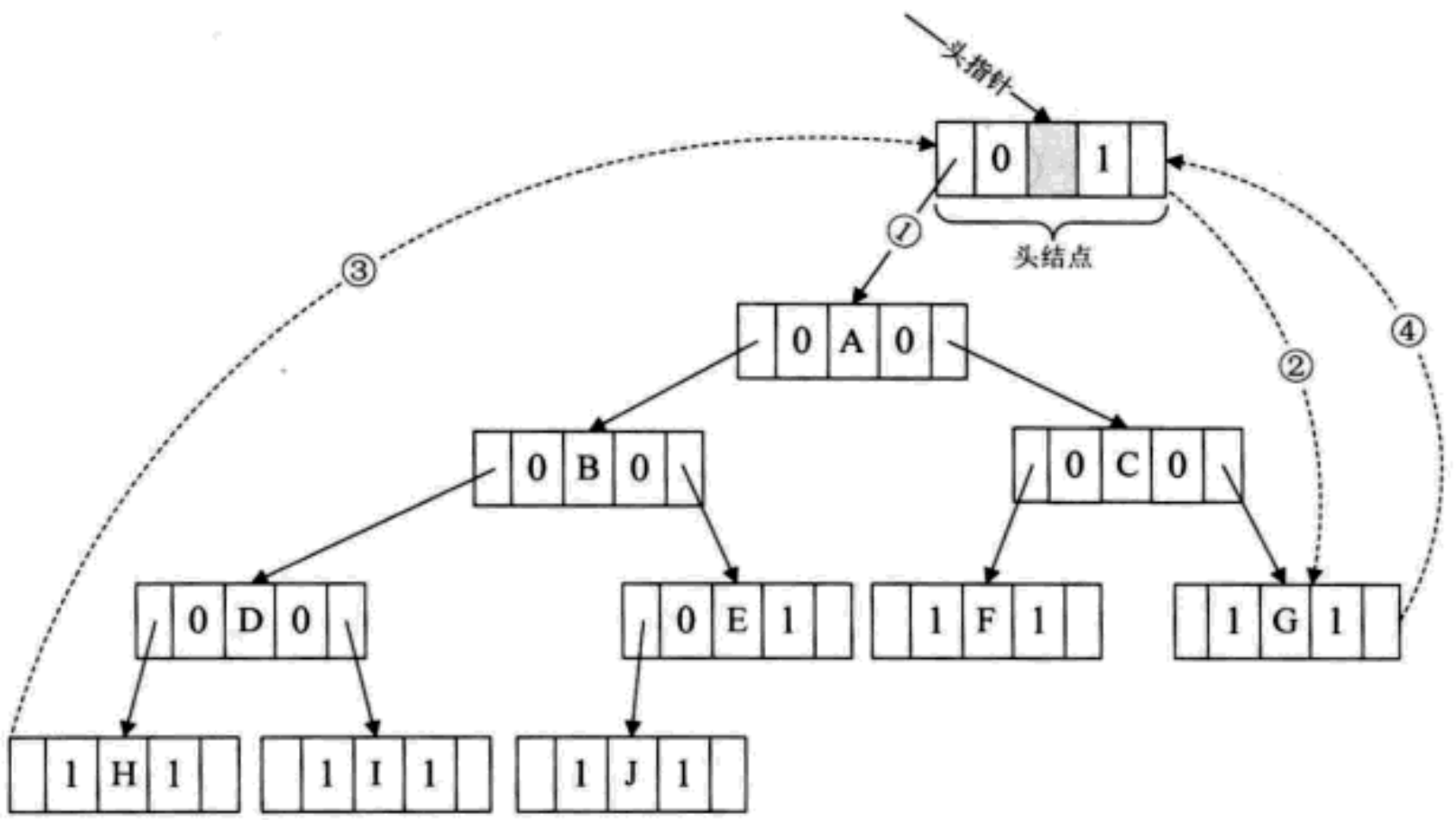﻿ 当初我要是这么学习二叉树就好了

### 当初我要是这么学习二叉树就好了

2021-11-22 2:21:52

### 目录

• 1. 树形结构
• 1.1 概念(了解)
• 1.2 概念(重要)
• 1.3 树的表示形式
• 1.4 树的应用
• 2. 二叉树(BinaryTree重点)
• 2.1 概念
• 2.2 二叉树的5种基本形态
• 2.3 两种特殊的二叉树
• 2.3.1 斜树
• 2.3.2 满二叉树
• 2.3.3 完全二叉树
• 2.4 二叉树的性质
• 2.4.1 第i层结点个数
• 2.4.2 树的所有最大点个数
• 2.4.3 叶子结点和非叶子结点数量关系
• 2.4.4 根据结点求树深度
• 2.4.5 父子结点编号关系
• 2.4.6 小练兵
• 2.5 二叉树的存储
• 2.5.1 顺序存储
• 2.5.1.1 完全二叉树的形式存储
• 2.5.1.2 一般树的形式存储
• 2.5.2 链式存储
• 3. 二叉树由浅入深解析
• 3.1 遍历二叉树
• 3.1.1 二叉树遍历原理
• 3.1.2 二叉树遍历方法
• 3.1.2.1 前序遍历
• 3.1.2.2 中序遍历
• 3.1.2.3 后序遍历
• 3.1.2.4 层序遍历
• 3.1.3 二叉树遍历算法
• 3.1.3.1 前序遍历算法根左右
• 3.1.3.2 中序遍历算法左根右
• 3.1.3.2 后序遍历算法左右根]
• 3.1.3.2 层序遍历算法上至下, 左至右
• 3.2 二叉树建立问题
• 3.2.1 前序中序构建二叉树
• 3.2.2 中序后序构建二叉树
• 3.2.3 根据二叉树创建字符串
• 3.2.4 前序遍历字符串构建二叉树
• 3.2.5 合并二叉树
• 3.2.6 递增二叉树
• 3.3 经典题目解析
• 3.3.1 结点个数
• 3.3.2 叶子结点个数
• 3.3.3 第k层结点个数
• 3.3.4 查找 val 所在结点
• 3.3.5 相同的树
• 3.3.6 另一棵树子树
• 3.3.7 二叉树最大深度
• 3.3.8 平衡二叉树
• 3.3.9 对称二叉树
• 3.3.10 二叉树的完全性检验
• 3.3.11 二叉树最近公公祖先
• 3.3.12 二叉树最大宽度
• 3.4. 特殊的二叉树问题
• 3.4.1 搜索二叉树
• 3.4.2 赫夫曼树及其应用
• 3.4.1. 赫夫曼树
• 3.4.2.赫夫曼树定义与原理
• 3.4.3 赫夫曼编码
• 3.4.3 线索二叉树
• 4. 总结回顾
• 5. 结束语

# 1. 树形结构

## 1.1 概念(了解)

• 有一个特殊的节点, 称为根节点, 根节点没有前驱节点
• 除根节点外, 其余节点被分成M(M > 0)个互不相交的集合T1, T2, …, Tm，其中每一个集合 <= m) 又是一棵与树类似的子树。每棵子树的根节点有且只有一个前驱，可以有0个或多个后继
• 树是递归定义的[树的定义中用到了树的概念]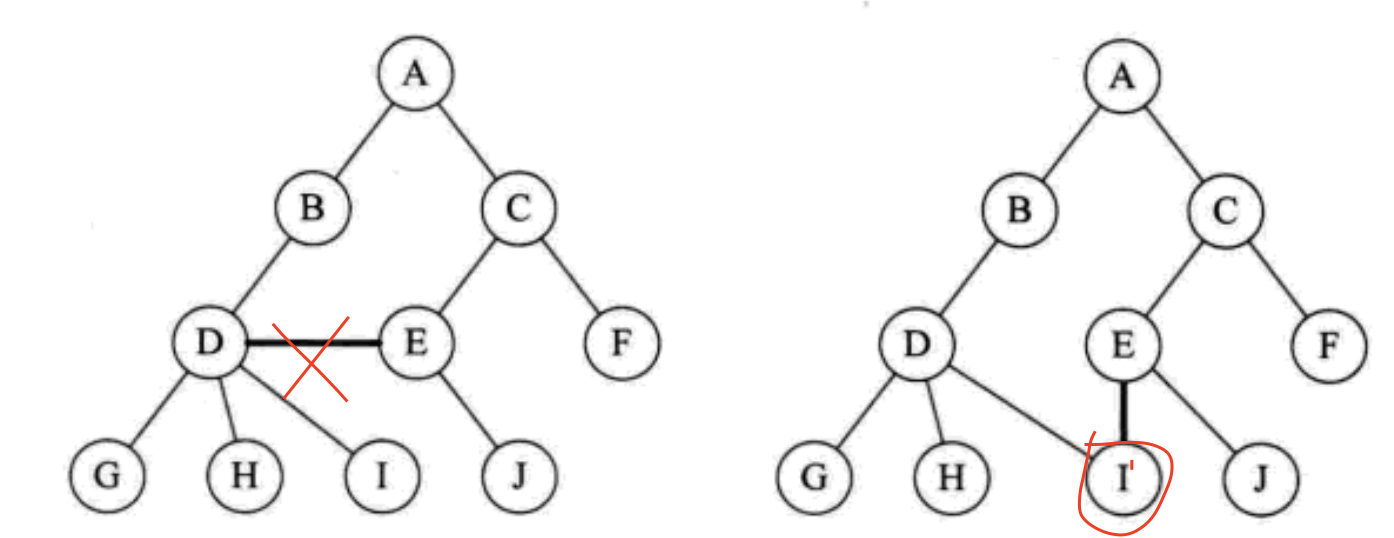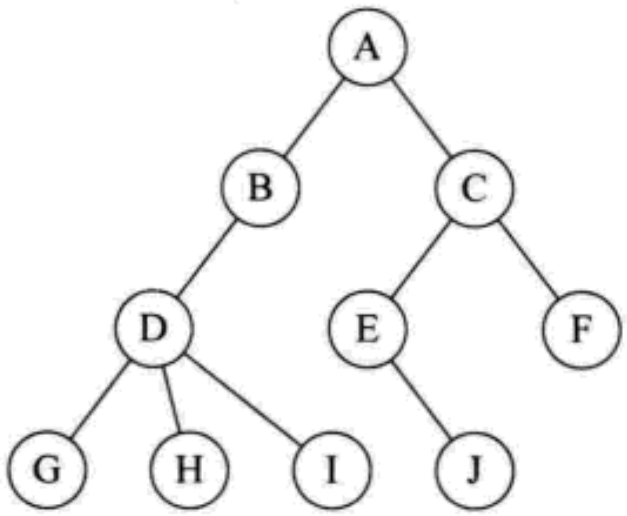## 1.2 概念(重要)

1. 节点的度: 一个节点含有的子树的个数称为该节点的度[A的度就是2]

2. 非终端节点或分支节点:度不为0的节点[A, B, C, D, E]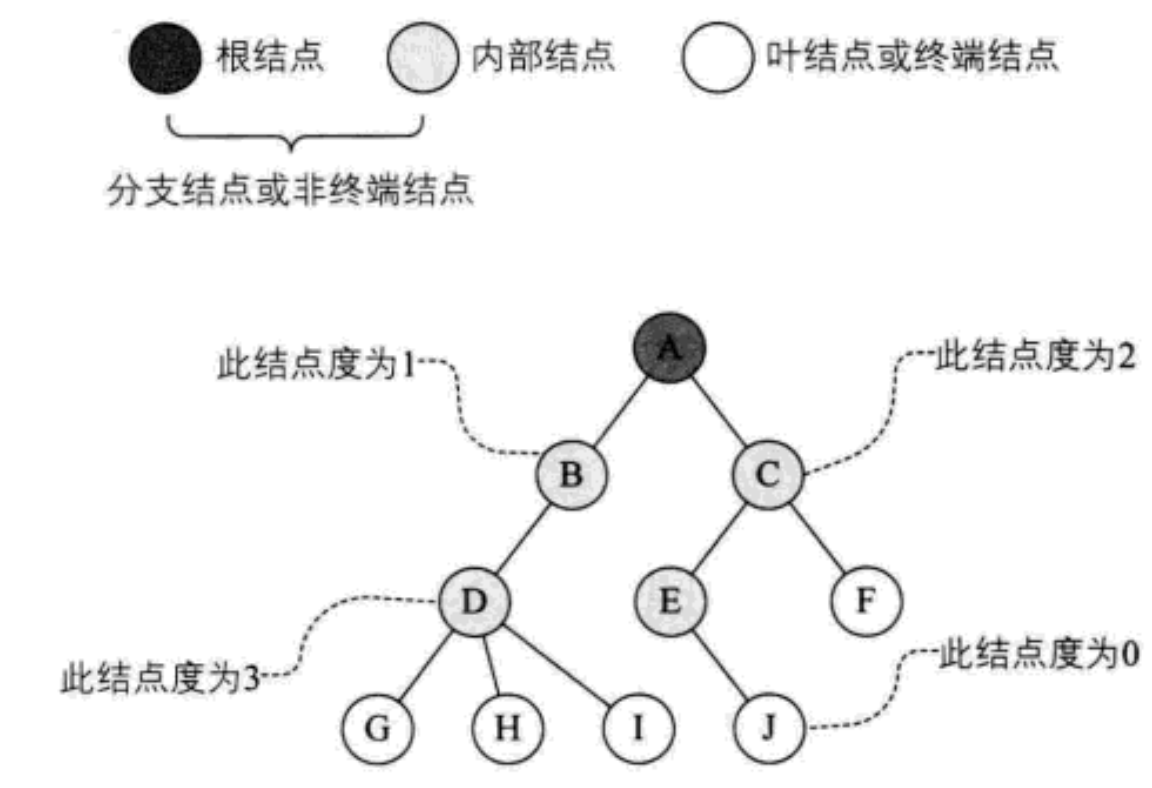3. 树的度: 一棵树中，最大的节点的度称为树的度; 如上图:树的度为3

4. 叶子节点或终端节点: 度为0的节点称为叶节点; 如上图:G、H、I、J节点为叶节点

5. 双亲节点或父节点: 若一个节点含有子节点，则这个节点称为其子节点的父节点; 如上图:A是B的父节点

6. 孩子节点或子节点: 一个节点含有的子树的根节点称为该节点的子节点; 如上图:B是A的孩子节点

7. 兄弟节点: 具有相同父节点的节点互称为兄弟节点[B和C, E和F, G, H和I]

8. 堂兄弟节点: 双亲在同一层的节点互称为堂兄弟节点[D和E, D和F]

9. 节点的祖先: 从根到该节点的所有分支经历所有节点[A]

10. 根结点: 一棵树中，没有双亲结点的结点;如上图:A

11. 森林: 由m(m>=0)棵互不相交的树的集合称为森林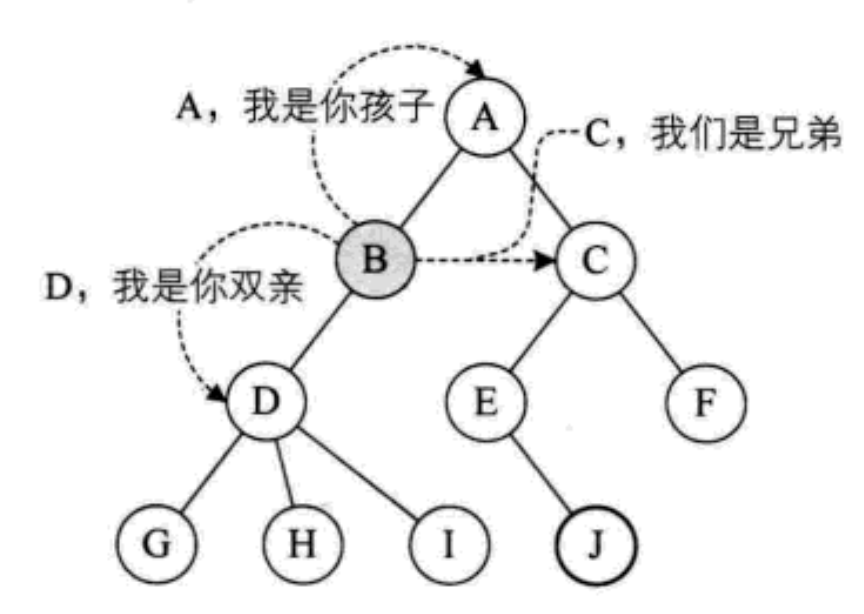12. 节点的层次: 从根开始定义起，根为第1层，根的子节点为第2层，以此类推

13. 树的高度或深度: 树中节点的最大层次; 如上图:树的高度为4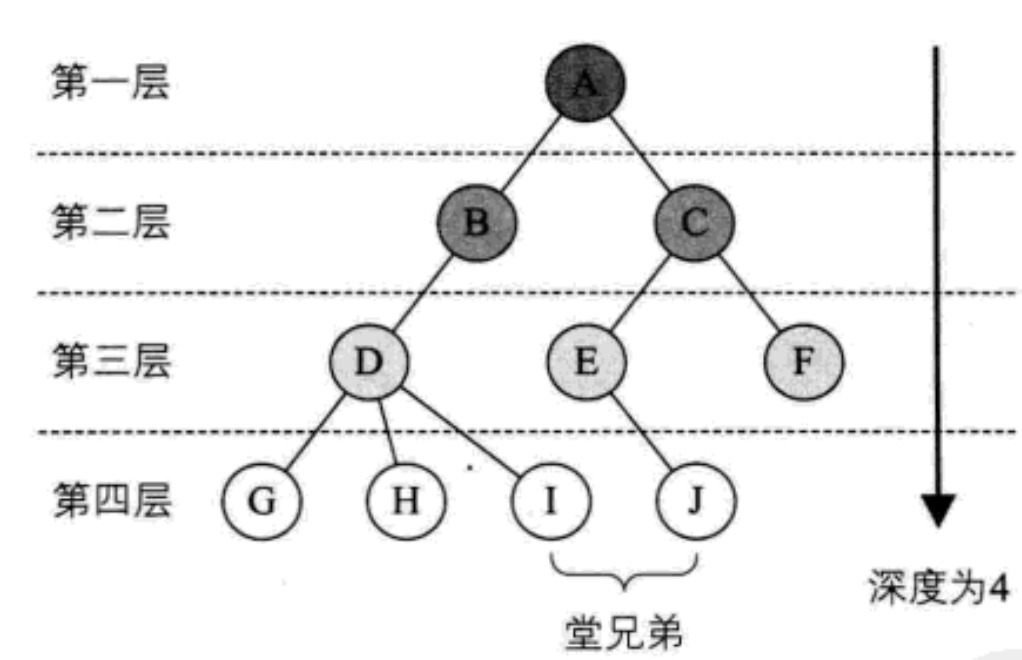## 1.3 树的表示形式

``````class Node {
int value;// 树中存储的数据
Node child;// 第一个孩子引用
Node brother;// 下一个兄弟引用
}
``````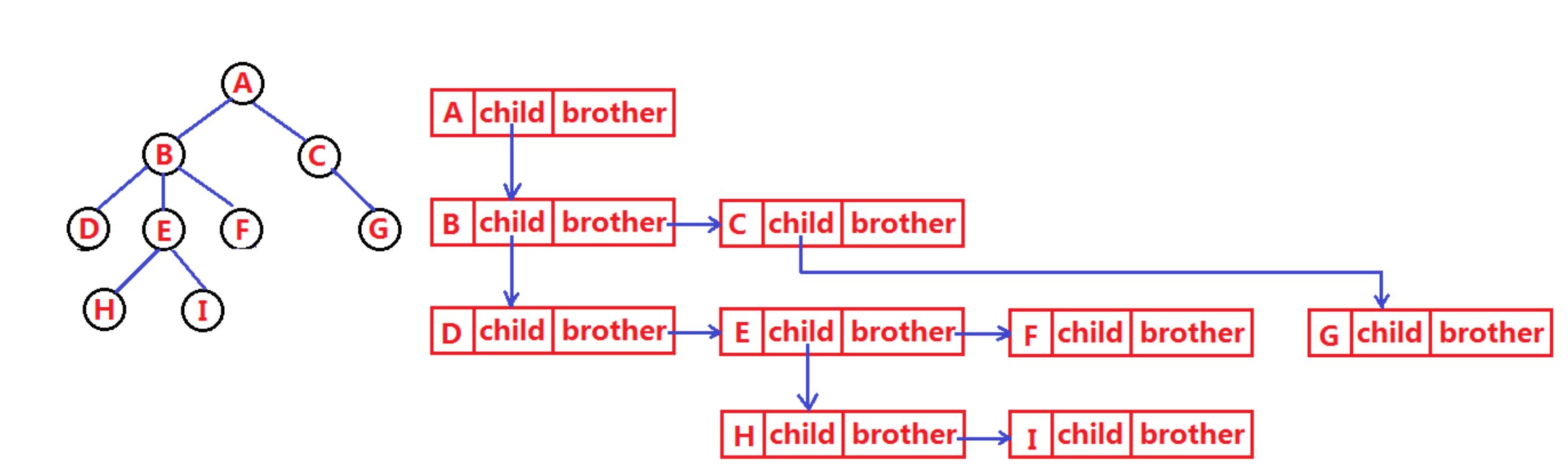## 1.4 树的应用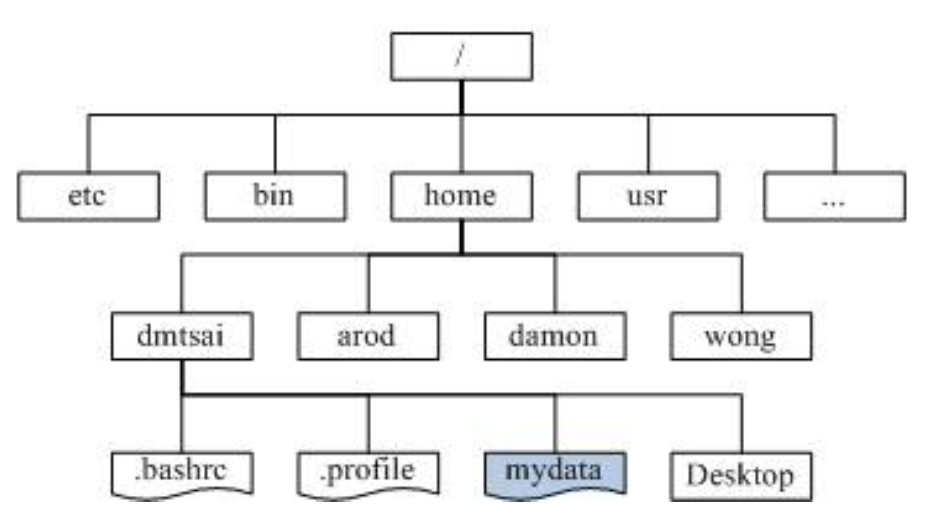# 2. 二叉树(BinaryTree重点)

## 2.1 概念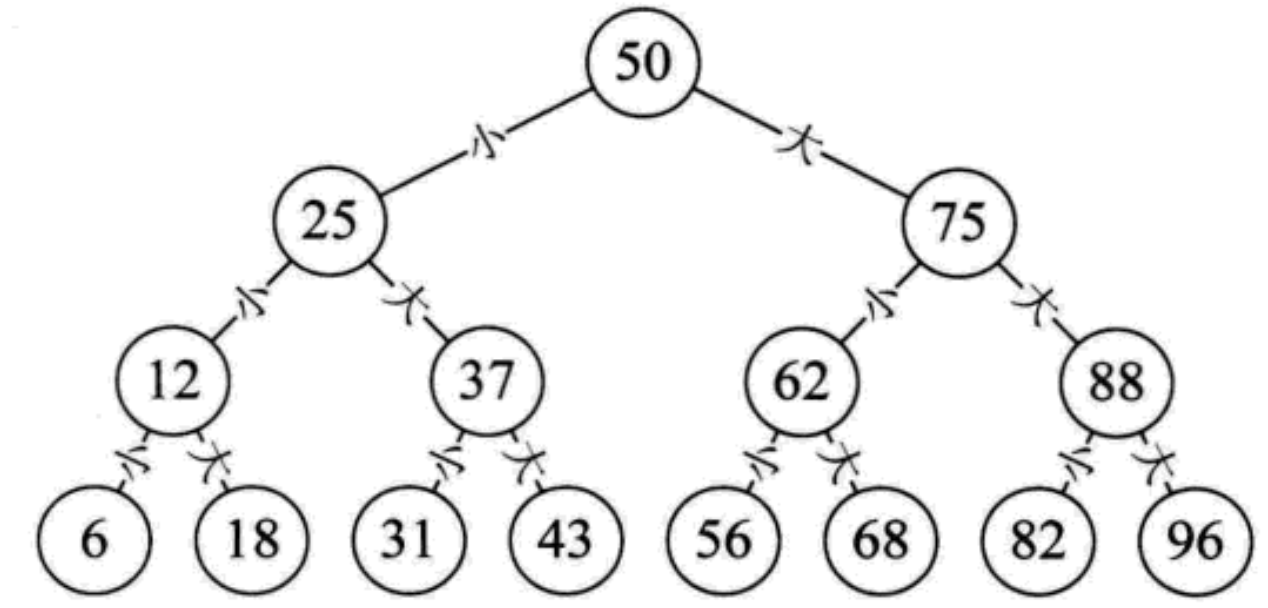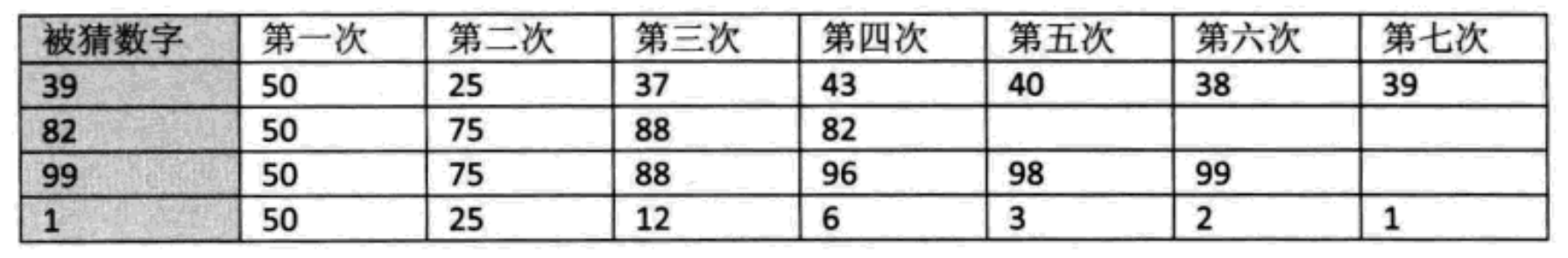1. 每个节点最多有2颗子树, 即不存在度大于2的节点
2. 二叉树有左右子树之分, 次序不能颠倒, 因此二叉树也是一颗有序树

## 2.2 二叉树的5种基本形态

1. 空二叉树
2. 只有一个根节点
3. 根节点只有左子树
4. 根节点只有右子树
5. 根节点即有左子树又有右子树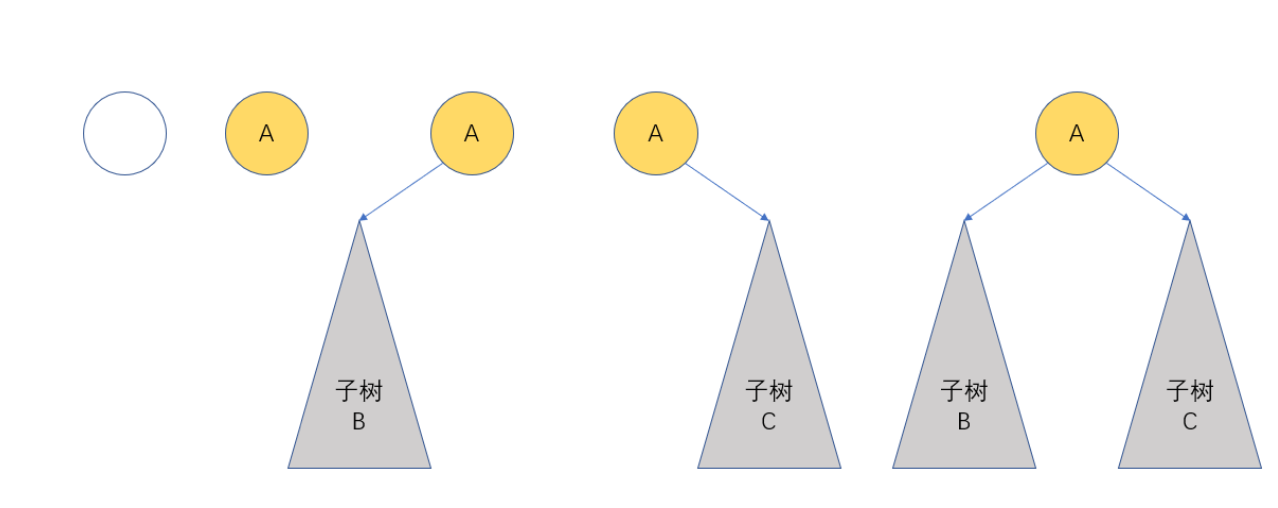## 2.3 两种特殊的二叉树

### 2.3.1 斜树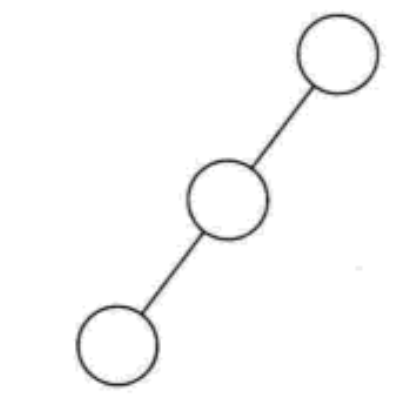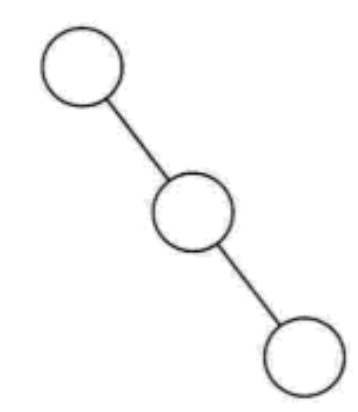### 2.3.2 满二叉树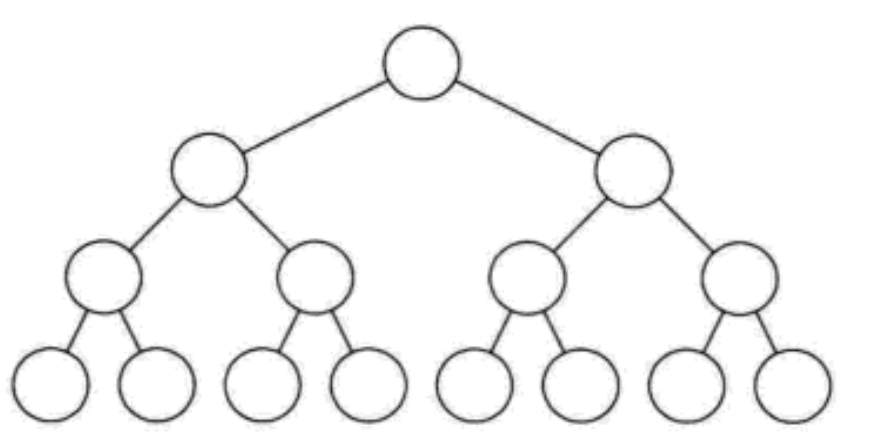1. 叶子结点只能出现在最下一层, 出现在其它层就不可能达到平衡
2. 非叶子结点的度一定是2, 否贼就是"缺胳膊少腿"
3. 在同样深度的二叉树中, 满二叉树结点个数最多, 叶子结点最多

### 2.3.3 完全二叉树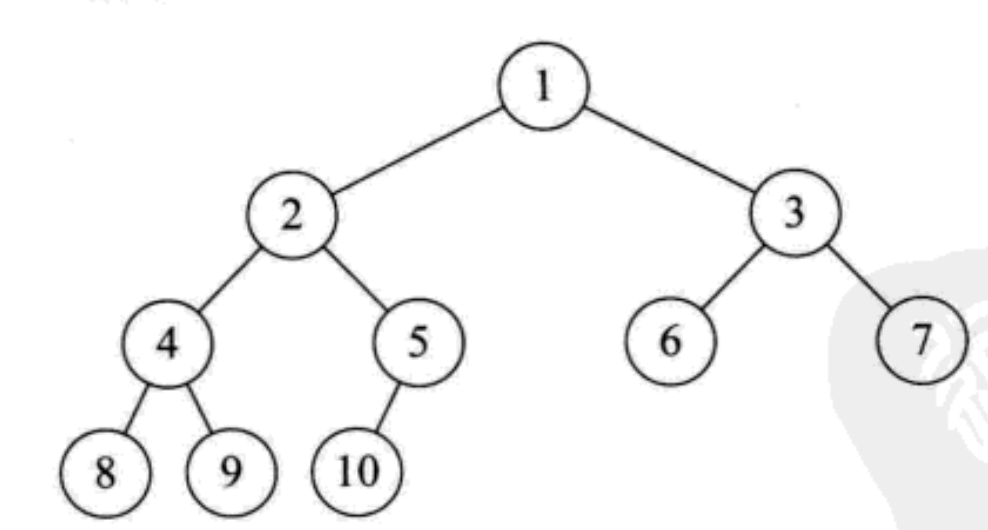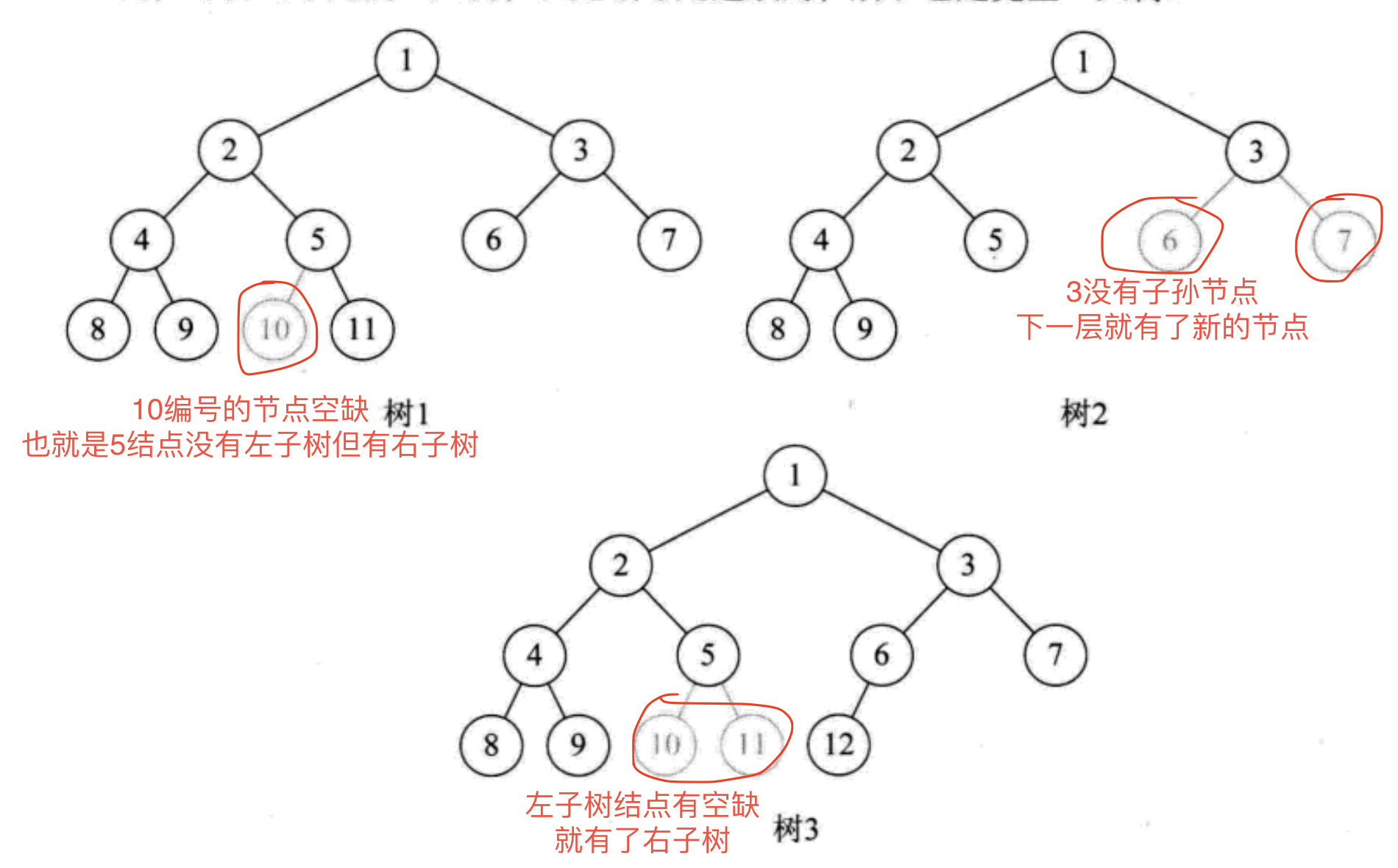1. 叶子结点只能出现在最下两层
2. 最下层的叶子结点一定集中在左部连续位置
3. 倒数第二层, 如果有叶子结点, 一定都在右部连续位置
4. 如果结点的度为1, 则该节点只有左孩子, 即不存在只有右子树的情况
5. 同样结点数的二叉树, 完全二叉树的深度最小

## 2.4 二叉树的性质

### 2.4.5 父子结点编号关系

• 若i>0，双亲序号:(i-1)/2;
• 若i=0，i为根节点编号，无双亲节点
• 若2i+1<n，左孩子序号:2i+1，否则无左孩子
• 若2i+2<n，右孩子序号:2i+2，否则无右孩子
这个规律很重要, 涉及到堆排, 二叉搜索树需要用到这个规律

### 2.4.6 小练兵

500个叶子结点计算步骤

1. 叶子结点总个数=第10层叶子结点个数+第9层叶子结点个数
有同学会想: 为何第9层会有叶子结点呢?
解释: 因为我们发现此树共有 1000 个结点, 而满二叉树的话会有 2^10-1 个结点, 所以说明 第10层没满, 第9层一定有叶子结点

1. 第10层全是叶子结点个数: 树的总结点个数 - 9层及以上所有结点个数
因为是一颗完全二叉树, 所以可以保证第9层一定是满二叉树
算式: 1000-(2^9-1)–>1000-511=489

1. 第9层叶子结点个数: 第9层结点个数 - 第10层父节点个数
算式: 2^(9-1)-第10层父节点个数

1. 第10层父节点个数求法
如果结点个数n是偶数, 则其父结点个数为 n/2
如果结点个数n是奇数, 则其父结点个数n/2+1
在第一步中我们求得了第10层父节点个数, 求第10层父节点个数就是: 489/2 + 1–>245
2. 现在可以计算第 3 步得出第9层叶子结点个数:
2^(9-1)-245–>256-245=11
3. 现在可以计算第 1 步中的所有整棵树的叶子结点;
10 层结点数 +9 叶子结点数: 489+11 = 500
4. 有了叶子结点个数, 很容易知道非叶子节点
总结点个数-叶子结点个数: 1000-500
5. 由于完全二叉树第10层结点个数为489, 为奇数, 所以一定有1个节点只有左孩子, 有 0 个节点只有右子树

n个节点的完全二叉树，最多可以有多少层: log(n+1)上取整

## 2.5 二叉树的存储

### 2.5.1 顺序存储

#### 2.5.1.1 完全二叉树的形式存储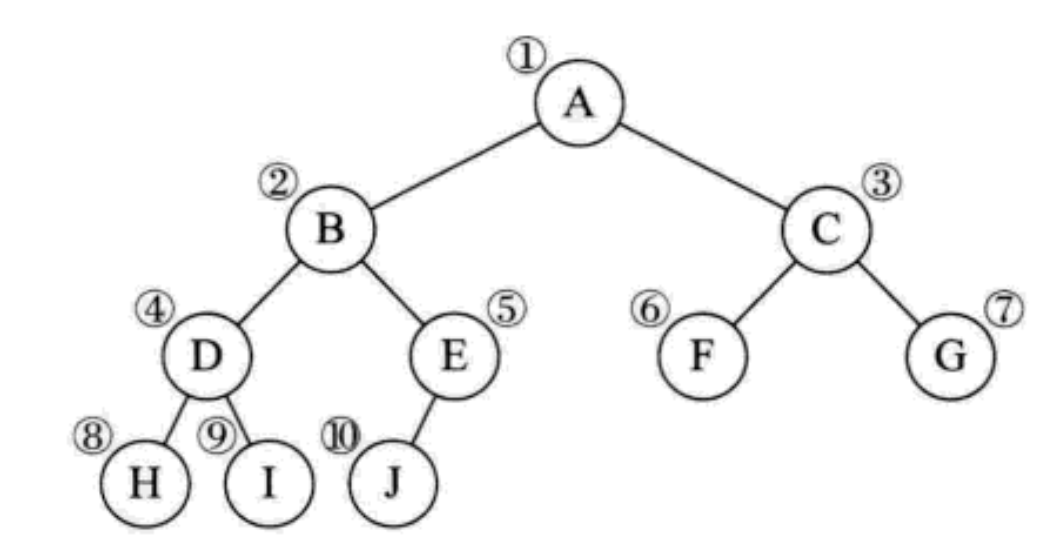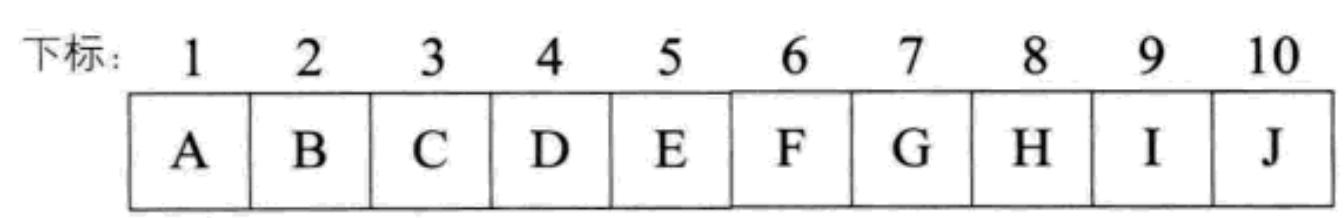#### 2.5.1.2 一般树的形式存储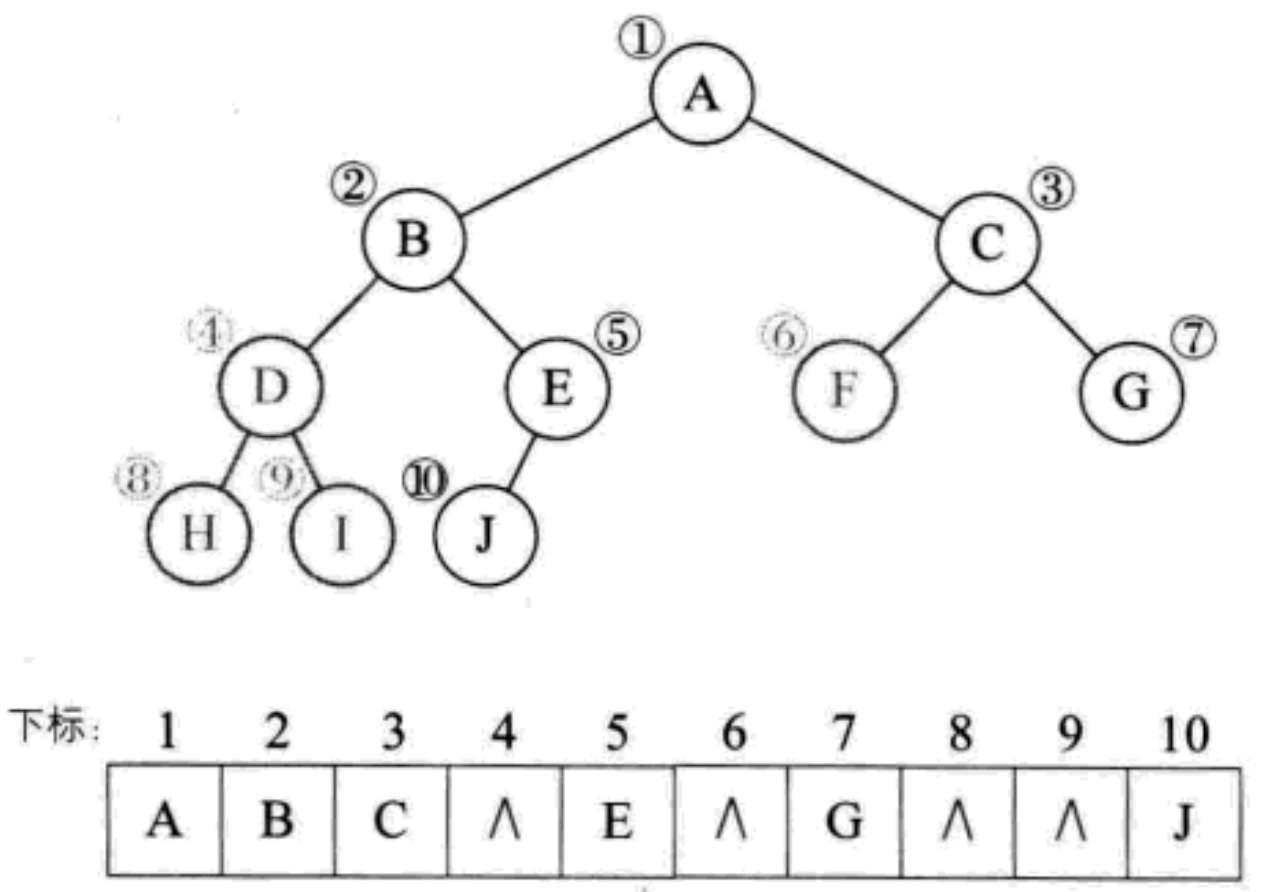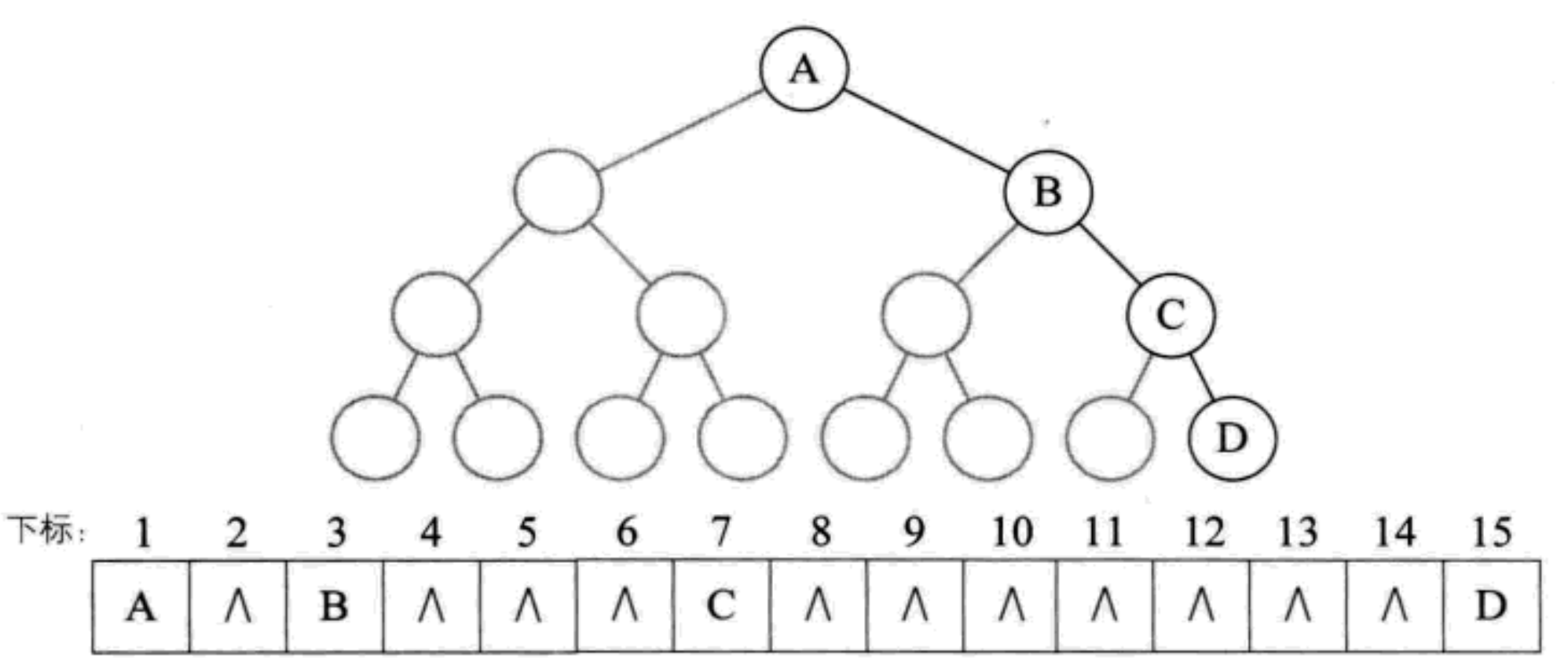### 2.5.2 链式存储``````class Node {
int val;// 数据域
Node left;// 左孩子的引用，常常代表左孩子为根的整棵左子树
Node right;// 右孩子的引用，常常代表右孩子为根的整棵右子树
}
``````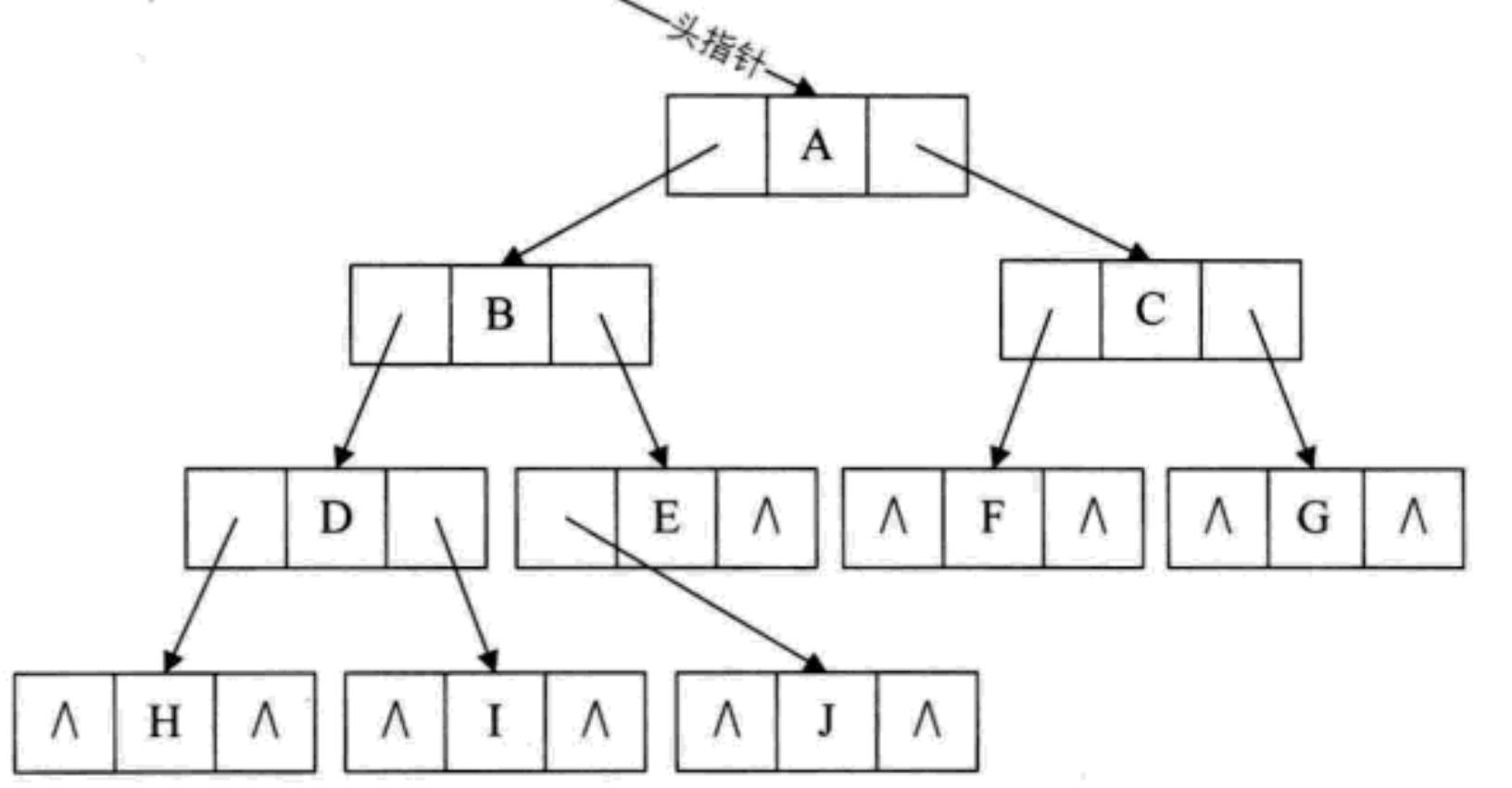# 3. 二叉树由浅入深解析

## 3.1 遍历二叉树

### 3.1.2 二叉树遍历方法

#### 3.1.2.1 前序遍历

1. 若二叉树为空, 则返回空操作
2. 否则先访问根节点, 然后前序遍历左子树再前序遍历右子树
3. 最终访问的结果就是: ABDGHCEIF
如下图就是 根左右 的顺序访问二叉树示意图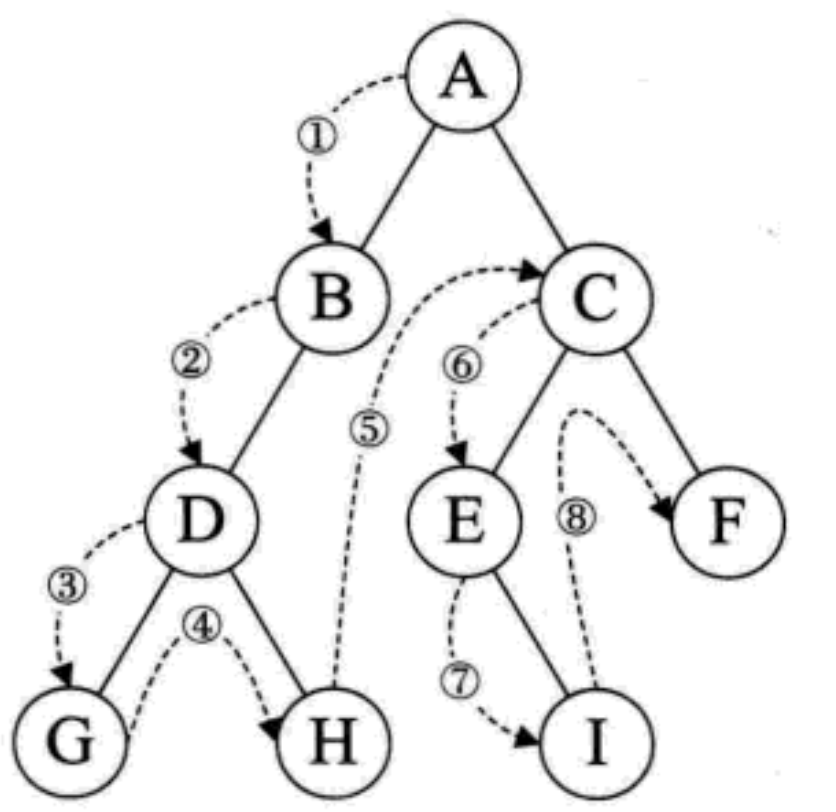#### 3.1.2.2 中序遍历

1. 树空, 则返回空操作
2. 否则就从根结点开始(注意并不是先访问根节点)
3. 中序遍历根节点的左子树
4. 然后访问根节点
5. 最后中序遍历右子树
6. 最终访问结果就是: GDHBAEICF
如下图就是 左根右 的顺序访问二叉树示意图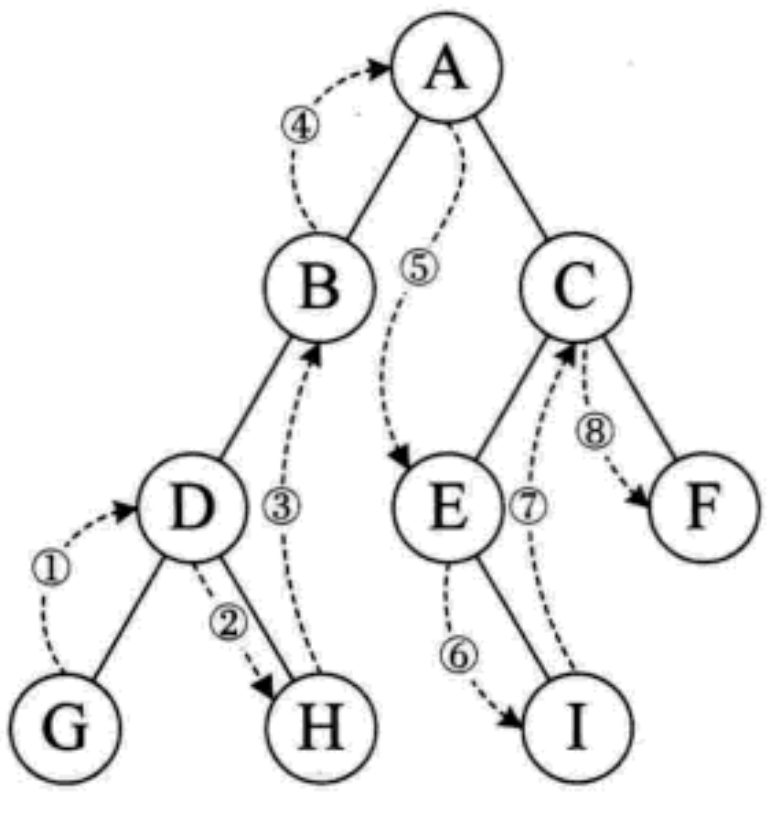#### 3.1.2.3 后序遍历

1. 树空, 则返回空操作
2. 否先从左到右线叶子结点后节点的方式遍历访问左右子树, 最后访问根节点
3. 最终访问结果是: GHDBIEFCA
如下图就是 左右根 的顺序访问二叉树示意图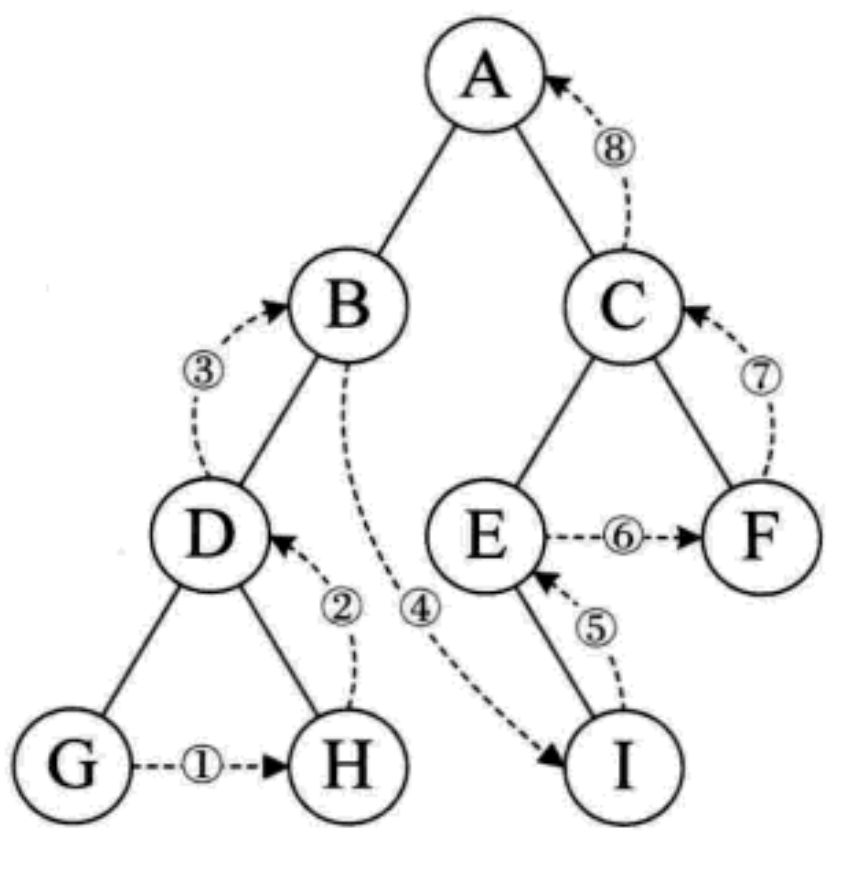#### 3.1.2.4 层序遍历

1. 若树为空, 返回空操作
2. 否则从树的第一层, 也就是根节点开始访问
3. 从上而下逐层遍历
4. 在同一层中, 按左到右的顺序对节点逐个访问
5. 最终访问结果就是: ABCDEFGHI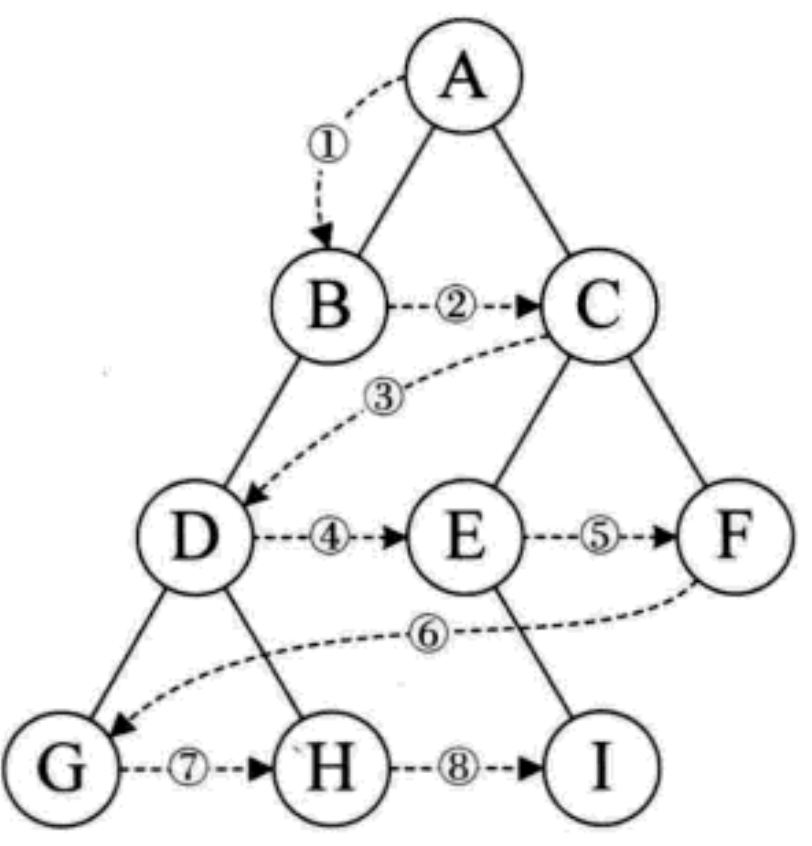### 3.1.3 二叉树遍历算法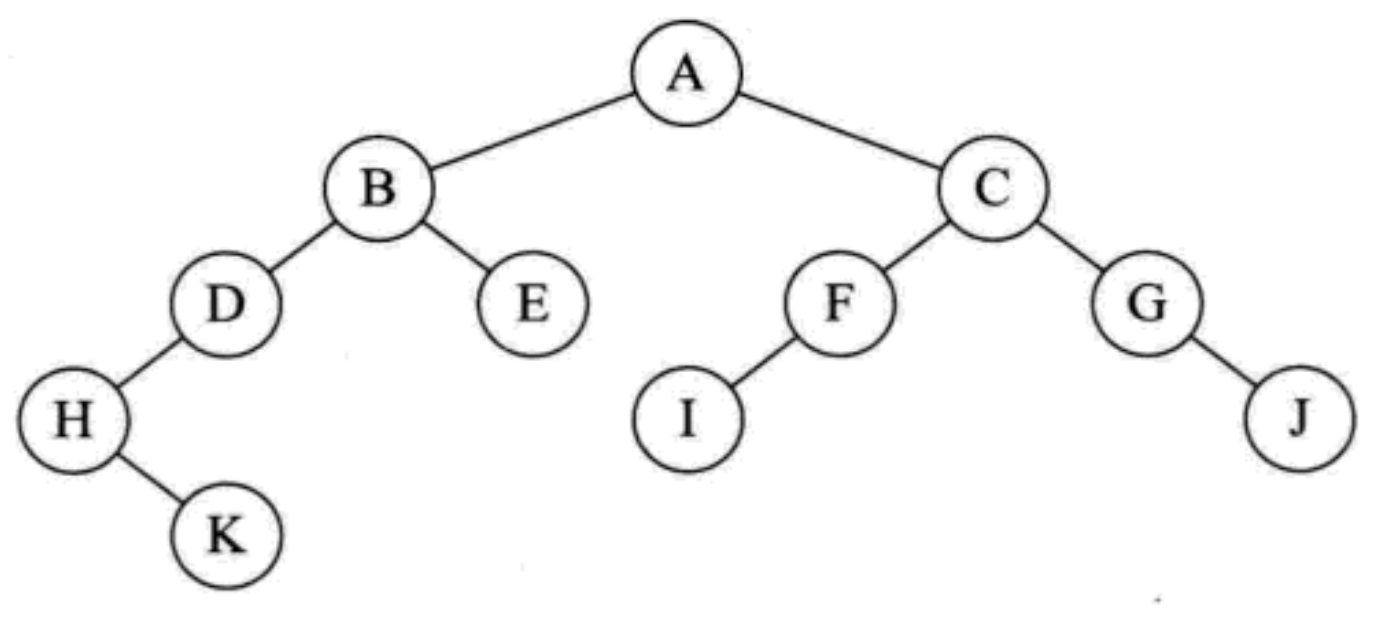#### 3.1.3.1 前序遍历算法根左右

OJ题目链接

``````private static void preOrderTraversal(BinaryTreeNode root) {
if (root == null) {
return;
} else {
System.out.print(root.val + " ");
preOrder(root.left);
preOrder(root.right);
}
}

private static List<String> preOrderTraversal(BinaryTreeNode root) {
List<String> result = new ArrayList<>();
if (root == null) {
return result;
} else {
return result;
}
}

A B D H K E C F I G J
``````

1. 调用 `preOrderTraversal(BinaryTreeNode root)`, root 根节点不为空, 所以执行 `System.out.print(root.val + " ");` 打印字母 A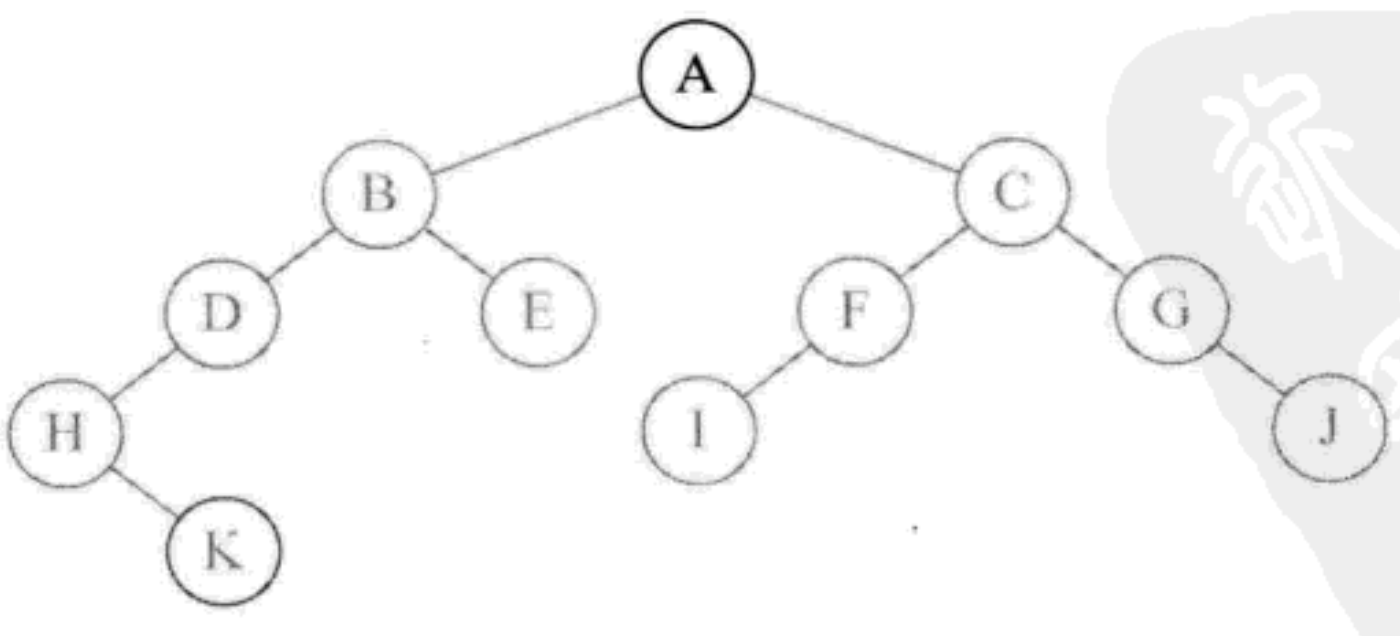2. 调用 `preOrderTraversal(root.left)`, 访问了A 节点的左孩子, 不为 null, 执行 `System.out.print(root.val + " ");` 打印字母 B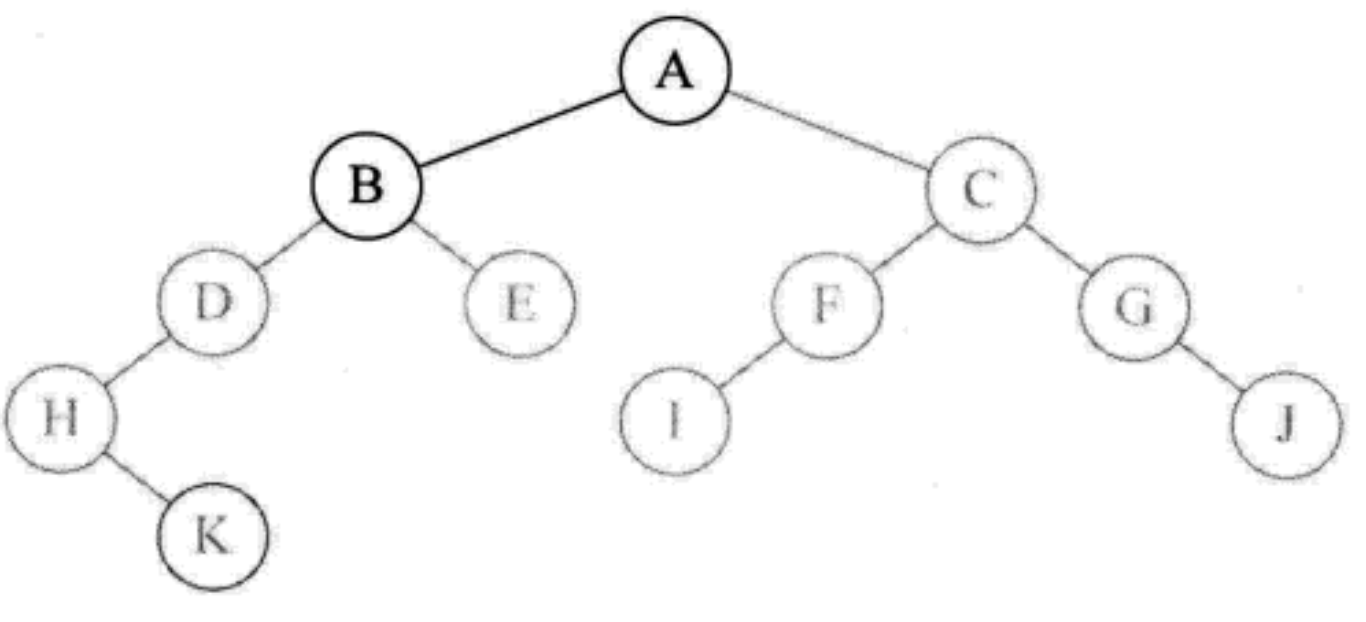3. 再次递归调用 `preOrderTraversal(root.left)`, 访问了B 节点的左孩子, 不为 null, 执行 `System.out.print(root.val + " ");` 打印字母 D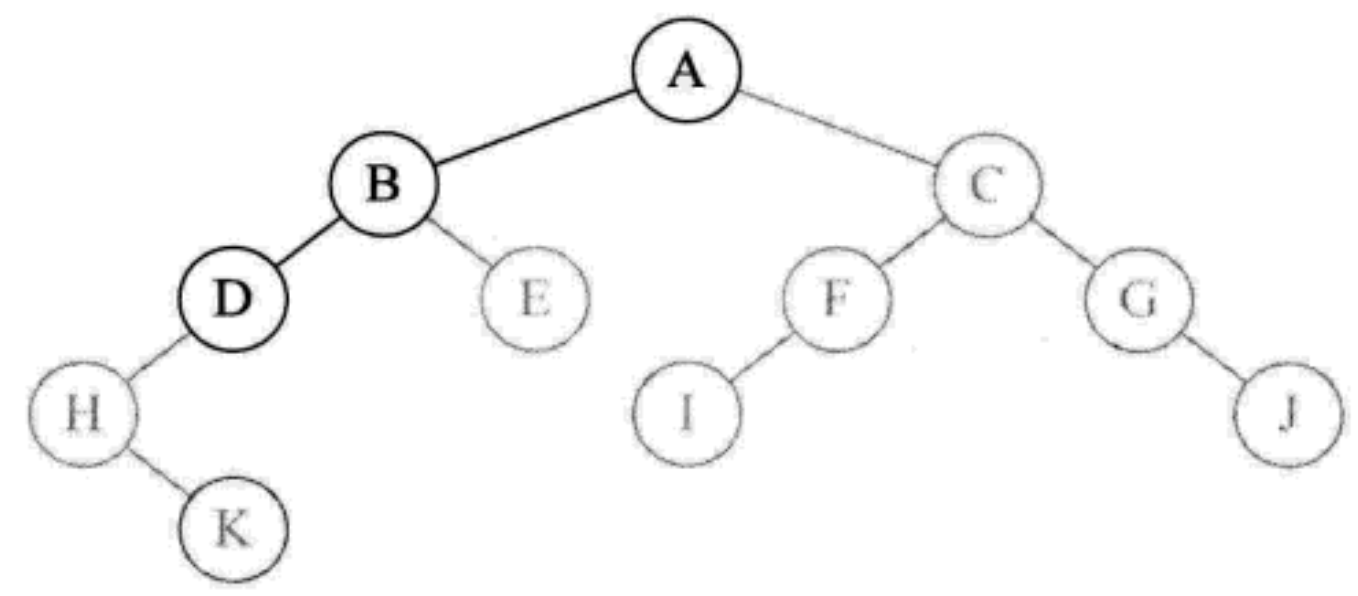4. 再次递归调用 `preOrderTraversal(root.left)`, 访问了D 节点的左孩子, 不为 null, 执行 `System.out.print(root.val + " ");` 打印字母 H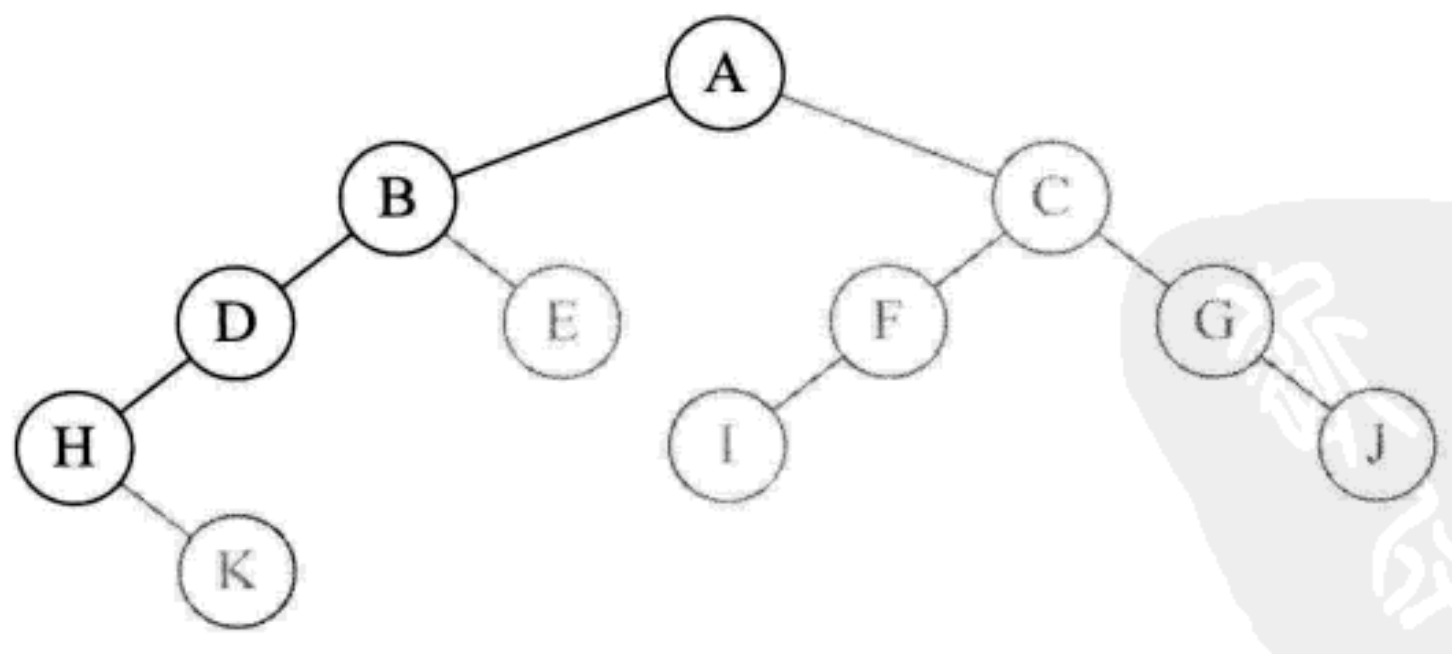5. 再次递归调用 `preOrderTraversal(root.left)`, 访问H 节点的左孩子, 此时因为 H 节点无左孩子, 所以 `root == null` 返回此函, 此时递归调用 `preOrderTraversal(root.right);` 访问了 H 节点的右孩子, 执行 `System.out.print(root.val + " ");` 打印字母 K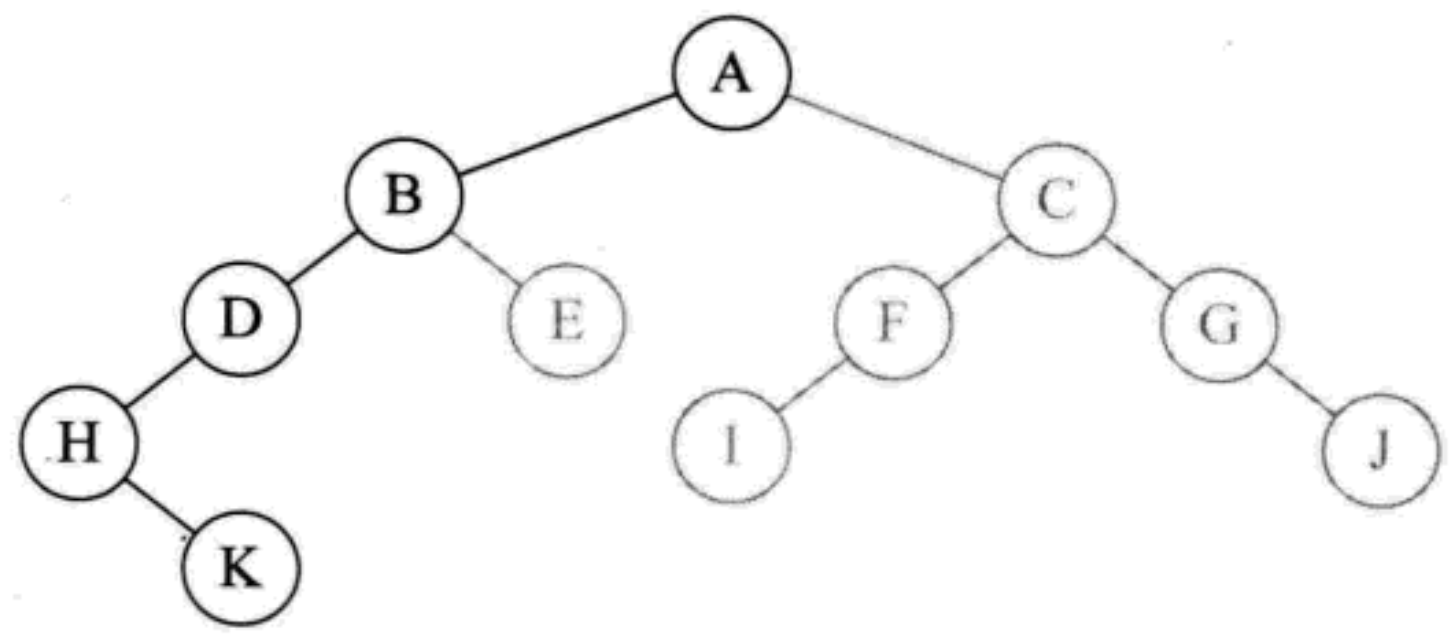6. 再次递归调用 `preOrderTraversal(root.left)`, 访问 K 节点的左孩子, 返回
调用 `preOrderTraversal(root.right)`, 访问了 K 节点的右孩子, 也是 null, 返回.于是函数执行完毕.
返回到上一级递归的函数(即打印 H 节点时的函数), 也执行完毕.[根左右都已经遍历完, 所以执行完毕]
返回打印 D 节点时的函数, 调用 `preOrderTraversal(root.ri ght)`访问 D 节点的右孩子, 不存在
返回到 B 节点, 调用 `preOrderTraversal(root.right)`, 访问 B 节点的右孩子, 打印字母 E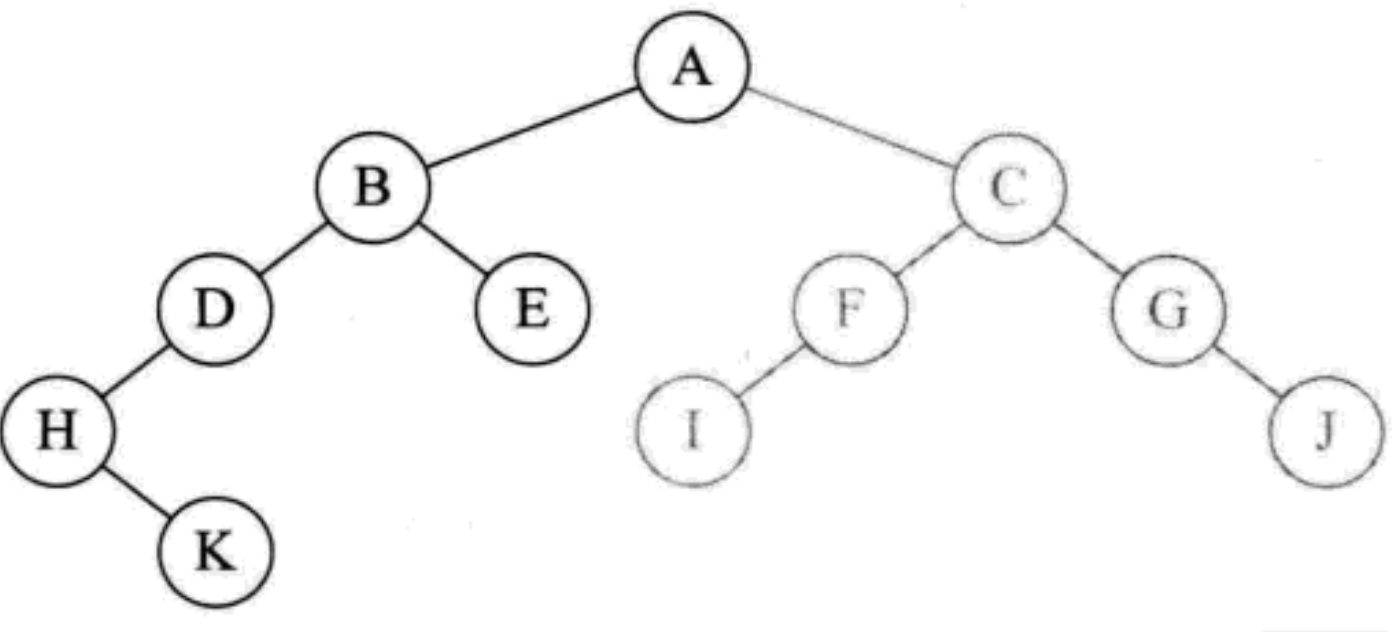7. 由于节点 E 没有左右孩子, 返回打印节点 B 时的递归函数, 递归执行完毕. 返回到最初的 `preOrderTraversal(root)`, 调用 `preOrderTraversal(root.right)`, 访问 A 节点的右孩子, 打印字母 C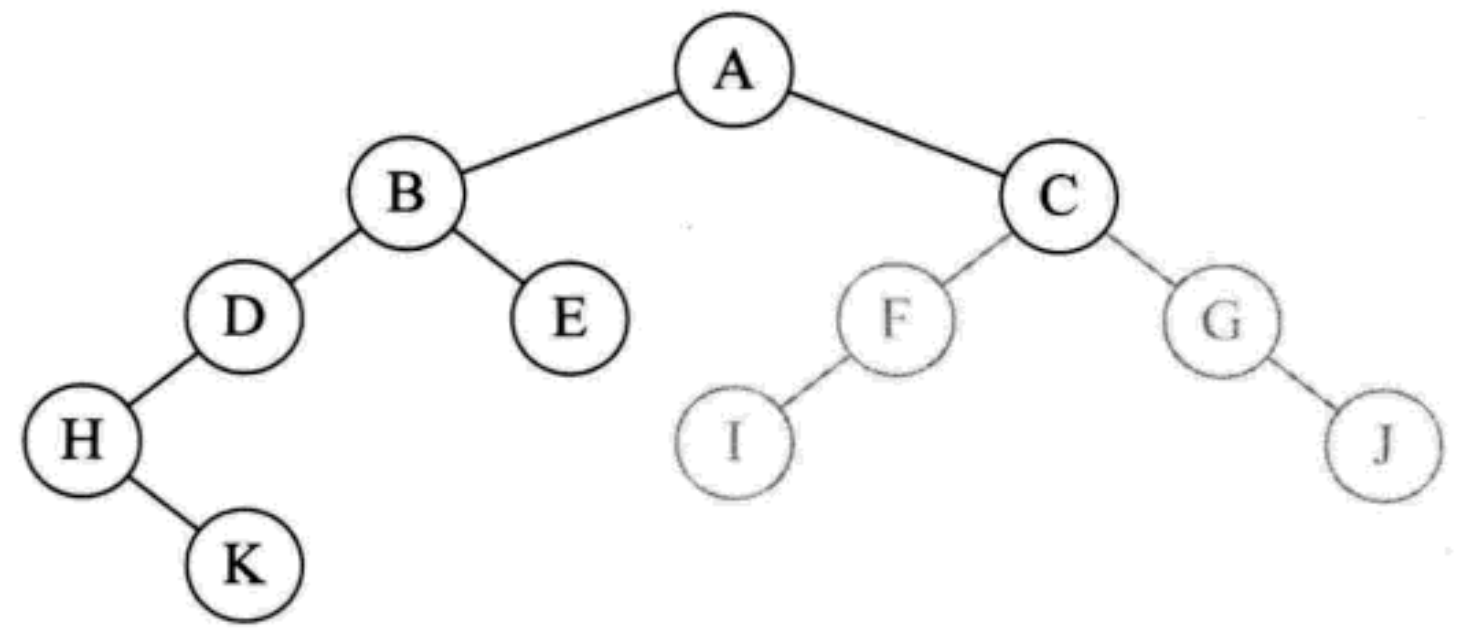8. 之后类似前面的递归调用, 一次继续打印F, I, G, j

``````/*

1.先创建一个栈
2.根节点放进栈里
3.循环取栈顶元素
4.出栈并访问这个元素值域
5.把当前元素的右子树入栈, 再左子树入栈【因为先打印左子树后打印右子树所以对于栈而言应该先入右子树再入左子树】
*/
private static void preOrderTraversalNo(BinaryTreeNode root) {
if (root == null) {
return;
} else {
Stack<BinaryTreeNode> stack = new Stack<>();
stack.push(root);
while (!stack.empty()) {
BinaryTreeNode top = stack.pop();
System.out.print(top.val + " ");
if (top.right != null) {
stack.push(top.right);
}
if (top.left != null) {
stack.push(top.left);
}
}
}
}

A B D H K E C F I G J
``````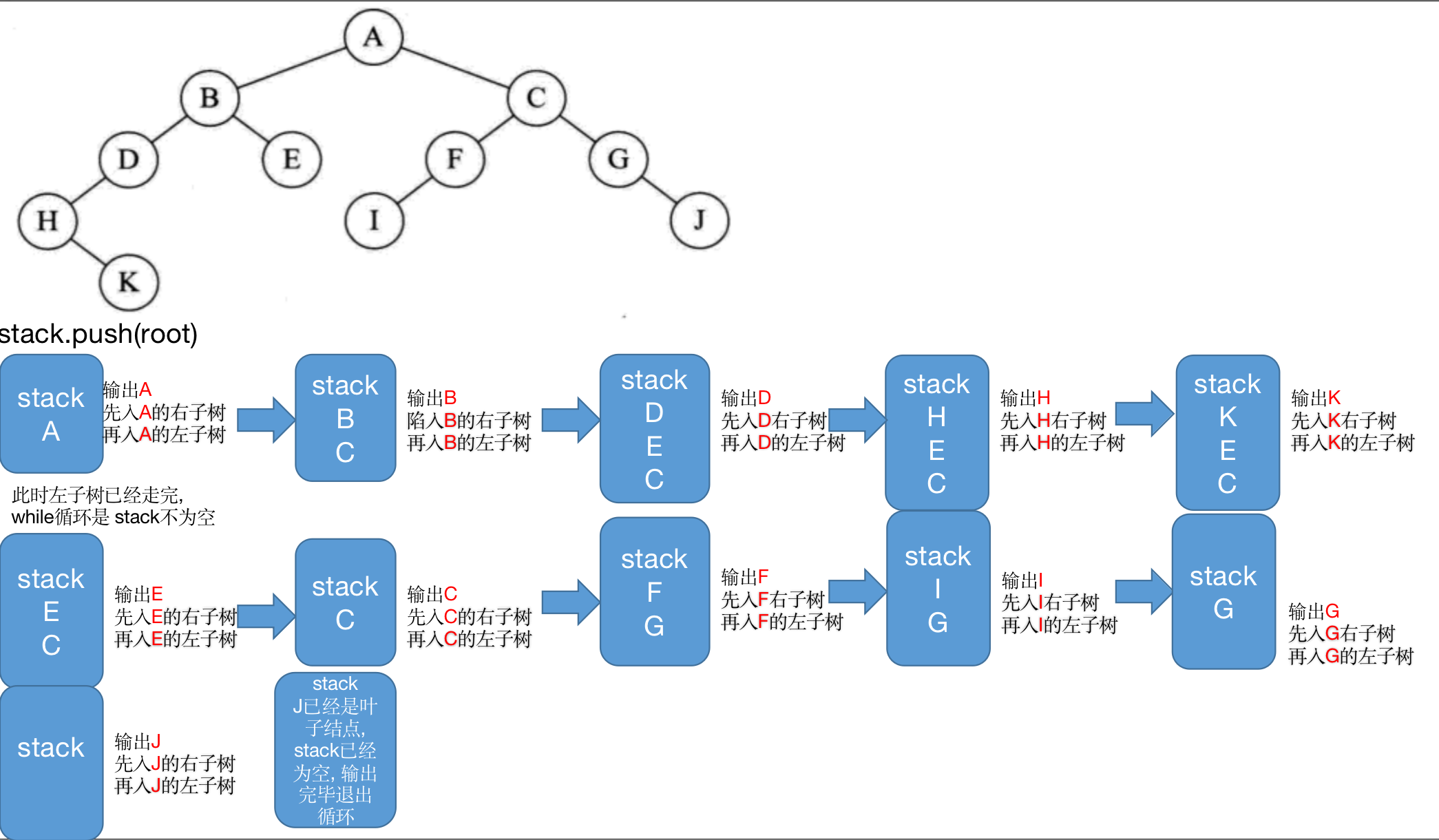#### 3.1.3.2 中序遍历算法左根右

OJ题目链接

``````private static void inOrderTraversal(BinaryTreeNode root) {
if (root == null) {
return;
} else {
preOrder(root.left);
System.out.print(root.val + " ");
preOrder(root.right);
}
}
H K D B E A I F C G J
``````

1. 调用 `inOrderTraversal(BinaryTreeNode root)`, root 的根节点 A 不为 null, 于是调用 `inOrderTraversal(root.left)`, 访问节点 B; B 不为空, 继续调用`inOrderTraversal(root.left)`, 访问节点 D; D 不为空, 继续调用`inOrderTraversal(root.left)`, 访问节点 H; H 不为空, 继续调用 `inOrderTraversal(root.left)`, 访问节点 H的左孩子; 为空, 返回. 打印当前节点 H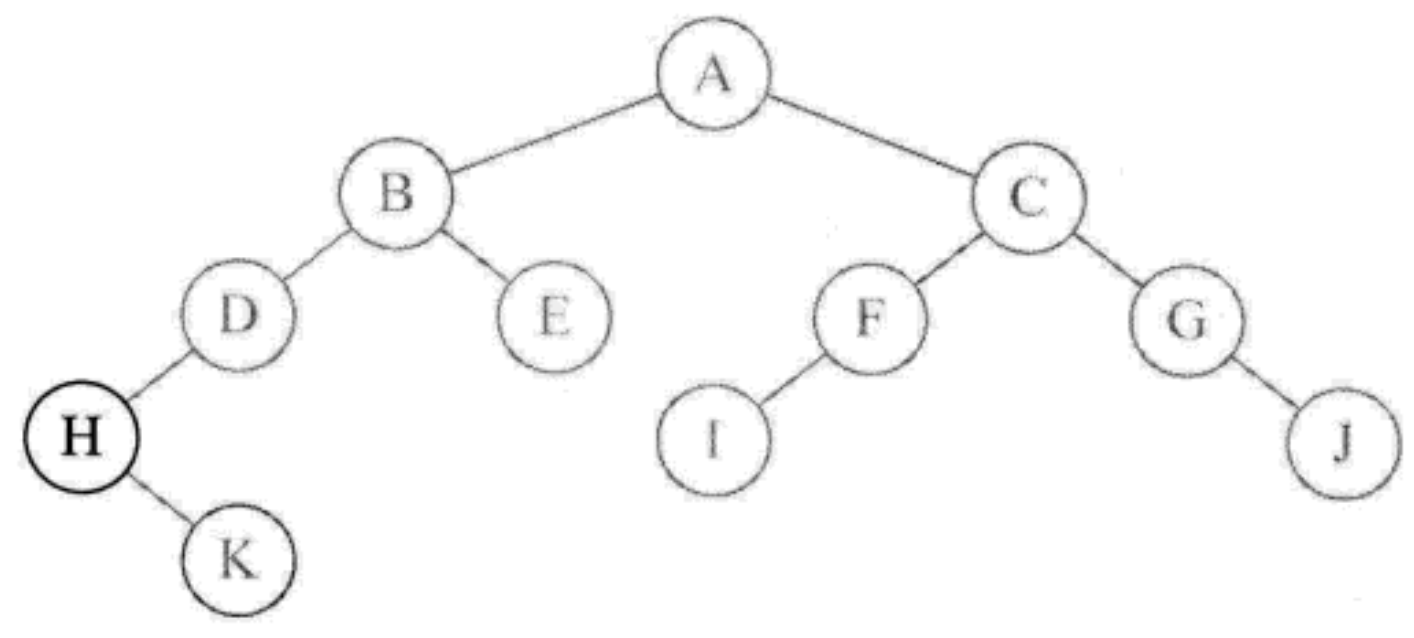2. 然后调用`inOrderTraversal(root.right)`, 访问 H 节点的右节点 K.因为 K 无左孩子, 所以打印 K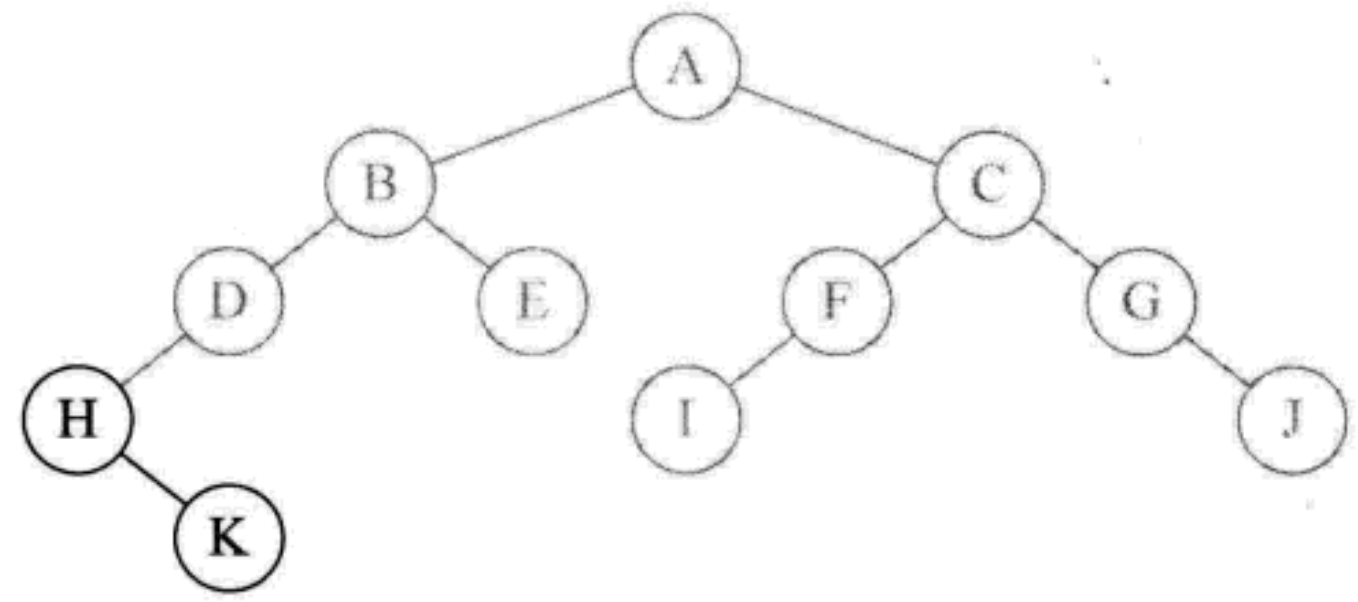3. 因为 K 没有右节点, 所以返回. 打印 H 节点的函数执行完毕, 返回. 打印字母 D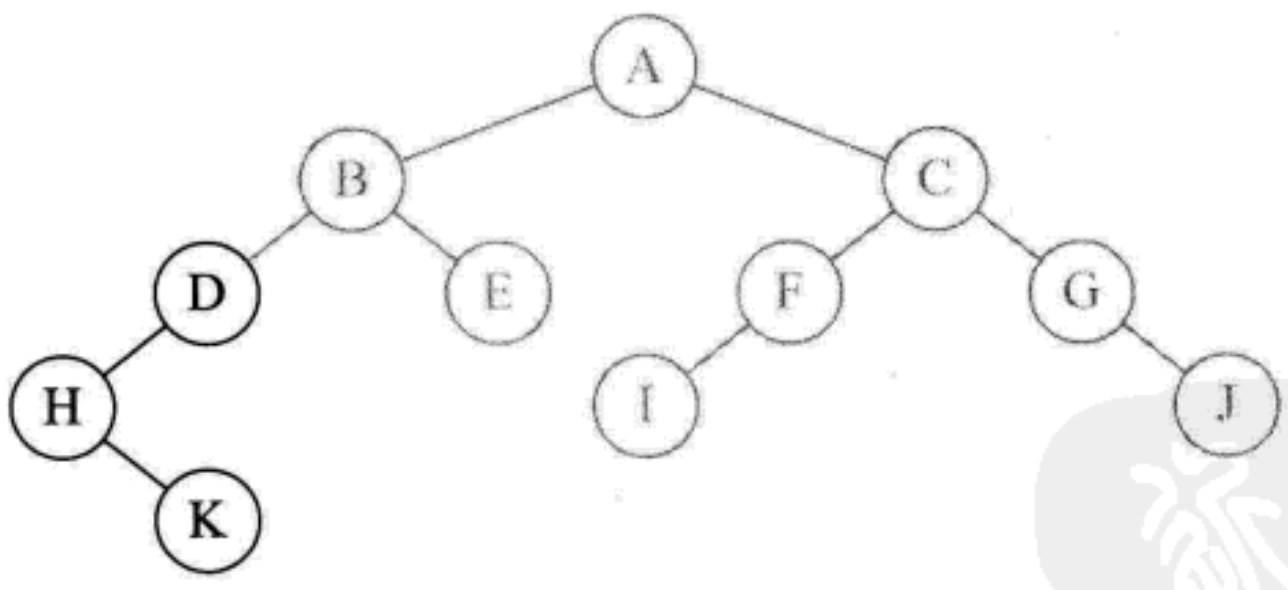4. 节点 D 没有右孩子, 所以返回. 打印 B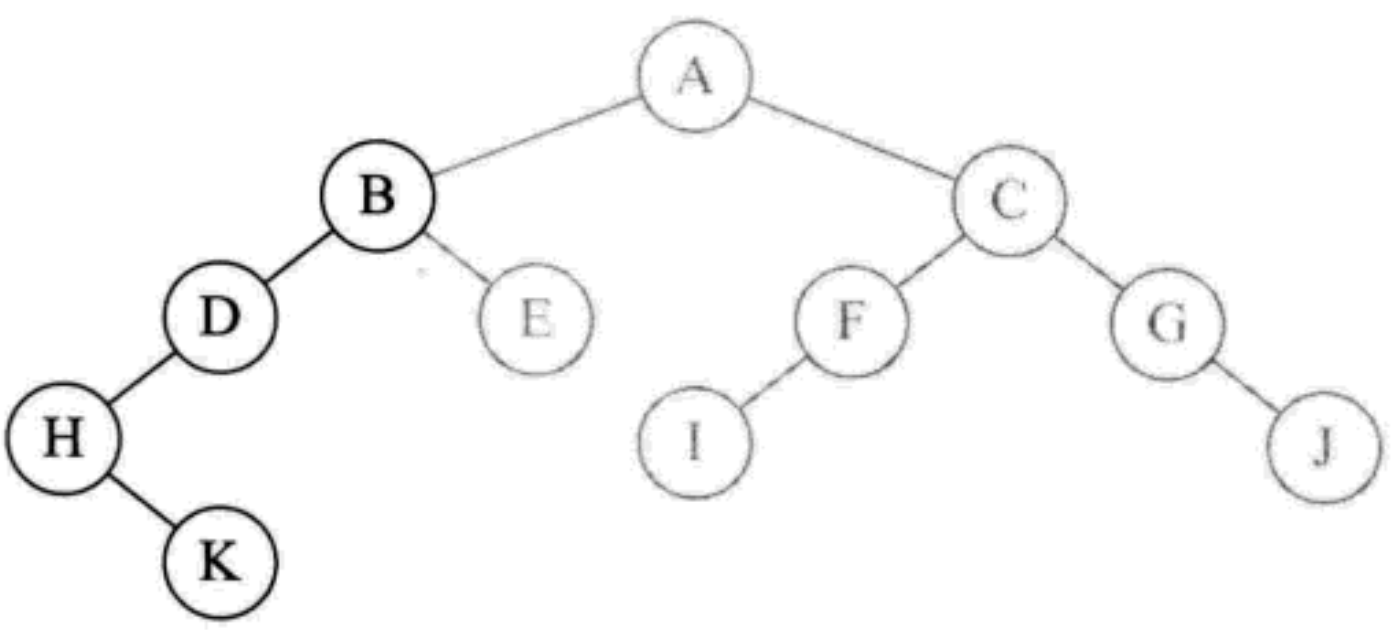5. 调用`inOrderTraversal(root.right)`, 访问 B 节点的右节点 E, 因为 E 没有左孩子, 所以打印 E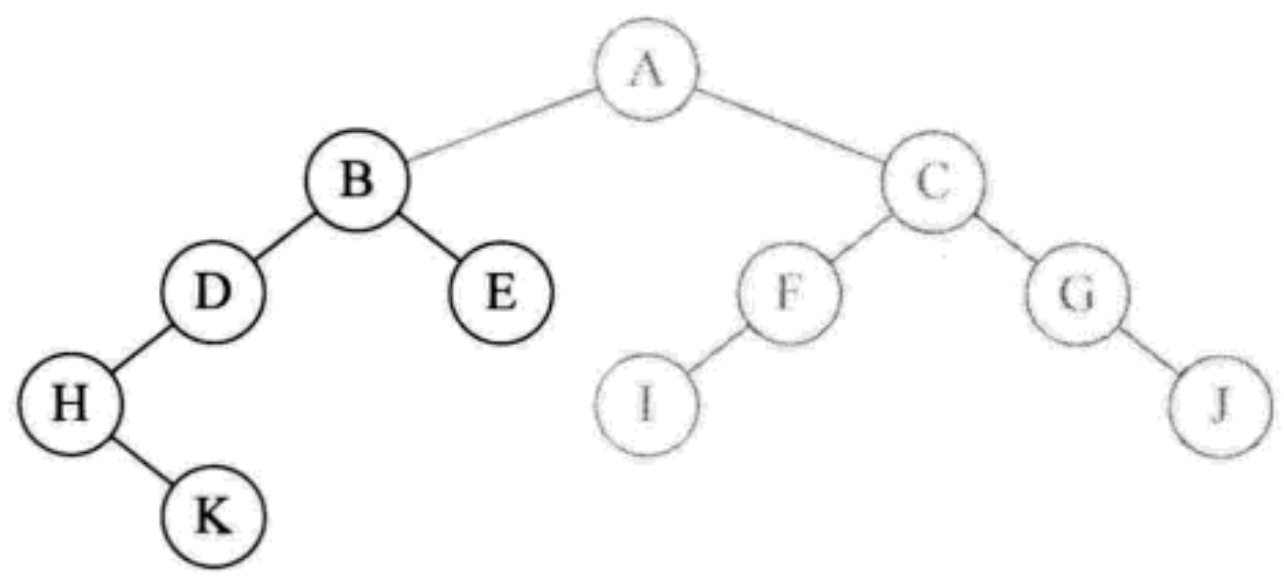6. 节点 E 无右孩子, 返沪. 打印 B 的函数执行完毕, 返回到了我们最初执行 `inOrderTraversal(root)` 的地方, 打印字母 A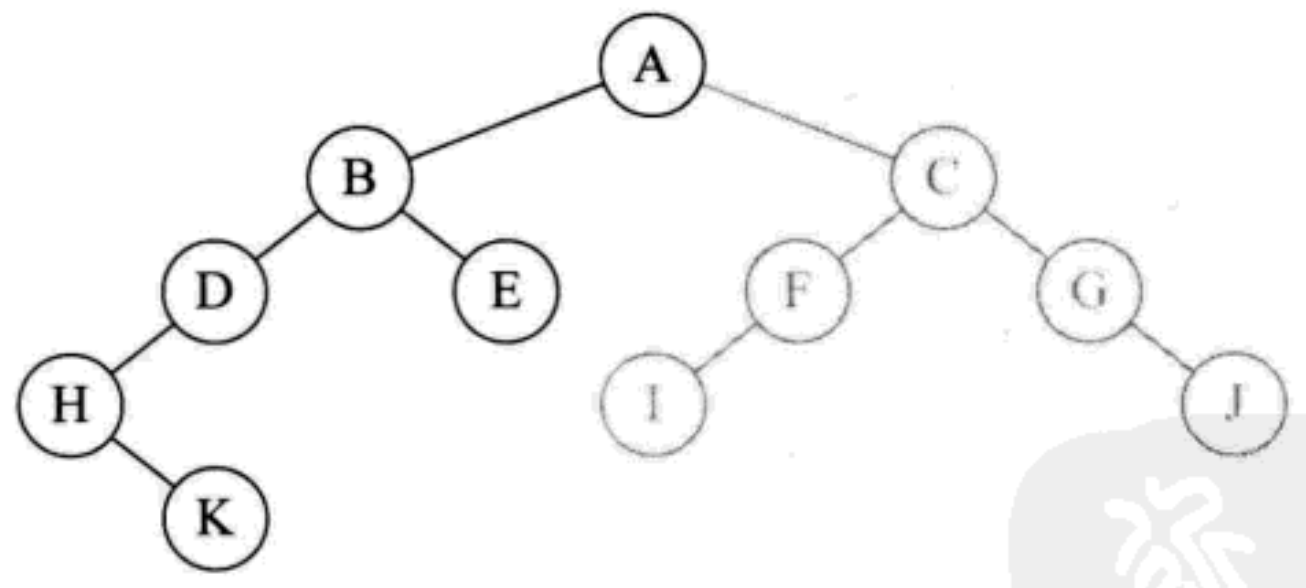7. 调用`inOrderTraversal(root.right)`, 访问 A 节点的右孩子C,再递归访问 C 的左孩子 F, F 的左孩子 I, 因为 I 无右孩子, 所以打印 I. 之后分别打印 F, C, G, J

``````/*
1.先创建一个栈
2.根节点入栈
3.循环取栈顶元素
4.左子树 集体入栈后再入右子树
5.最外层 while 循环判断需要留意.因为 cur= cur.right 会导致 cur == nulll, 因此在加入一个 !stack.empty() 的判断
*/
private static void inOrderTraversalNo(BinaryTreeNode root) {
if (root == null) {
return;
} else {
Stack<BinaryTreeNode> stack = new Stack<>();
BinaryTreeNode cur = root;
while (cur != null || !stack.empty()) {
while (cur != null) {
stack.push(cur);
cur = cur.left;
}
cur = stack.pop();
System.out.print(cur.val + " ");
cur = cur.right;
}
}
}

H K D B E A I F C G J
``````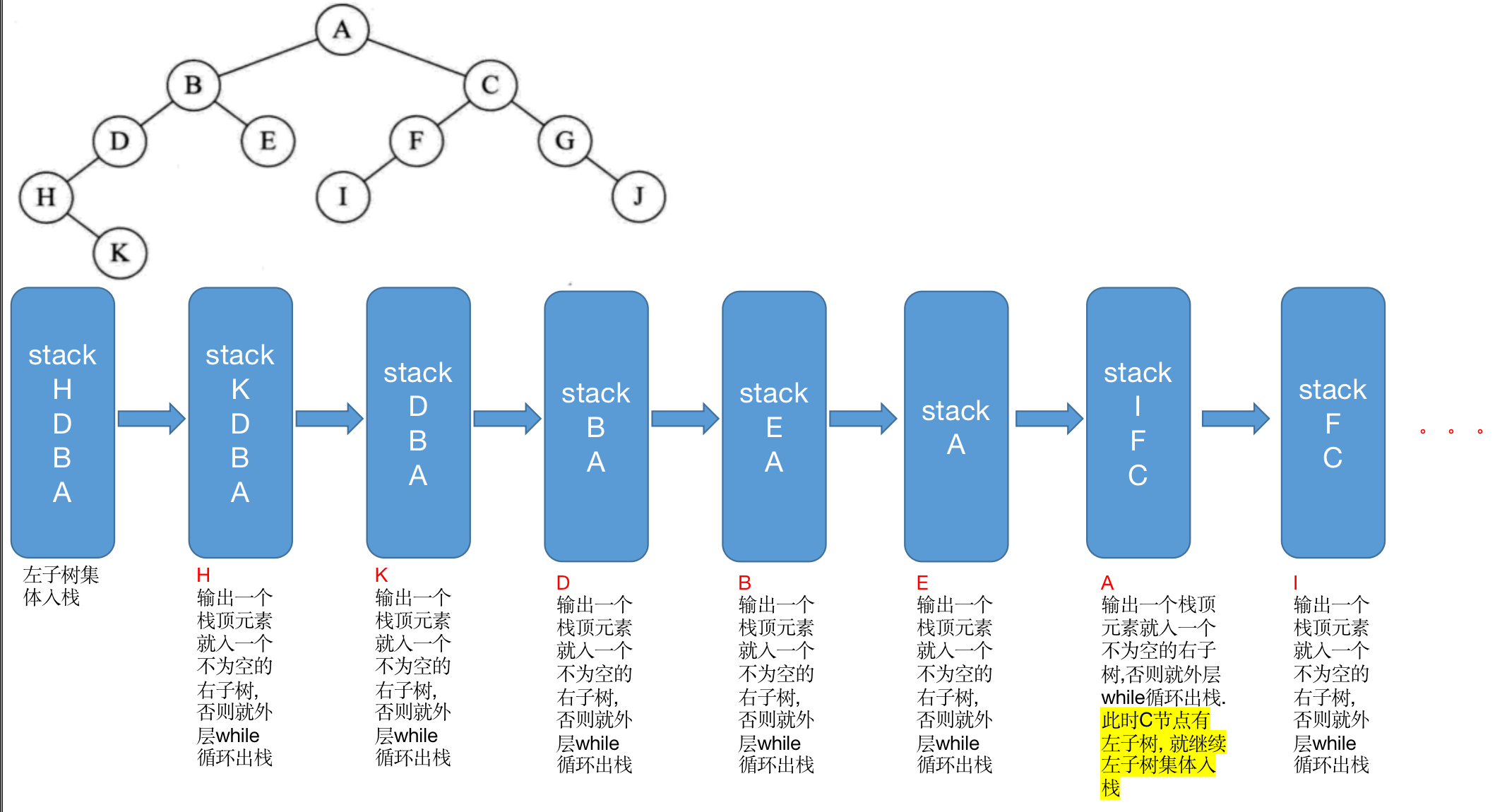#### 3.1.3.2 后序遍历算法左右根]

OJ题目链接

``````/*
OJ: 通过
*/
class Solution {
List<Integer> list = new ArrayList<>();
public List<Integer> postorderTraversal(TreeNode root) {
if(root == null){
return list;
}else{
postorderTraversal(root.left);
postorderTraversal(root.right);
return list;
}
}
}
``````
``````private static void postOrderTraversal(BinaryTreeNode root) {
if (root == null) {
return;
} else {
postOrderTraversal(root.left);
postOrderTraversal(root.right);
System.out.print(root.val + " ");
}
}
``````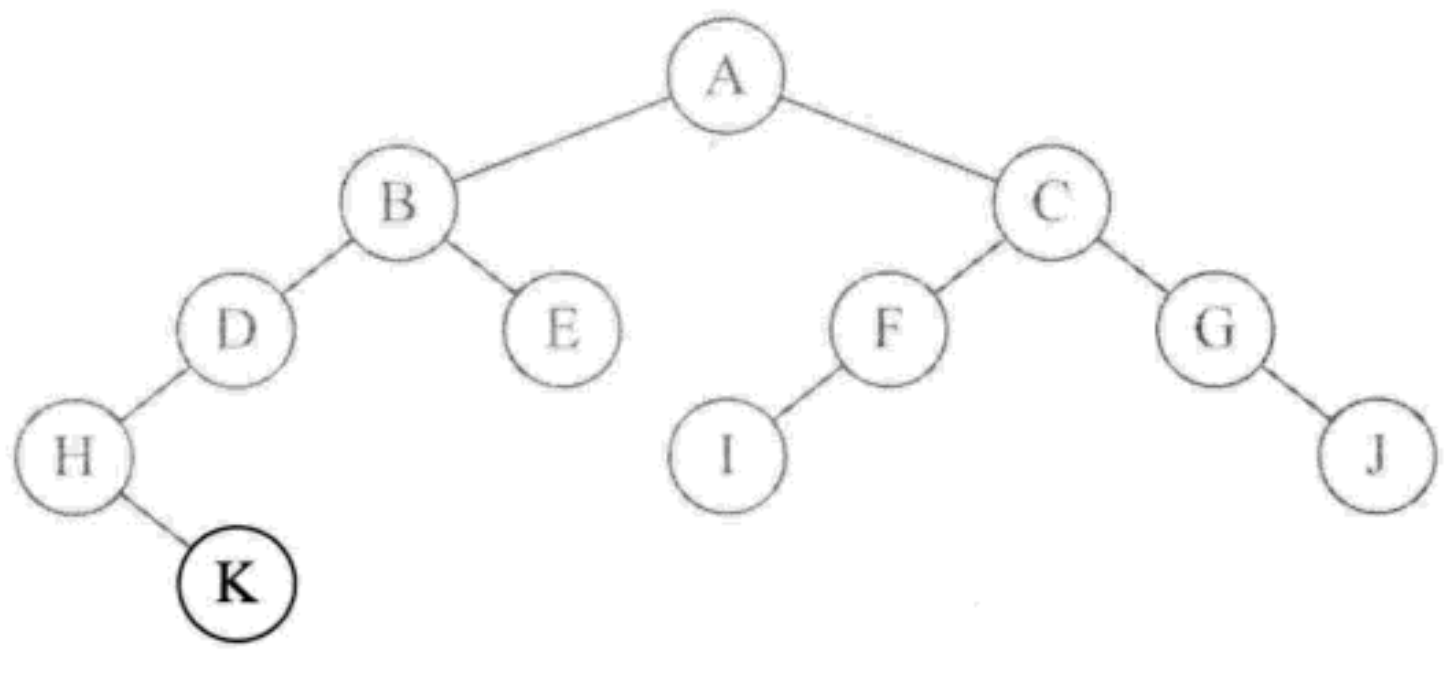1. 后序遍历先递归左子树, 由根节点 A->B->D->H, 节点 H 无左孩子, 再查看 H 的右孩子 K, 因为节点 K 无左右孩子, 所以打印 K.
2. K打印执行完之后返回到执行打印 H 的程序 `System.out.print(root.val + " ");`, 打印 H 节点
3. 后续的分析步骤和都是看返回的节点有无左右子树是否遍历完了: 遍历完了就输出根节点, 否则就继续遍历

``````/*

1.外层循环依旧需要加上 !stack.empty()来带动循环
2.被打印完的节点置为 null, 并且判断该节点是否被打印过
3.判断

*/
private static void postOrderTraversalNo(BinaryTreeNode root) {
if (root == null) {
return;
} else {
Stack<BinaryTreeNode> stack = new Stack<>();
BinaryTreeNode cur = root;
BinaryTreeNode prev = null;
while (cur != null || !stack.empty()) {
while (cur != null) {
stack.push(cur);
cur = cur.left;
}
cur = stack.peek();
if (cur.right == null || cur.right == prev) {
stack.pop();
System.out.print(cur.val + " ");
prev = cur;
cur = null;
} else {
cur = cur.right;
}
}
}
}

K H D E B I F J G C A
``````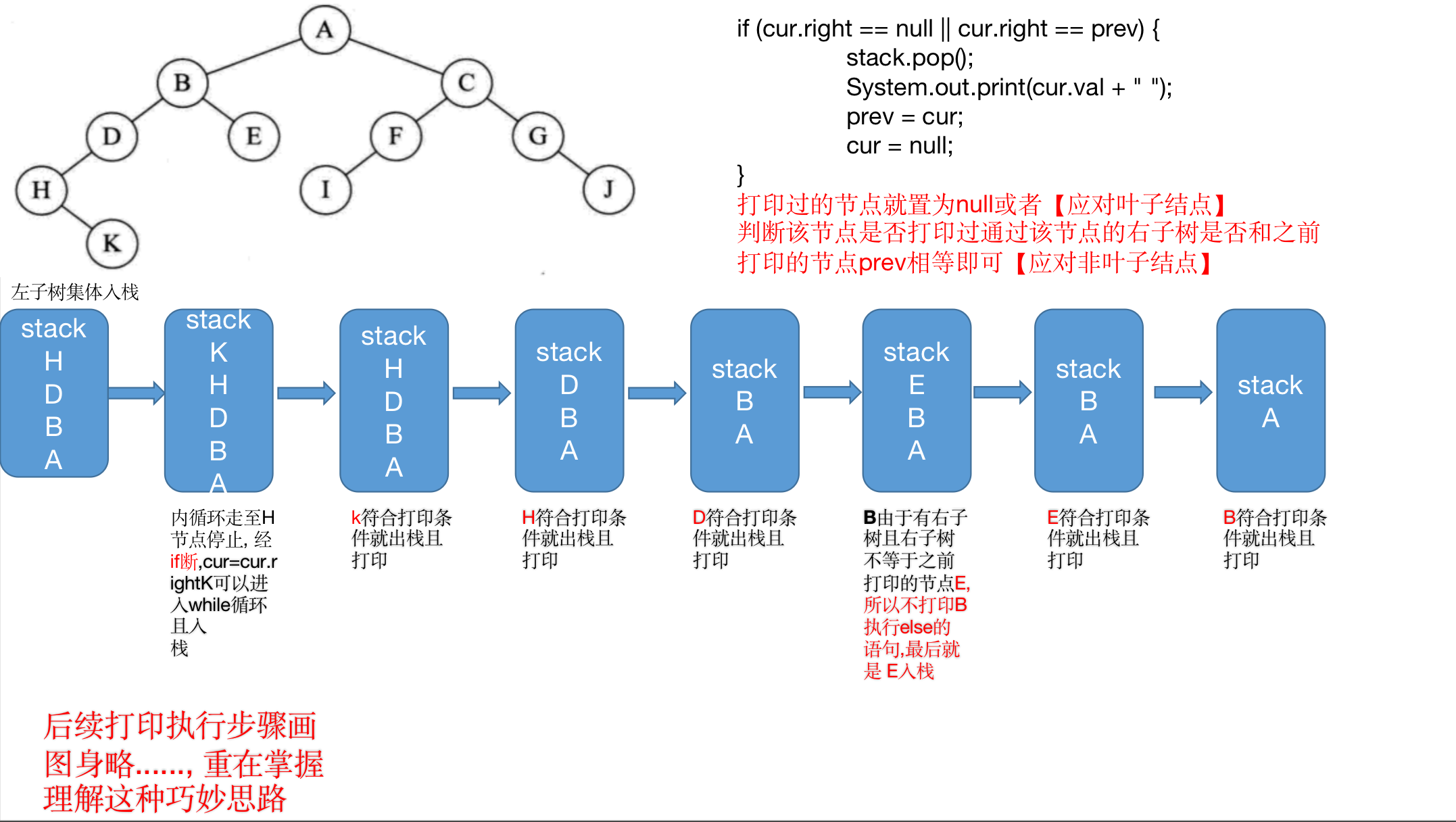#### 3.1.3.2 层序遍历算法上至下, 左至右

OJ题目链接

``````class Solution {
public List<List<Integer>> levelOrder(TreeNode root) {
// 1.返回空盒子
List<List<Integer>> list = new ArrayList<>();
if(root == null){
return list;
}else{
// 2.队列村粗
queue.offer(root);
while(!queue.isEmpty()){
int size = queue.size();
List<Integer> tmp = new ArrayList<>();
while(size-- > 0){
TreeNode top = queue.poll();
if(top.left != null){
queue.offer(top.left);
}
if(top.right != null){
queue.offer(top.right);
}
}
}
return list;
}
}
}
[[A], [B, C], [D, E, F, G], [H, I, J], [K]]
``````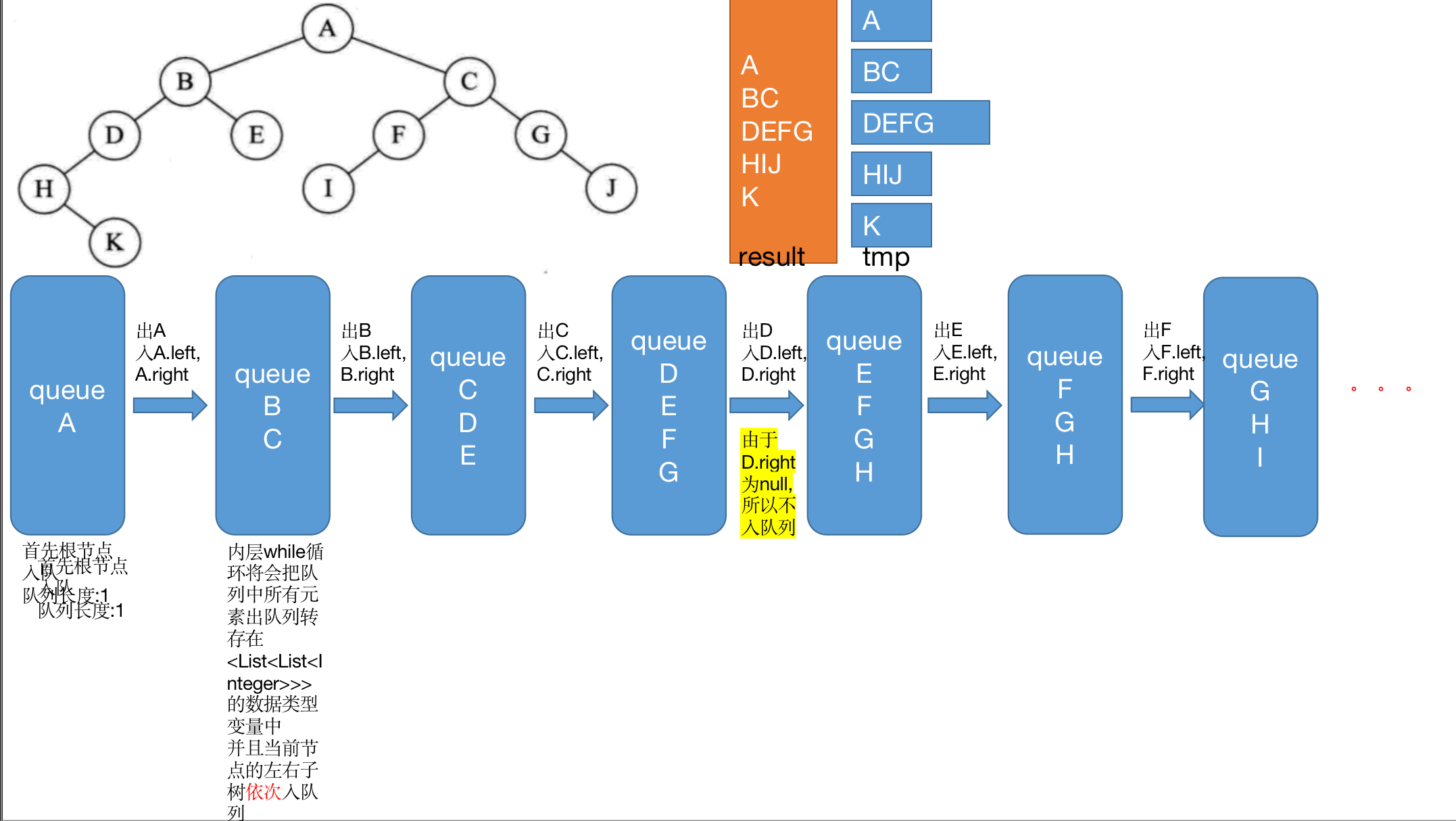``````/*
1.先创建一个队列
2.把根节点放在队列
3.循环取队列元素全部
4.内部 list 添加 当前元素
4.再把当前元素的左子树入队列， 再入右子树
5.大的 ret 添加每次新添元素后的 list
*/
private static void levelOrder(BinaryTreeNode root) {
if (root == null) {
return;
} else {
queue.offer(root);
while (!queue.isEmpty()) {
BinaryTreeNode top = queue.poll();
System.out.print(top.val + " ");
if (top.left != null) {
queue.offer(top.left);
}
if (top.right != null) {
queue.offer(top.right);
}
}
}
}
A B C D E F G H I J K
``````

## 3.2 二叉树建立问题

### 3.2.1 前序中序构建二叉树

OJ题目链接

``````class Solution {
private int findInorderIndex(int[] inorder, int inbegin, int inend, int key){
for(int i=inbegin; i<=inend; ++i){
if(inorder[i] == key){
return i;
}
}
return -1;
}

private TreeNode buildTreeChild(int[] preorder, int[] inorder, int inbegin, int inend){
if(inbegin > inend){
return null;
}else{
TreeNode root = new TreeNode(preorder[preIndex++]);
int rootIndex = findInorderIndex(inorder, inbegin, inend, root.val);
root.left = buildTreeChild(preorder, inorder, inbegin, rootIndex-1);
root.right = buildTreeChild(preorder, inorder, rootIndex+1, inend);
return root;
}
}

private int preIndex = 0;

public TreeNode buildTree(int[] preorder, int[] inorder) {
if(preorder == null || inorder == null){
return null;
}else{
return buildTreeChild(preorder, inorder, 0, inorder.length-1);
}
}
}
``````

1. 判断前序或中序是否有一个为 null【特殊情况处理】
2. 利用 `buildTreeChild` 函数返回根节点
3. 先构造根节点, 再递归构建左子树和右子树
4. 所有递归完之后返回最后的 root 就是树的根节点

1. 第一查找的是 `preorder[preIndex]` 的根节点【preIndex值为0】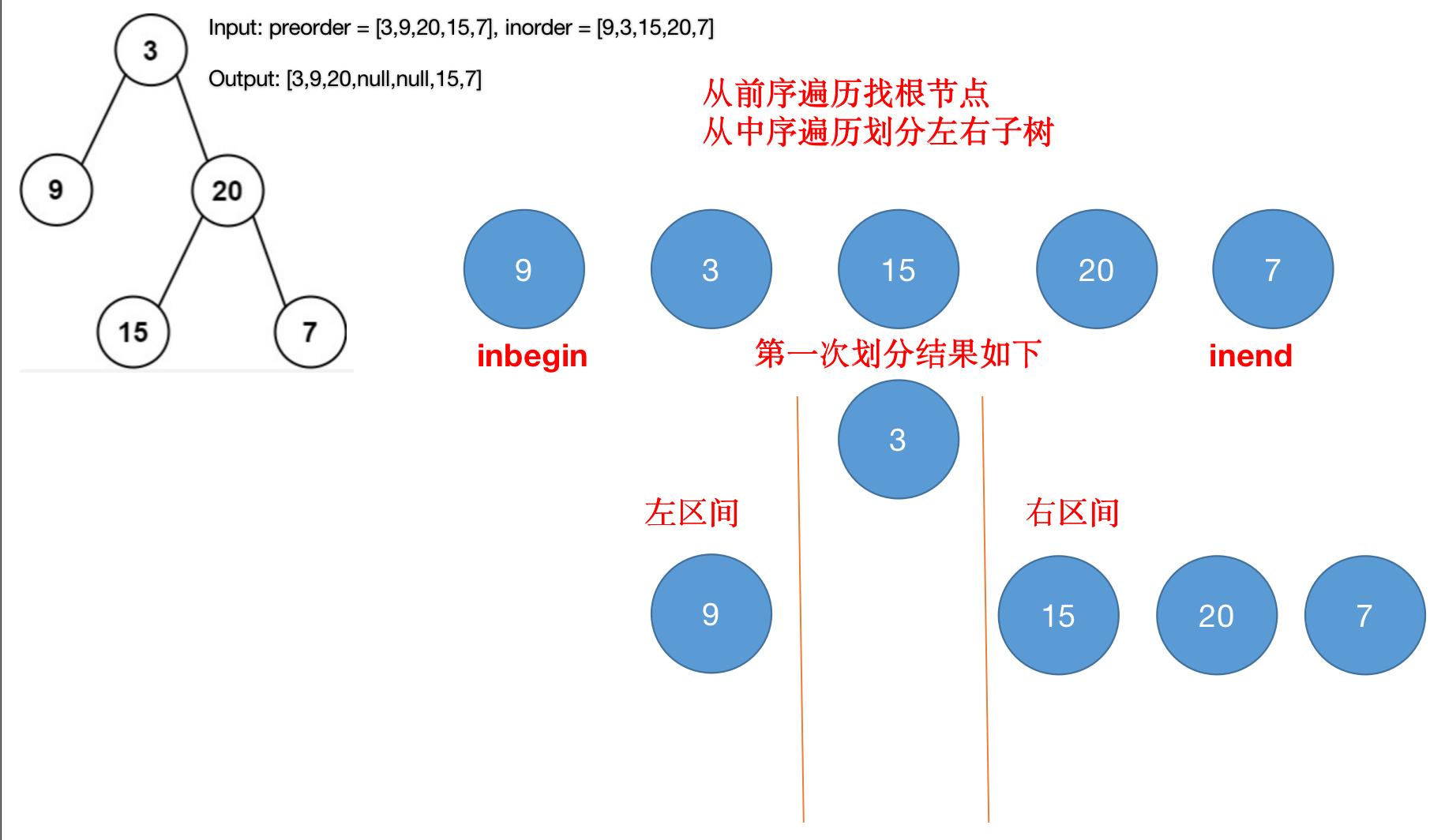2. 由于第二次划分的时候, 新的 inendrootIndex-1 所以导致的 `if(inbegin>inend) return;` 程序返回执行 `root.right = buildTreeChild(preorder, inorder, rootIndex+1, inend);`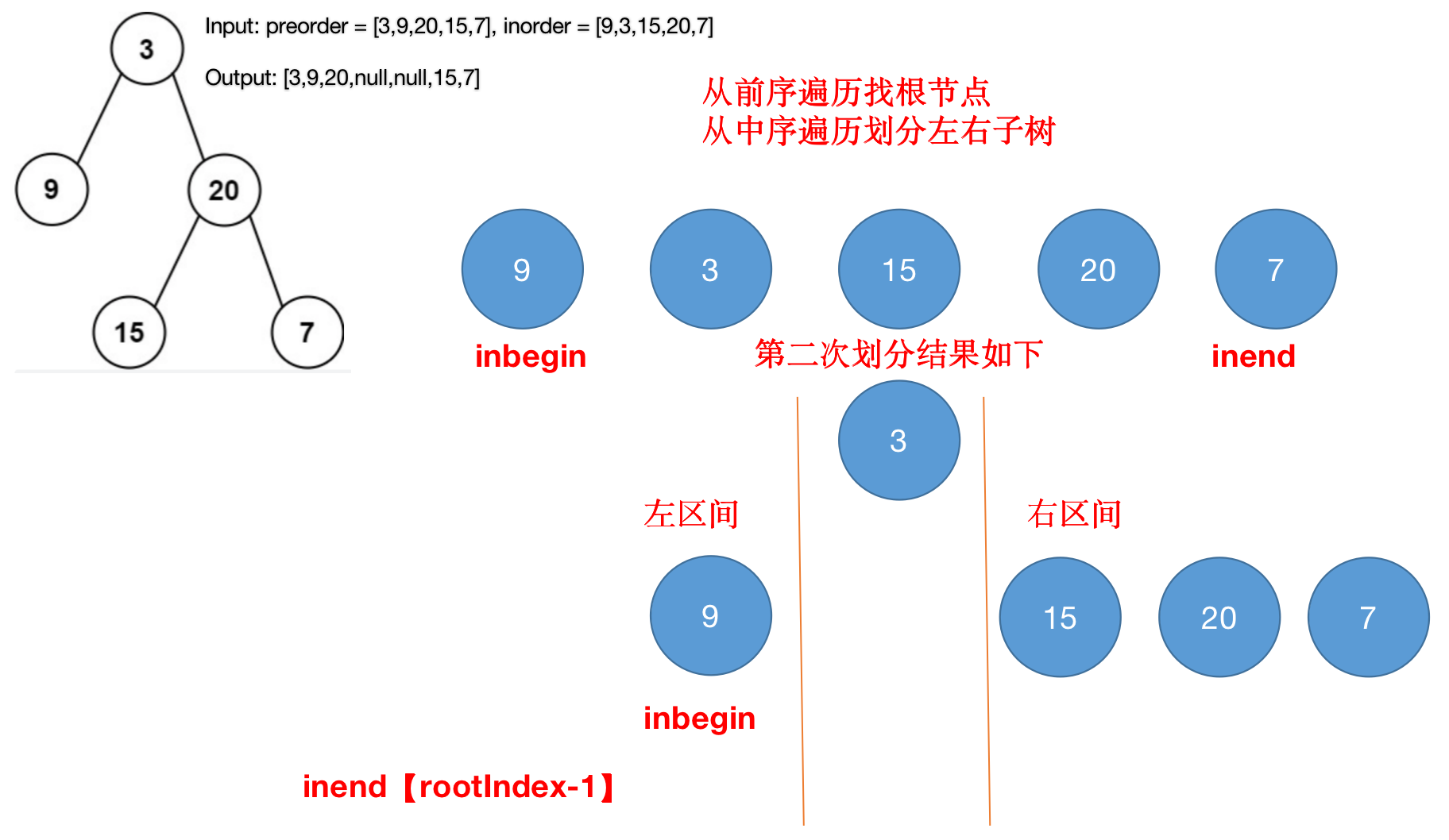3. 第三次划分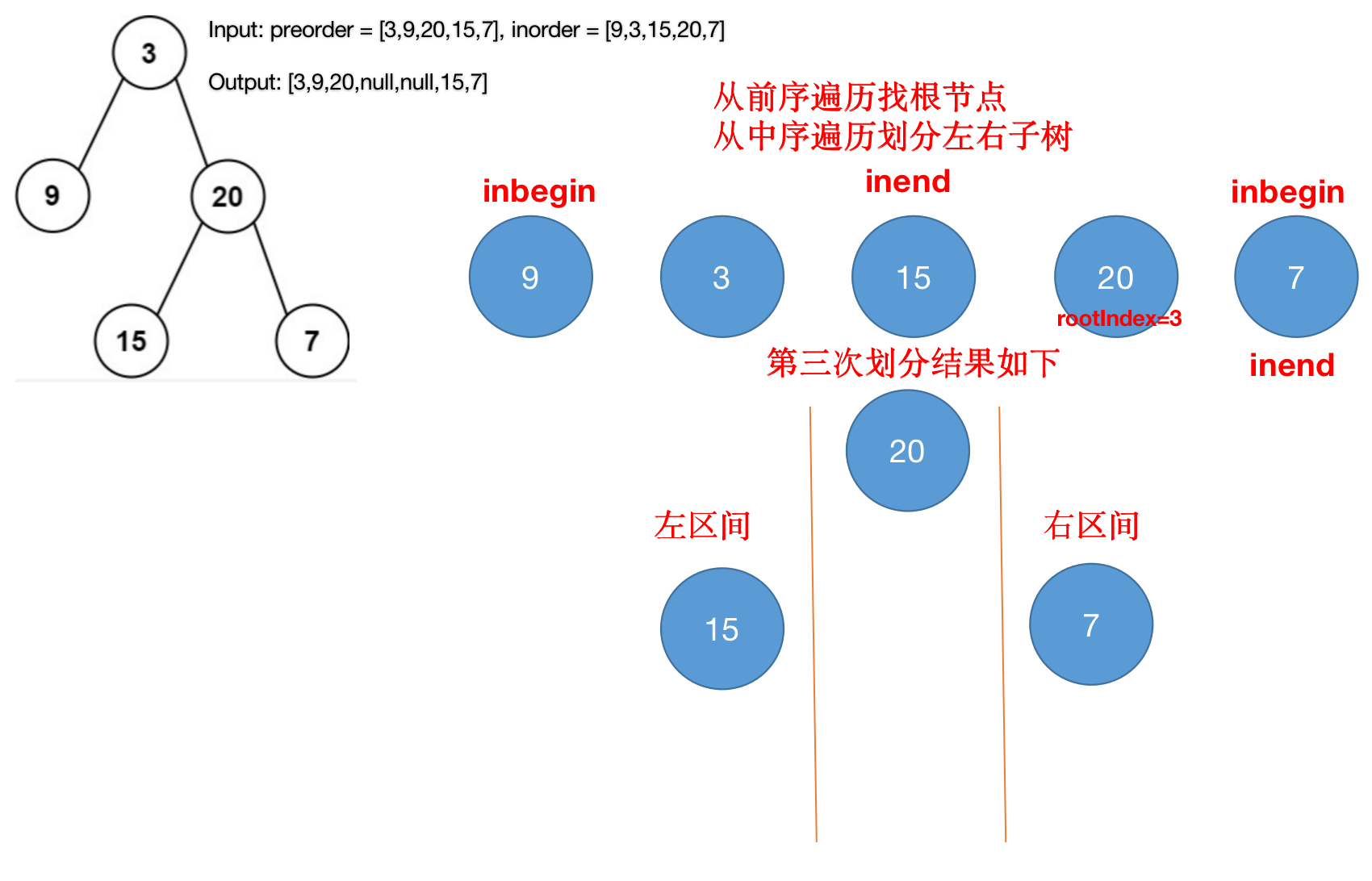4. 综合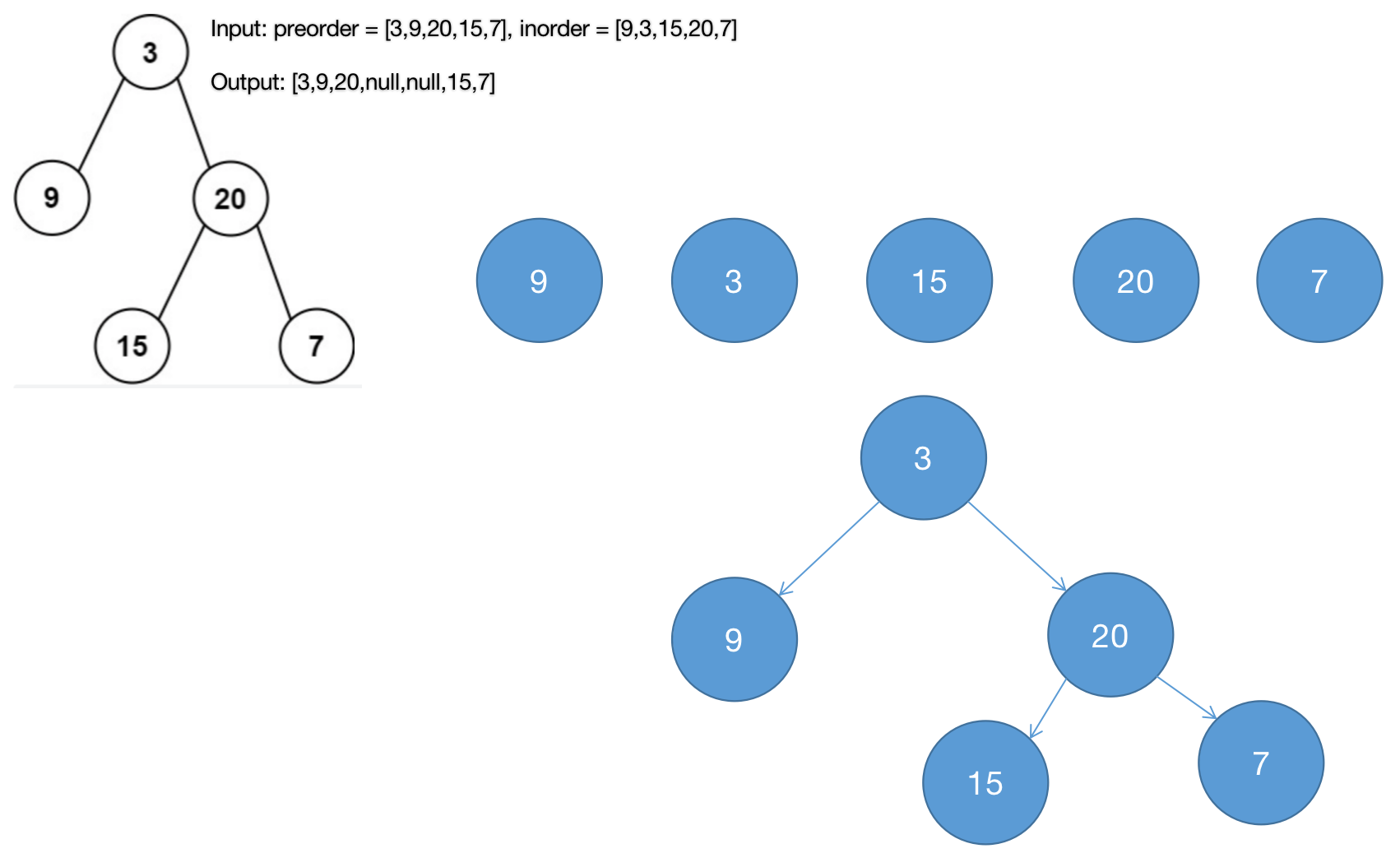### 3.2.2 中序后序构建二叉树

OJ题目链接

``````class Solution {
private int postIndex = 0;

private int findInorderIndex(int[] inorder, int inbegin, int inend, int key){
for(int i=inbegin; i<=inend; ++i){
if(inorder[i] == key){
return i;
}
}
return -1;
}

private TreeNode buildTreeChild(int[] inorder, int[] postorder, int inbegin, int inend){
if(inbegin > inend){
return null;
}else{
TreeNode root = new TreeNode(postorder[postIndex--]);
int rootIndex = findInorderIndex(inorder, inbegin, inend, root.val);
root.right = buildTreeChild(inorder, postorder, rootIndex+1, inend);
root.left = buildTreeChild(inorder, postorder, inbegin, rootIndex-1);
return root;
}
}

public TreeNode buildTree(int[] inorder, int[] postorder) {
if(inorder == null || postorder == null){
return null;
}else{
postIndex = postorder.length-1;
return buildTreeChild(inorder, postorder, 0, postIndex);
}
}
}
``````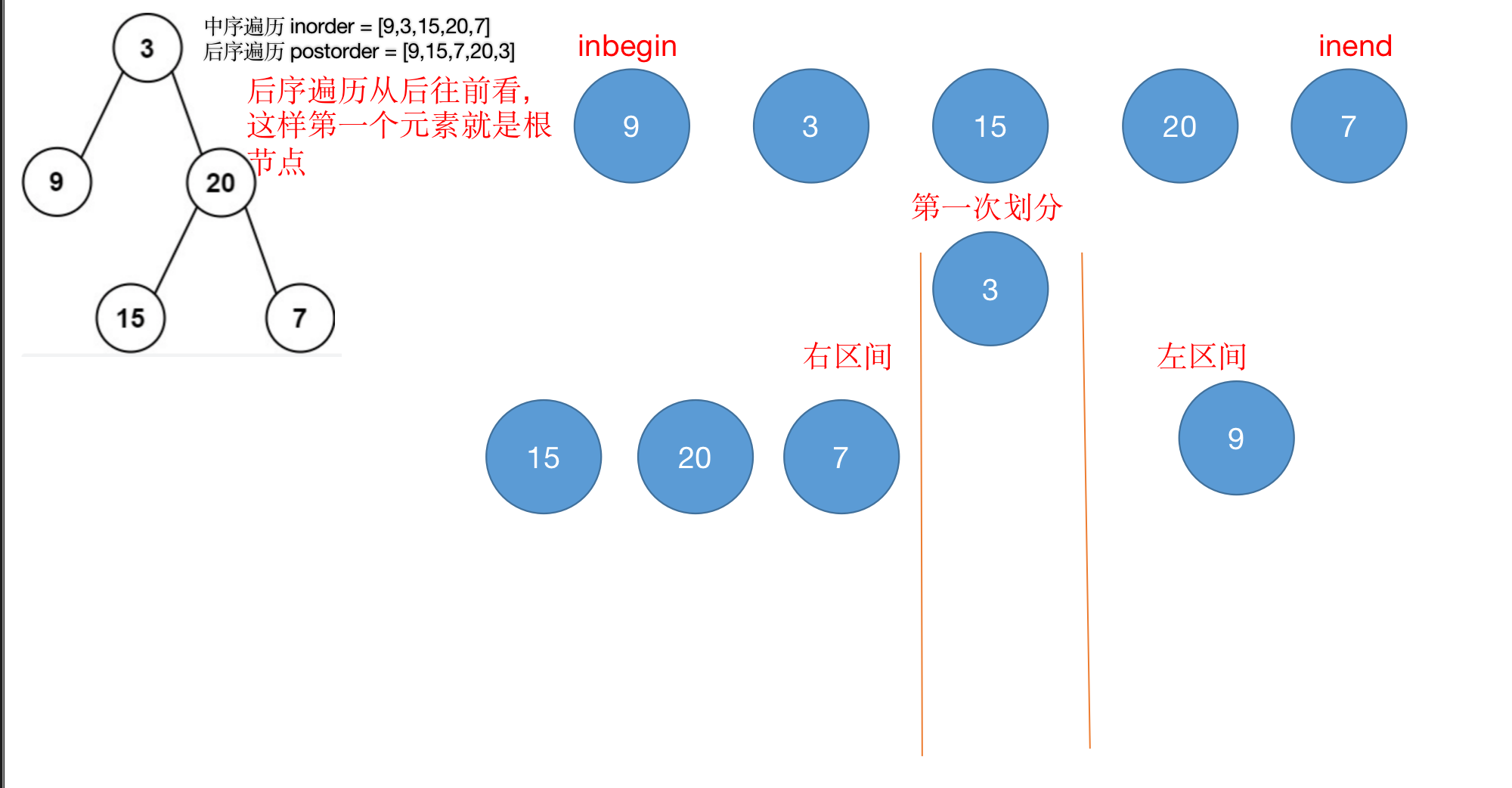1. 已知前序遍历和中序遍历, 可以确定唯一的一颗二叉树
2. 已知中序遍历和后序遍历, 可以确定唯一的一颗二叉树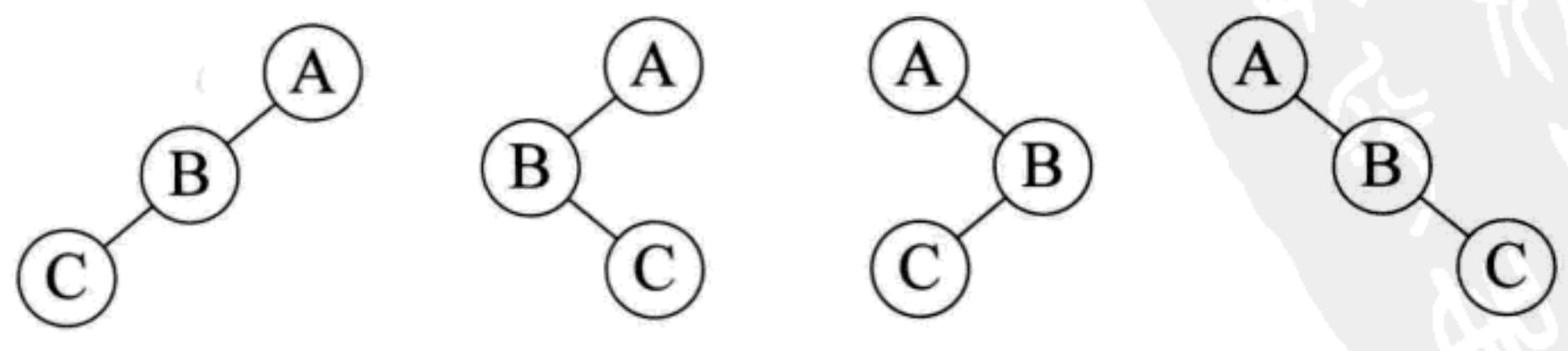### 3.2.3 根据二叉树创建字符串

OJ题目链接

1. 先处理左子树, 再处理右子树
2. 按照题目要求进行一个一个添加括号
``````class Solution {
private void tree2strChild(TreeNode root, StringBuffer stringBuffer){
if(root == null){
return;
}else{
stringBuffer.append(root.val);
// 1.处理左子树
if(root.left == null){
if(root.right == null){
return;
}else{
stringBuffer.append("()");
}
}else{
stringBuffer.append("(");
tree2strChild(root.left, stringBuffer);
stringBuffer.append(")");
}
// 2.处理右子树
if(root.right == null){
return;
}else{
stringBuffer.append("(");
tree2strChild(root.right, stringBuffer);
stringBuffer.append(")");
}
}
}

public String tree2str(TreeNode root) {
if(root == null){
return "";
}else{
StringBuffer stringBuffer = new StringBuffer();
tree2strChild(root, stringBuffer);
return  stringBuffer.toString();
}
}
}
``````

1. 递归前序遍历的形式添加括号
2. 添加完毕之后再删除第一个和最后一个括号即可
``````class Solution {
private StringBuffer stringBuffer = null;
private void tree2strChild(TreeNode root){
if(root == null){
return;
}else{
stringBuffer.append("("+root.val);
tree2strChild(root.left);
if(root.left == null && root.right != null){
stringBuffer.append("()");
}
tree2strChild(root.right);
stringBuffer.append(")");
}
}
public String tree2str(TreeNode root) {
if(root == null){
return "";
}else{
stringBuffer = new StringBuffer();
tree2strChild(root);
stringBuffer.deleteCharAt(0);
stringBuffer.deleteCharAt(stringBuffer.length()-1);
return stringBuffer.toString();
}
}
}
``````

### 3.2.4 前序遍历字符串构建二叉树

OJ题目链接

``````import java.util.Scanner;
import java.util.Stack;

class TreeNode{
char val;
TreeNode left;
TreeNode right;
TreeNode(char val){
this.val = val;
}
}

public class Main{

// 1.采用的是迭代的方式进行中序遍历
private static void inOrderTraversal(TreeNode root){
if(root == null){
return;
}else{
Stack<TreeNode> stack = new Stack<>();
TreeNode cur = root;
while(cur != null || !stack.empty()){
// 2. 左子树集体入栈
while(cur != null){
stack.push(cur);
cur = cur.left;
}
cur = stack.pop();
System.out.print(cur.val + " ");
cur = cur.right;
}
}
}

private static int index = 0;// 记录打印的字符串下标, 因为是递归, 所以应该使用全局变量而不是局部变量
private static  TreeNode createTree(String str){
if(str == null){
return null;
}else{
TreeNode root = null;
// 1.越过空节点
if(str.charAt(index) == '#'){
++index;
}else{
// 2. 按照前序根左右的形式递归构建二叉树
root = new TreeNode(str.charAt(index++));
root.left = createTree(str);
root.right = createTree(str);
}
return root;
}
}

public static void main(String[] args){
Scanner scanner = new Scanner(System.in);
while(scanner.hasNext()){
String str = scanner.next();
TreeNode root = createTree(str);
inOrderTraversal(root);
System.out.println();
}
}
}
``````

### 3.2.5 合并二叉树

OJ题目练习

``````class Solution {
public TreeNode mergeTrees(TreeNode root1, TreeNode root2) {
// 1.如果某个节点为null, 则返回另一个节点
if(root1 == null){
return root2;
}else if(root2 == null){
return root1;
}else{
// 2.如果两个节点都不为null, 则开始合并二叉树
TreeNode root = new TreeNode(root1.val+root2.val);
root.left = mergeTrees(root1.left, root2.left);
root.right = mergeTrees(root1.right, root2.right);
return root;
}
}
}
``````

### 3.2.6 递增二叉树

OJ练习题目

``````class Solution {
private TreeNode cur = null;

private void inOrderTraversal(TreeNode root){
if(root == null){
return;
}else{
//1.左
inOrderTraversal(root.left);
// 2.根
cur.right = root;
root.left =
// 3.右
inOrderTraversal(root.right);
}
}

public TreeNode increasingBST(TreeNode root) {
if(root == null){
return null;
}else{
// 1.傀儡节点用来保存cur节点的位置用于返回
TreeNode newRoot = new TreeNode(-1);
// 2.移动节点
cur = newRoot;
inOrderTraversal(root);
return newRoot.right;
}
}
}
``````

## 3.3 经典题目解析

### 3.3.1 结点个数

``````/*

*/
private static int size = 0;

private static void getSize1(BinaryTreeNode root) {
if (root == null) {
return;
} else {
++size;
getSize1(root.left);
getSize1(root.right);
}
}

private static int getSize2(BinaryTreeNode root) {
if (root == null) {
return 0;
} else {
return 1 + getSize2(root.left) + getSize2(root.right);
}
}
``````

### 3.3.2 叶子结点个数

``````/*

*/
private static int leaftSize = 0;

private static void getLeafSize1(BinaryTreeNode root) {
if (root == null) {
return;
} else {
if (root.left == null && root.right == null) {
++leaftSize;
} else {
getLeafSize1(root.left);
getLeafSize1(root.right);
}
}
}

private static int getLeafSize2(BinaryTreeNode root) {
if (root == null) {
return 0;
} else {
if (root.left == null && root.right == null) {
return 1;
} else {
return getLeafSize2(root.left) + getLeafSize2(root.right);
}
}
}
``````

### 3.3.3 第k层结点个数

``````private static int getKLevelSize(BinaryTreeNode root, int k) {
if (root == null || k < 1) {
return 0;
} else {
if (k == 1) {
return 1;
} else {
return getKLevelSize(root.left, k - 1) + getKLevelSize(root.right, k - 1);
}
}
}
``````

### 3.3.4 查找 val 所在结点

``````private static BinaryTreeNode find(BinaryTreeNode root, String val) {
if (root == null) {
return null;
} else {
if (root.val.equals(val)) {
return root;
} else {
BinaryTreeNode ret = find(root.left, val);
if (ret != null && ret.val.equals(val)) {
return ret;
}
ret = find(root.right, val);
if (ret != null && ret.val.equals(val)) {
return ret;
}
return null;
}
}
}
``````

### 3.3.5 相同的树

OJ题目练习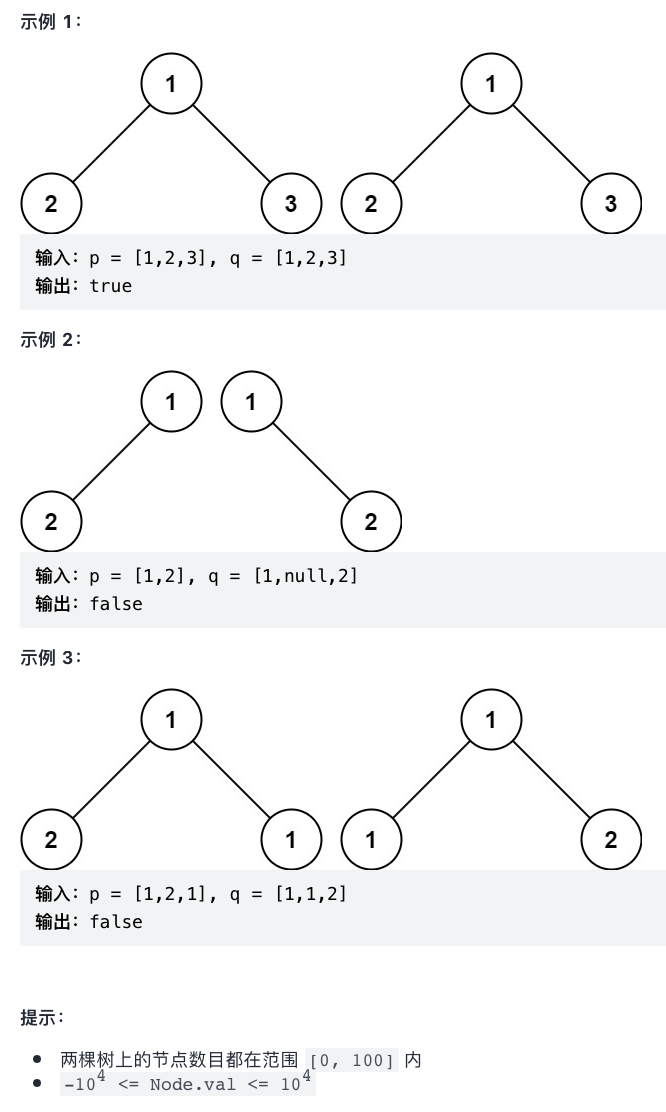``````class Solution {
public boolean isSameTree(TreeNode p, TreeNode q) {
// 1.都为null： 说明之前的节点和节点值相等, 所以相等; 或者两个空节点也是相等的
if(p == null && q == null){
return true;
// 2.某个节点遍历完了但另一个节点不为null, 所以是false
}else if(p == null || q == null){
return false;
}else{
// 3节点值不相等就 false
if(p.val != q.val){
return false;
}else{
// 4. 递归进行下一次比较
return isSameTree(p.left, q.left) && isSameTree(p.right, q.right);
}
}

}
``````

### 3.3.6 另一棵树子树

OJ题目练习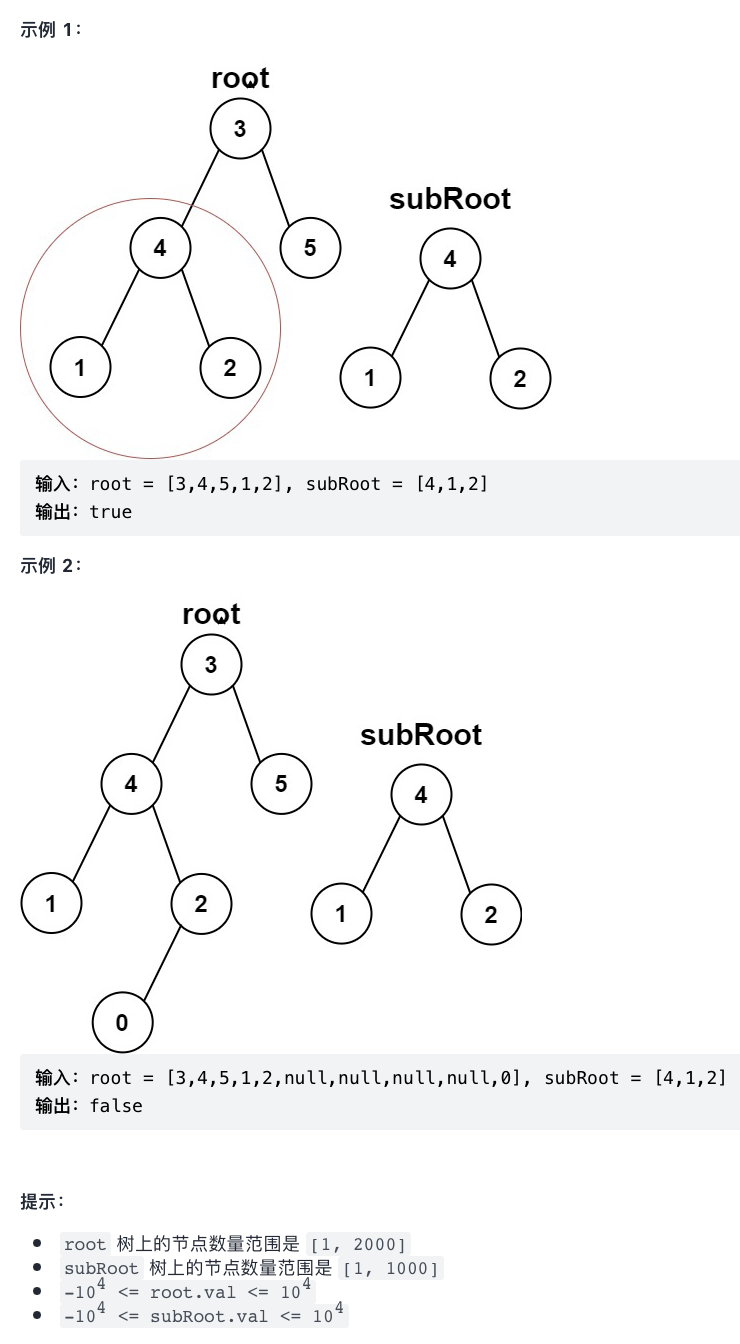``````class Solution {
public boolean isSameTree(TreeNode p, TreeNode q) {
// 1.都为null： 说明之前的节点和节点值相等, 所以相等; 或者两个空节点也是相等的
if(p == null && q == null){
return true;
// 2.某个节点遍历完了但另一个节点不为null, 所以是false
}else if(p == null || q == null){
return false;
}else{
// 3节点值不相等就 false
if(p.val != q.val){
return false;
}else{
// 4. 递归进行下一次比较
return isSameTree(p.left, q.left) && isSameTree(p.right, q.right);
}
}
}

public boolean isSubtree(TreeNode root, TreeNode subRoot) {
// 1.两个 nullnull 则是子树; 遍历到了叶子结点的子树【虽然不存在】, 说明之前遍历的都是相等的
if(root == null && subRoot == null){
return true;
}else if(root == null && subRoot != null || root != null && subRoot == null){
return false;
}else{
// 1.如果两个节点不为空, 且相等, 则是子树
if(isSameTree(root, subRoot)){
return true;
// 2.递归判断是否为子树
}else if(isSubtree(root.left, subRoot)){
return true;
}else if(isSubtree(root.right, subRoot)){
return true;
}else{
return false;
}
}
}
}
``````

### 3.3.7 二叉树最大深度

OJ练习题目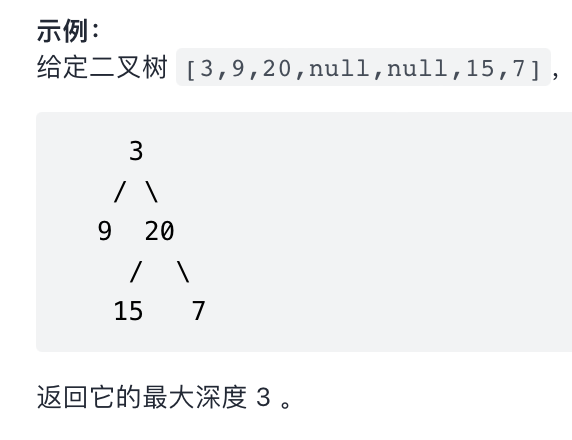``````class Solution {
public int maxDepth(TreeNode root) {
if(root == null){
return 0;
}else{
// 1.计算左子树的高度
int left = maxDepth(root.left)+1;
// 2.计算右子树的高度
int right = maxDepth(root.right)+1;
if(left>right){
return left;
}else{
return right;
}
}
}
}
``````

### 3.3.8 平衡二叉树

OJ练习题目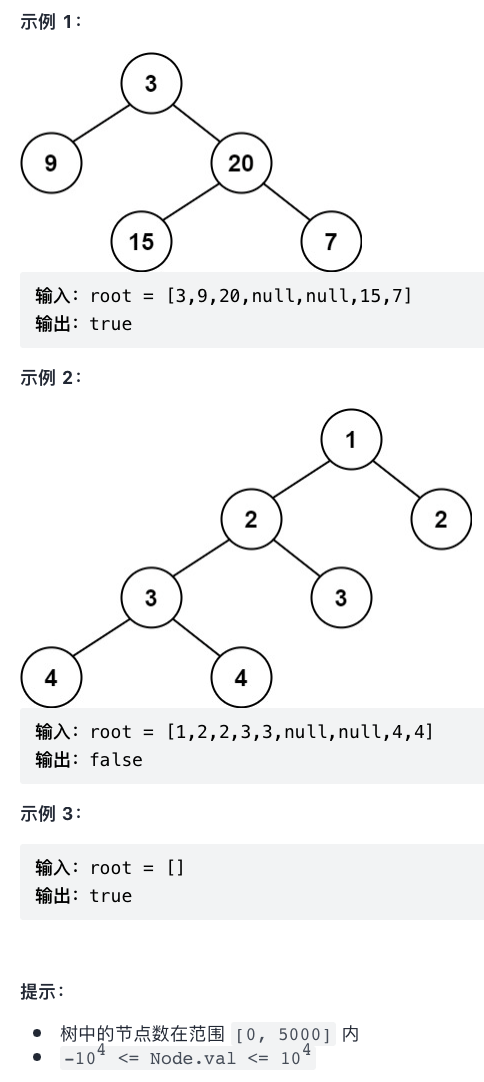``````class Solution {
private int maxDepth(TreeNode root){
if(root == null){
return 0;
}else{
int left = 1+maxDepth(root.left);
int right = 1+maxDepth(root.right);
return left>right?left:right;
}
}

public boolean isBalanced(TreeNode root) {
if(root == null){
return true;
}else{
int left = maxDepth(root.left);
int right = maxDepth(root.right);
if(Math.abs(left-right)>1){
return false;
}else{
return isBalanced(root.left) && isBalanced(root.right);
}
}
}
}
``````
``````// 1. 优化后的代码: 边求高度边计算左右子树高度差, 不用递归两次
class Solution {
private int maxDepth(TreeNode root){
if(root == null){
return 0;
}else{
int left = maxDepth(root.left);
int right = maxDepth(root.right);
if(left >= 0 && right >= 0 && Math.abs(left-right)<=1){
return Math.max(left, right)+1;
}else{
return -1;
}
}
}
public boolean isBalanced(TreeNode root) {
return maxDepth(root) > -1;
}
}
``````

### 3.3.9 对称二叉树

OJ练习题目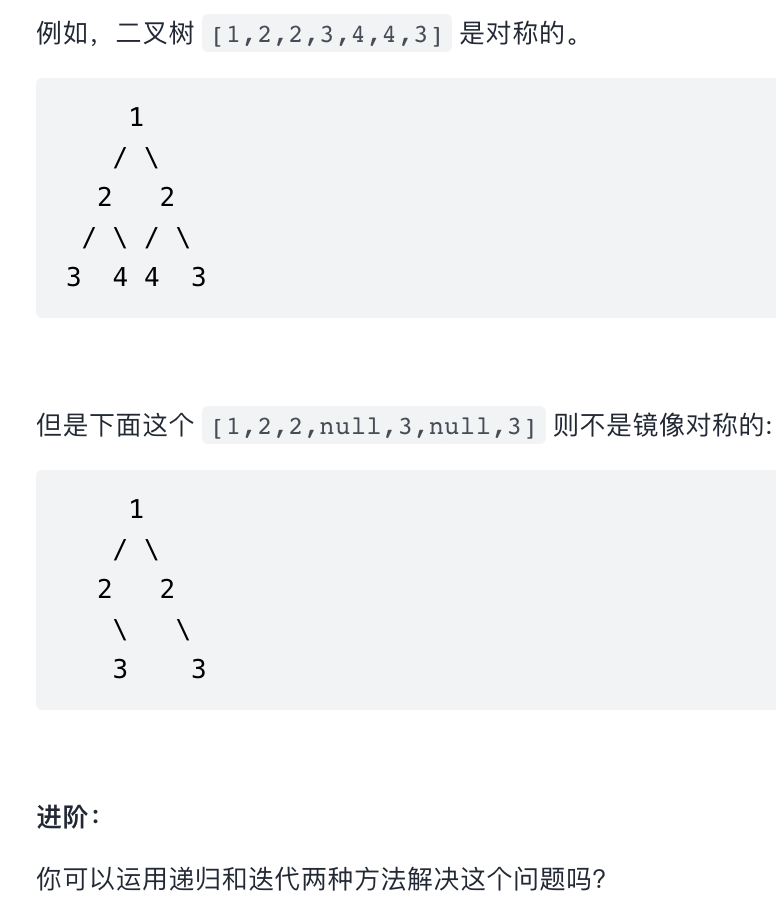``````class Solution {
private boolean isSameTree(TreeNode leftTree, TreeNode rightTree) {
if (leftTree == null && rightTree == null) {
return true;
} else if (leftTree != null && rightTree == null || leftTree == null && rightTree != null) {
return false;
} else {
if (leftTree.val != rightTree.val) {
return false;
} else {
/*
1. 之前的判断步骤和 比较二叉树是否相同 有点类似
2. 之后应该比较 左树的左 和 右树的右; 左树的右 和 右树的左 是否相同
*/
return isSameTree(leftTree.left, rightTree.right) && isSameTree(leftTree.right, rightTree.left);
}
}
}

public boolean isSymmetric(TreeNode root) {
if (root == null) {
return true;
} else {
return isSameTree(root.left, root.right);
}
}
}
``````

### 3.3.10 二叉树的完全性检验

OJ题目练习``````/*
1. 当遇到 null 节点时候说明已经遍历所有完全二叉树就退出
2. 在队列末尾慢慢弹出元素, 如果弹出了不为 null 则说明不是一个完全二叉树
*/
class Solution {
public boolean isCompleteTree(TreeNode root) {
if(root == null){
return true;
}else{
queue.offer(root);
// 1.直到遇到 null 节点就停止入队列
while(!queue.isEmpty()){
TreeNode top = queue.poll();
if(top != null){
queue.offer(top.left);// 程序走到叶子结点, 则会把 null 节点也带入队列, 后续的 peekpeek 探测的时候如果遇到了不为 null 的节点的话就代表在遇到 null 节点之后还有其它叶子结点, 此时一定不是完全二叉树
queue.offer(top.right);
}else{
break;
}
}//程序执行到这儿, 说明队列中已经存储所有的节点
// 2.节点出队列, peek探测元素是否为 null
while(!queue.isEmpty()){
TreeNode top = queue.peek();
if(top != null){
return false;
}else{
queue.poll();//出队列
}
}
return true;
}
}
}
``````

``````class Solution {
private int n=0, p=0;

private boolean dfs(TreeNode root, int k){
if(root == null){
return true;// 1.节点遍历完毕
}else if(k > 100){
return false;// 2.题目提示: 树中将会有 1 到 100 个结点
}else{
++n;// 3.每遍历一次, 结点个数就自增一, 记录当前节点下标
p = Math.max(p, k);// 4.因为有左右子树, 所以每次应该选取最大下标才可
return dfs(root.left, 2*k) && dfs(root.right, 2*k+1);
}
}

public boolean isCompleteTree(TreeNode root) {
if(root == null){
return true;
}else{
if(!dfs(root, 1)){
return false;// 1. 超过 100 节点就返回 false
}else{
return n == p;// 2.未超过 100 节点, 判断节点数和最大下标数是否相等
}
}
}
}
``````

### 3.3.11 二叉树最近公公祖先

OJ题目练习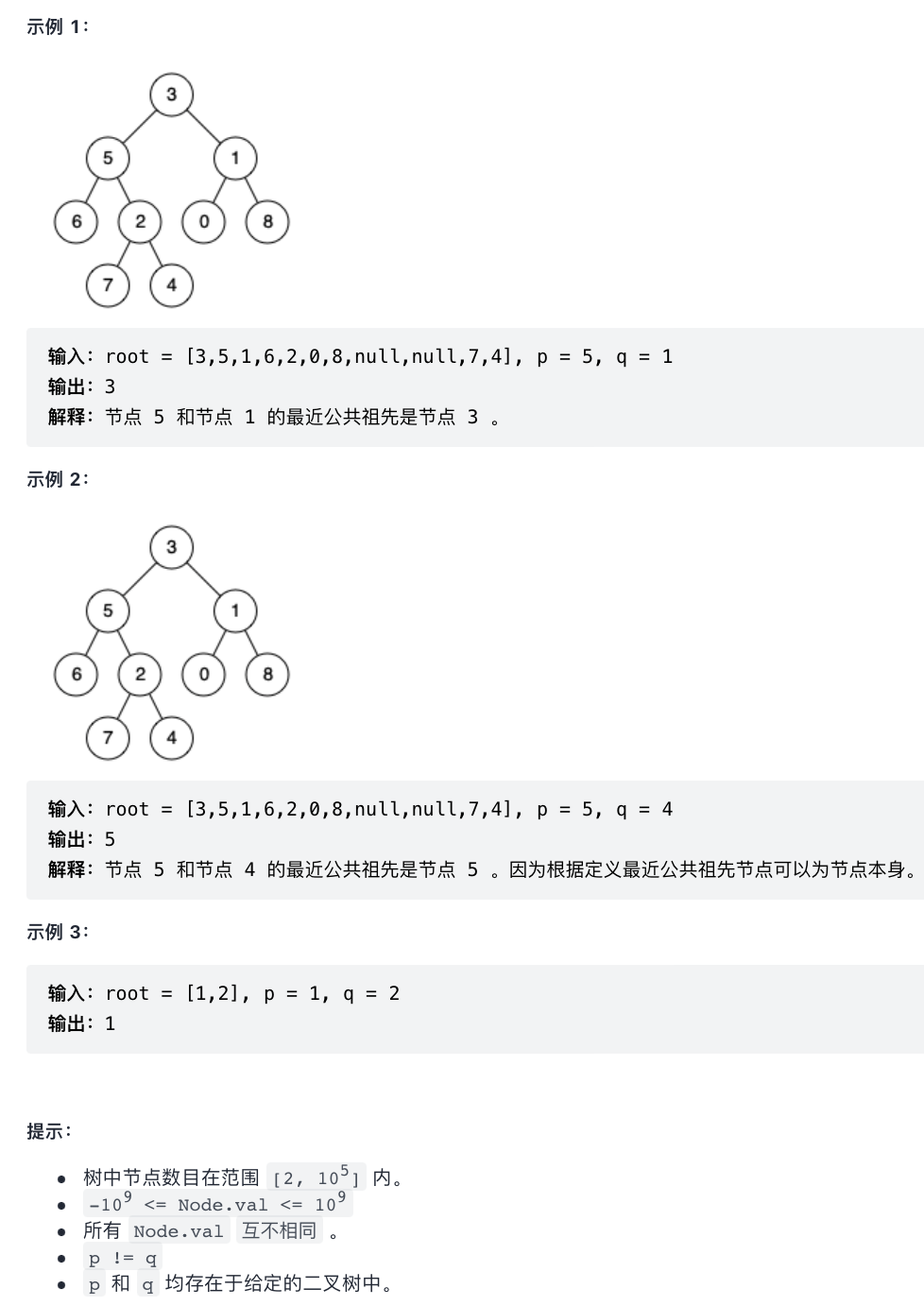``````/*
left == null, right == null: return null
left != null, right != null: return root
left == null, right != null: return right
left != null, right == null: return left
*/
class Solution {
public TreeNode lowestCommonAncestor(TreeNode root, TreeNode p, TreeNode q) {
if(root == null || root == p || root == q){
return root;
}else{
TreeNode left = lowestCommonAncestor(root.left, p, q);
TreeNode right = lowestCommonAncestor(root.right, p, q);
if(left == null && right == null){
return null;
}else if(left != null && right == null){
return left;
}else if(left == null && right != null){
return right;
}else{
return root;
}
}
}
}
``````

### 3.3.12 二叉树最大宽度

OJ题目练习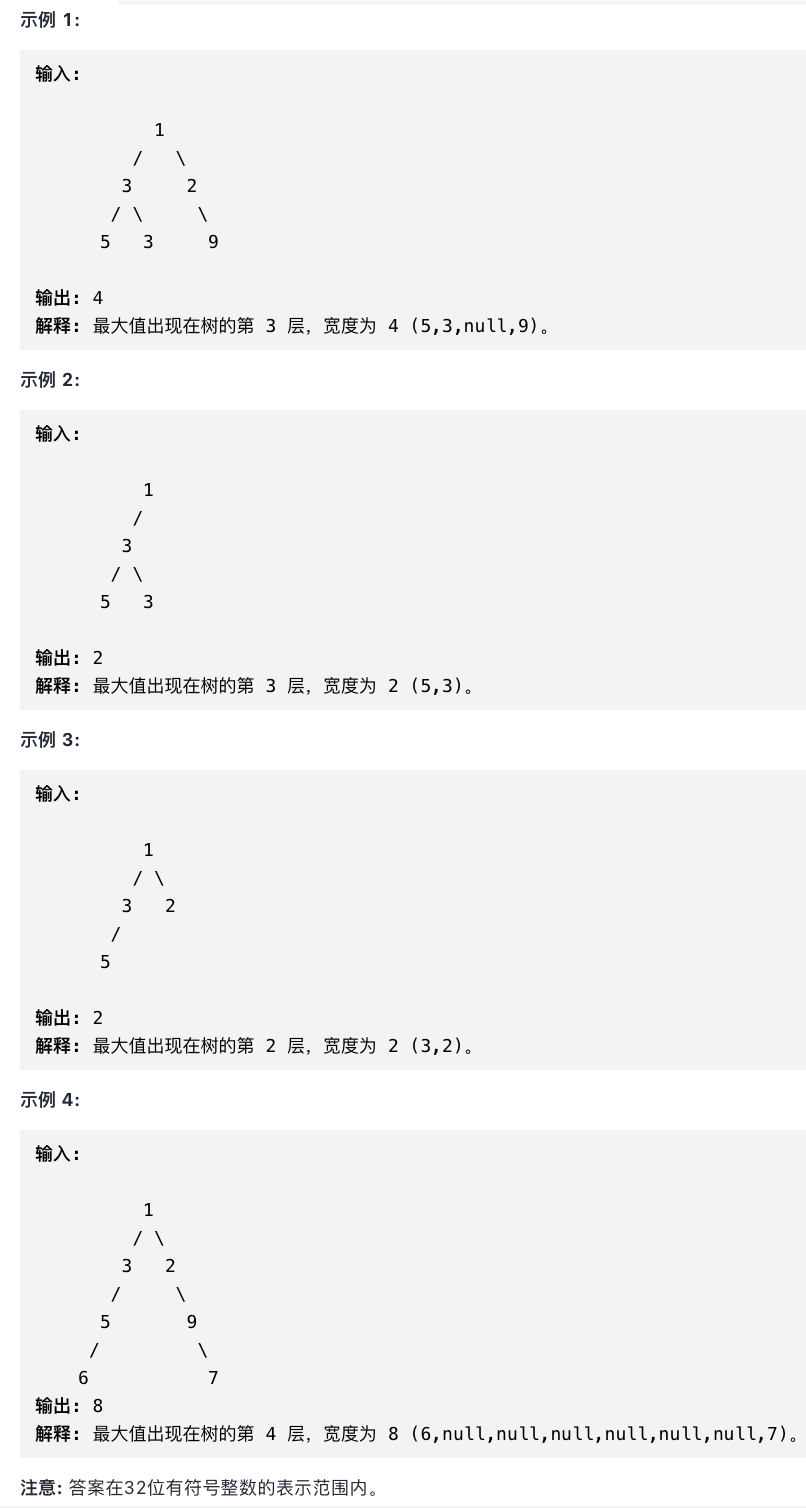``````class Solution {
public int widthOfBinaryTree(TreeNode root) {
if(root == null){
return 0;
}else{
queue.offer(root);
int max_width = 0;
while(!queue.isEmpty()){
int cur_width = queue.getLast().val-queue.getFirst().val+1;// 1.利用结点的值域记录当前队列宽度
// 2.头节点的左右子树入队列, 为下一次宽度计算做准备
int count = queue.size();// 3.队列大小时刻变化着的, 我们应该保留入队列之前的队列大小
for(int i=0; i<count; ++i){
TreeNode top = queue.poll();// 4.出队列元素且保留, 为后续左右子树入队列做铺垫
if(top.left != null){
queue.offer(top.left);
top.left.val = top.val << 1;// 5.依据的是二叉树的性质5--左孩子:2*i    根:i    右孩子: 2*i+1
}
if(top.right != null){
queue.offer(top.right);
top.right.val = (top.val << 1) + 1;
}
}
if(max_width < cur_width){
max_width = cur_width;
}
}
return max_width;
}
}
}
``````

## 3.4. 特殊的二叉树问题

### 3.4.1 搜索二叉树

``````    static class BinaryTreeNode {
// 键值对的形式存储
int key;
int value;
BinaryTreeNode left;
BinaryTreeNode right;

public BinaryTreeNode(int key, int value) {
this.key = key;
this.value = value;
}
}

// root: 表示树的根节点, 空树就是用 null 来表示
private BinaryTreeNode root = null;

//查找: 根据 Key 找 Value
private Integer search(int key) {
BinaryTreeNode cur = root;
while (cur != null) {
if (key < cur.key) {
cur = cur.left;
} else if (key > cur.key) {
cur = cur.right;
} else {
return key;
}
}
return null;
}

// 插入
private void insert(int key, int value) {
if (root == null) {
root = new BinaryTreeNode(key, value);
} else {
BinaryTreeNode cur = root;
BinaryTreeNode parend = null;
while (cur != null) {
parend = cur;
if (key < cur.key) {
cur = cur.left;
} else if (key > cur.key) {
cur = cur.right;
} else {
return;// 二叉搜索树不存在相等, 如果相等也没必要赋值
}
}
// 程序执行到这儿, 说明 parent 已经是 cur 的父节点, 修改父节点即可
if (key < parend.key) {
parend.left = new BinaryTreeNode(key, value);
} else {
parend.right = new BinaryTreeNode(key, value);
}
}
}

// 删除: 按照 key 删除
private void remove(int key) {
// 1.先查找 key 对应的位置
BinaryTreeNode cur = root;
BinaryTreeNode parent = null;
while (cur != null) {
parent = cur;
if (key < cur.key) {
cur = cur.left;
} else if (key > cur.key) {
cur = cur.right;
} else {
/*
2.核心删除
考虑当前 cur.left 为空
是否为根节点 root
是: 链接右子树
否: 接着判断当前删除的子节点 cur 是其父节点 parent 节点的左右子树关系
考虑当前 cur.right 为空
紧接 cur.left 根节点判断一样的思路
考虑当前 cur.right 和 cur.left 都不为空
移形换影删除
*/
if (cur.left == null) {
if (cur == root) {
root = cur.right;
} else if (cur == parent.left) {
parent.left = cur.right;
} else if (cur == parent.right) {
parent.right = cur.right;
}
} else if (cur.right == null) {
if (cur == root) {
root = cur.left;
} else if (cur == parent.left) {
parent.left = cur.left;
} else if (cur == parent.right) {
parent.right = cur.left;
}
} else {
// 具体移形换影步骤
// 1.右子树找最小值, 并记录其父节点
BinaryTreeNode targetParent = cur;
BinaryTreeNode target = cur.right;
while (target.left != null) {// 最小值特点是: 左子树为空
targetParent = target;
target = target.left;
}
// 2.程序执行到这儿: targetParent 是 target 父节点; target 是右子树中最小值
// 3.就把 替罪羊target 节点的值赋值给删除节点
cur.key = target.key;
cur.value = target.value;
// 4.断开末尾 最小值节点 的关系, 建立新的关系
if (target == targetParent.left) {
targetParent.left = target.right;// 最小值左节点为 null
} else if (target == targetParent.right) {
targetParent.right = target.right;
}
}
}
}
}
``````

### 3.4.2 赫夫曼树及其应用

#### 3.4.1. 赫夫曼树``````if (a < 60) {
b = "不及格";
} else if (a < 70) {
b = "及格";
} else if (a < 80) {
b = "中等";
} else if (a < 90) {
b = "良好";
} else {
b = "优秀";
}
``````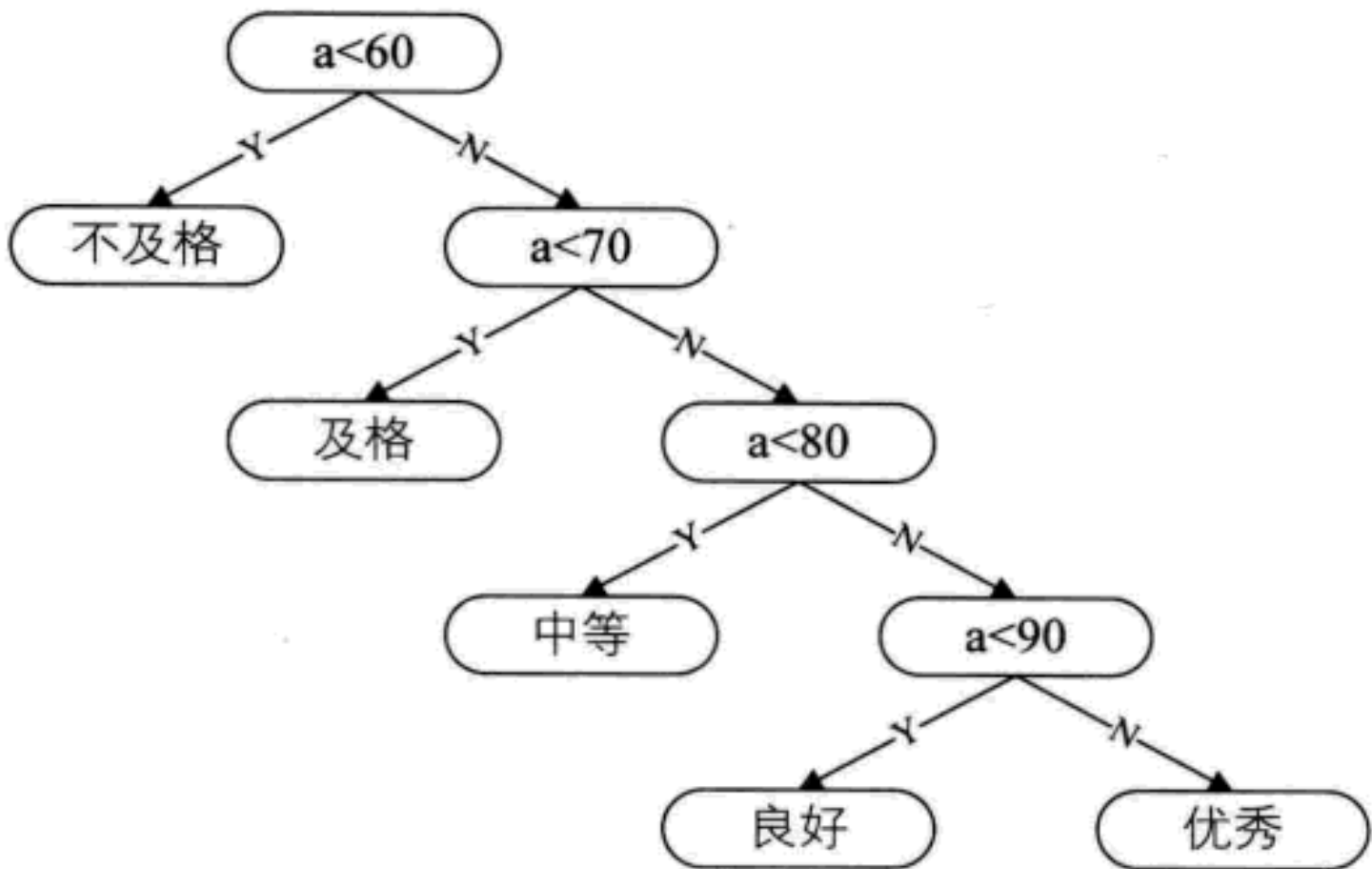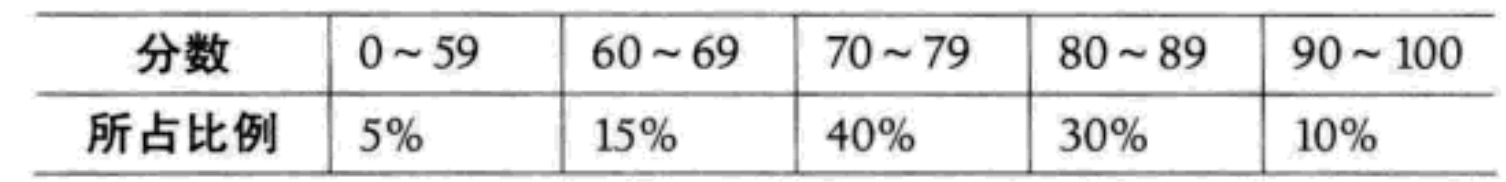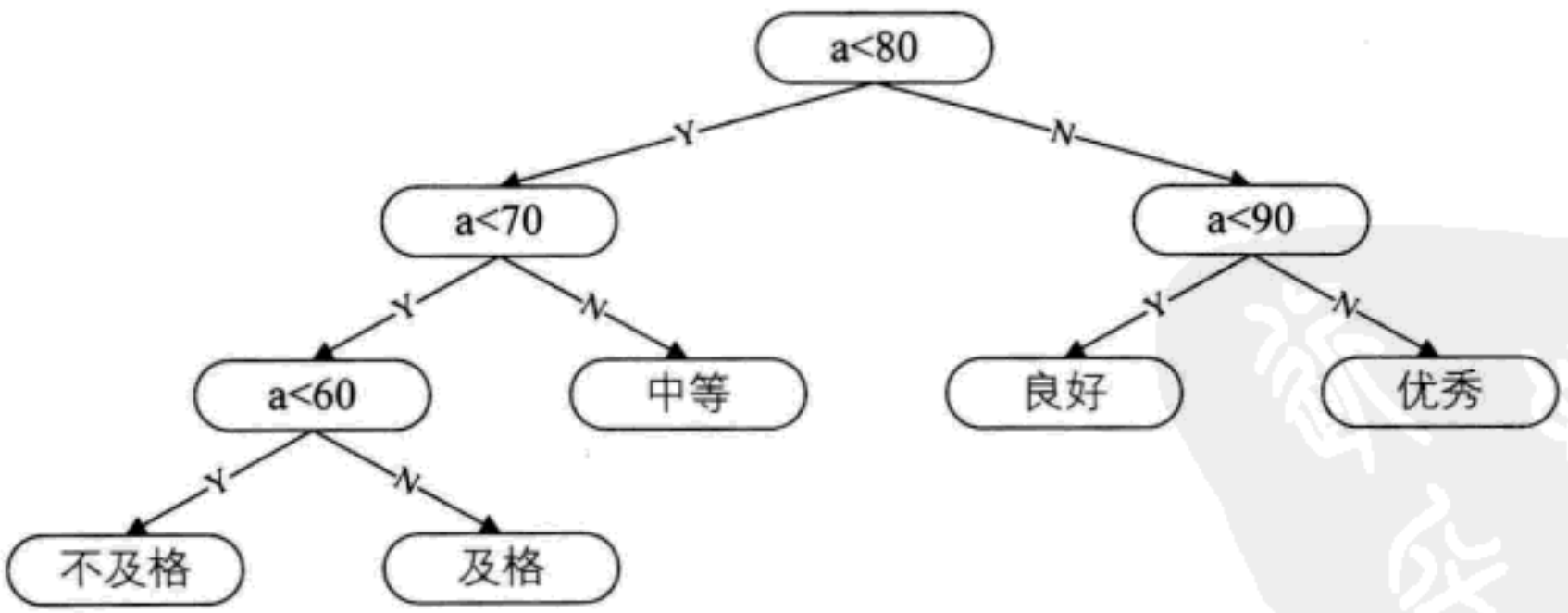#### 3.4.2.赫夫曼树定义与原理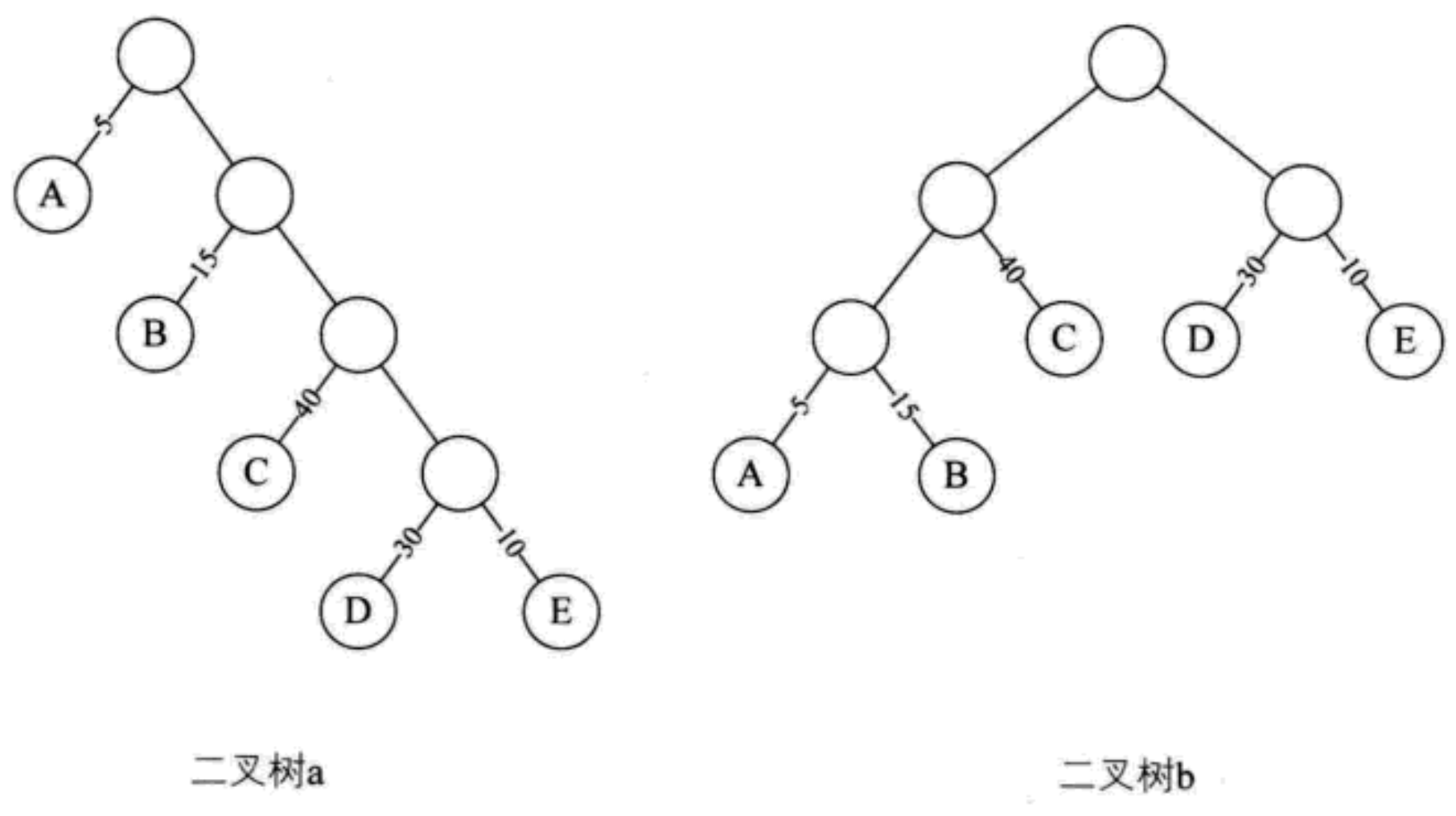1. 先把有权值的叶子结点按照从小到大的顺序排列成一个有序序列[A5, E10, B15, D30, C40]
2. 取头两个最小全值的节点作为一个新节点 N1 的两个字节点, A 是 N1 左子树, E 是 N1 右子树. 注意左右子树大小关系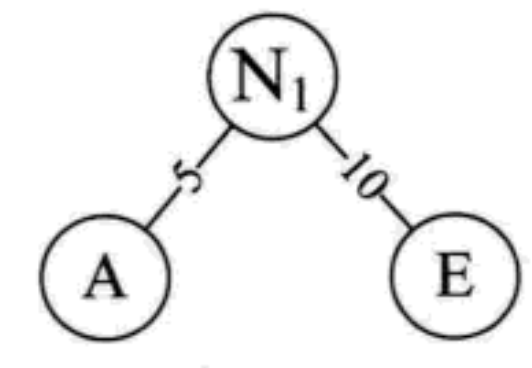3. 将 N1 替换 A 与 E, 插入有序序列中, 保持从小到大排列[N1 15, B15, D30, C40]
4. 重复步骤2. 将 N1 与 B 作为一个新节点 N2 的两个字节点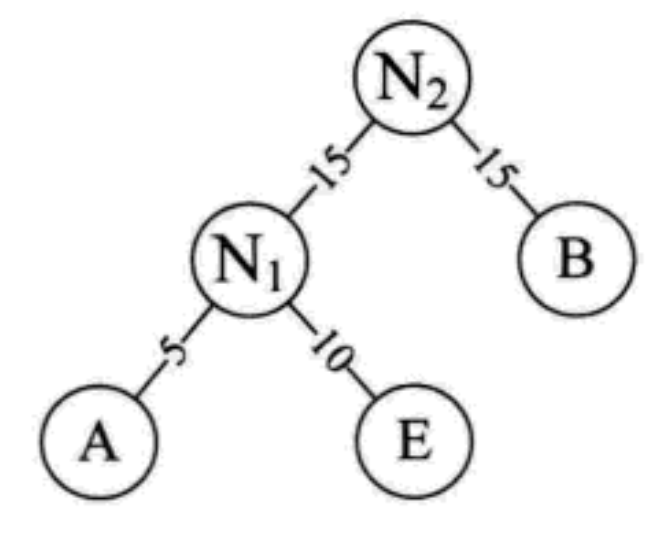重复步骤3的序列替换序列最小值, 再重复步骤2生成新的节点…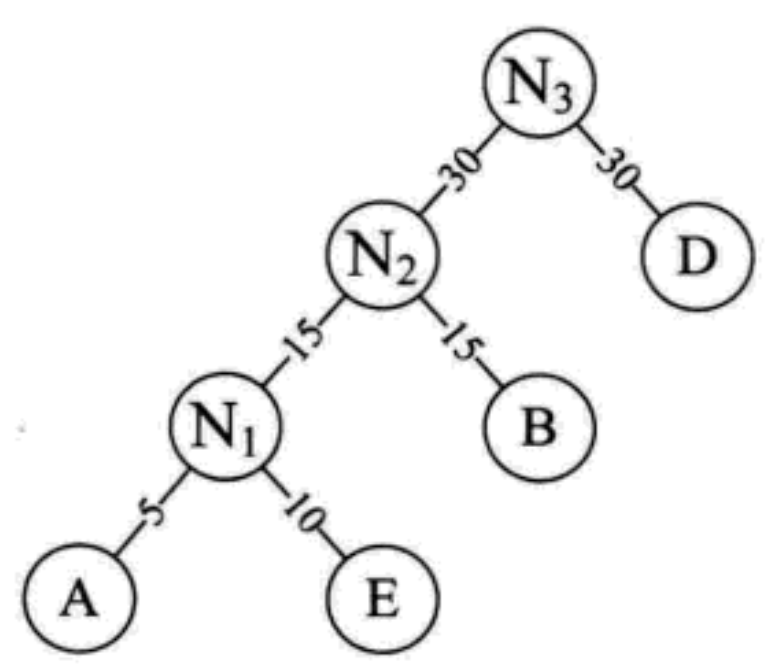最终效果：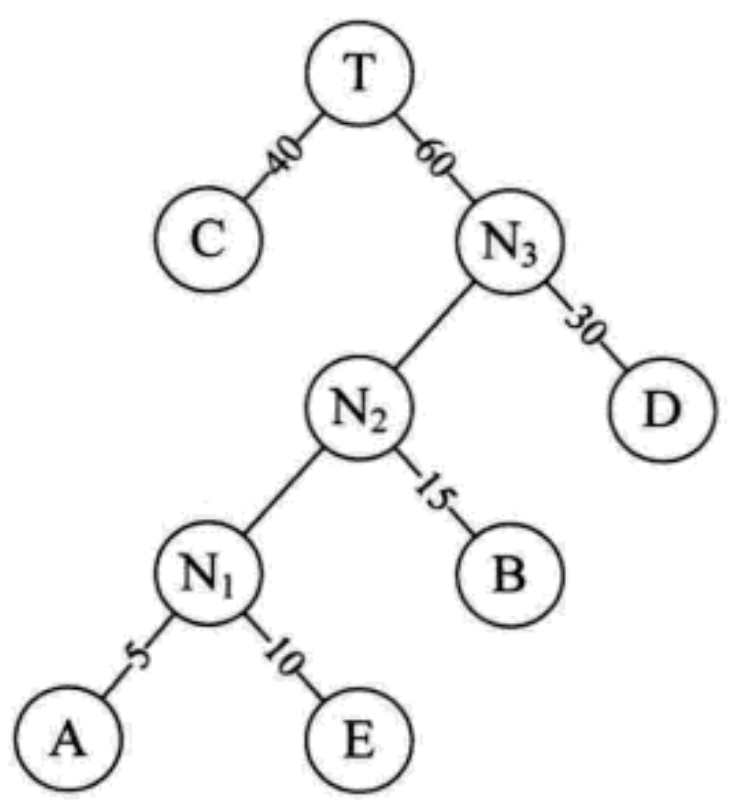WPL=40 * 1 + 30 * 2 + 15 * 3 + 10 * 4 + 5 * 4 = 205, 比搜索二叉树还少了15, 因此这才是最优的赫夫曼树

#### 3.4.3 赫夫曼编码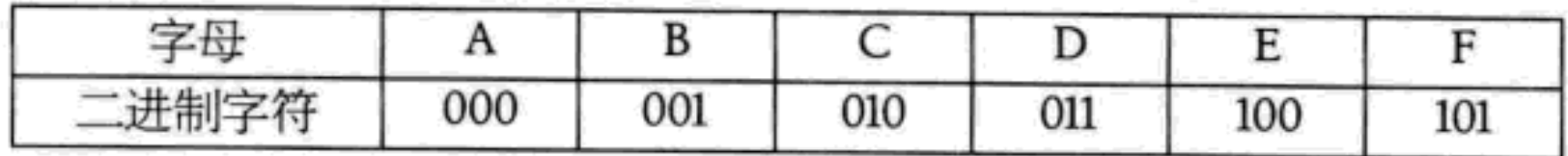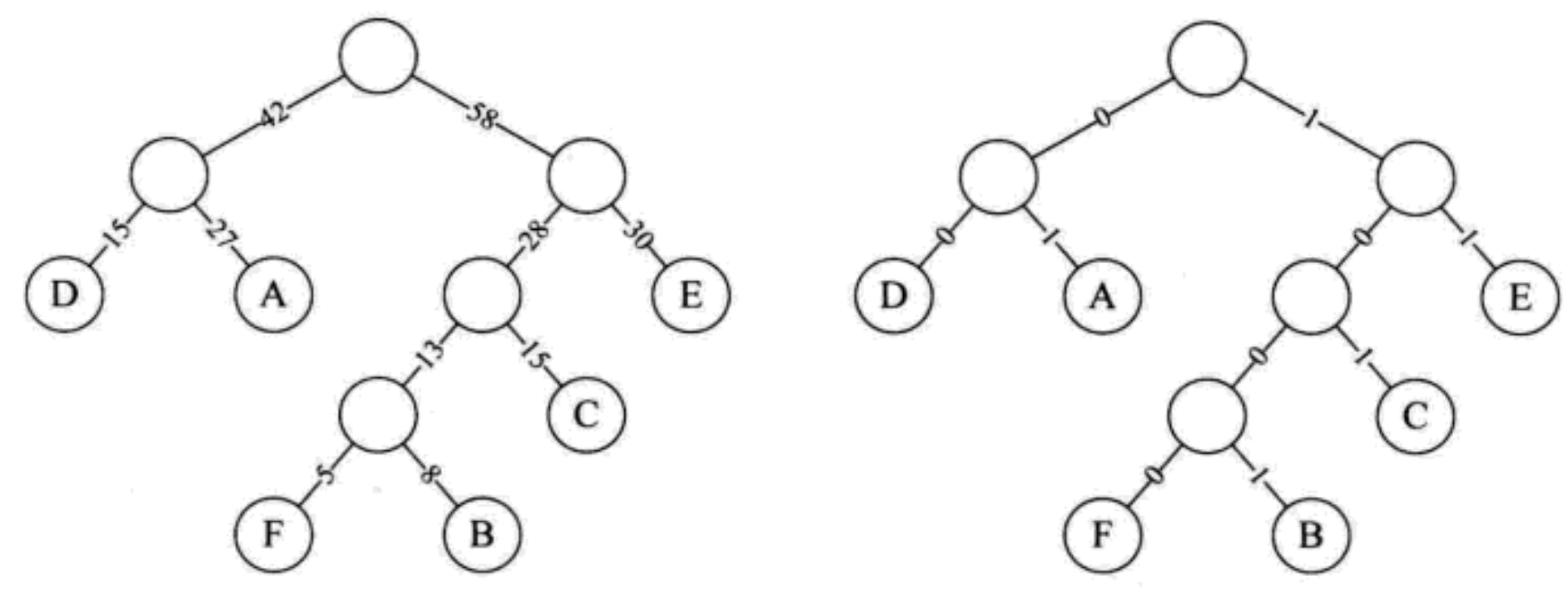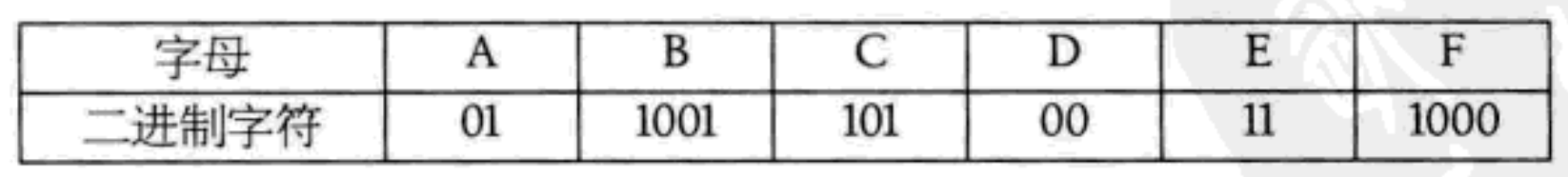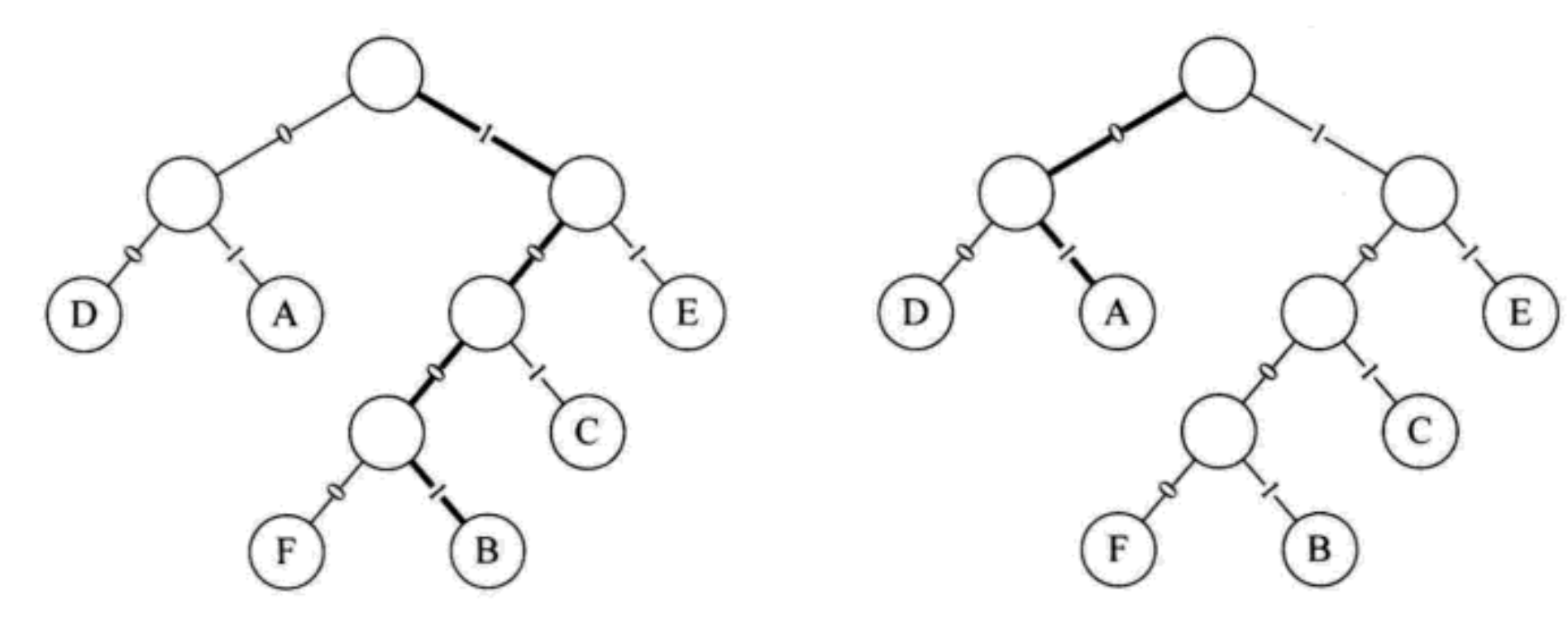### 3.4.3 线索二叉树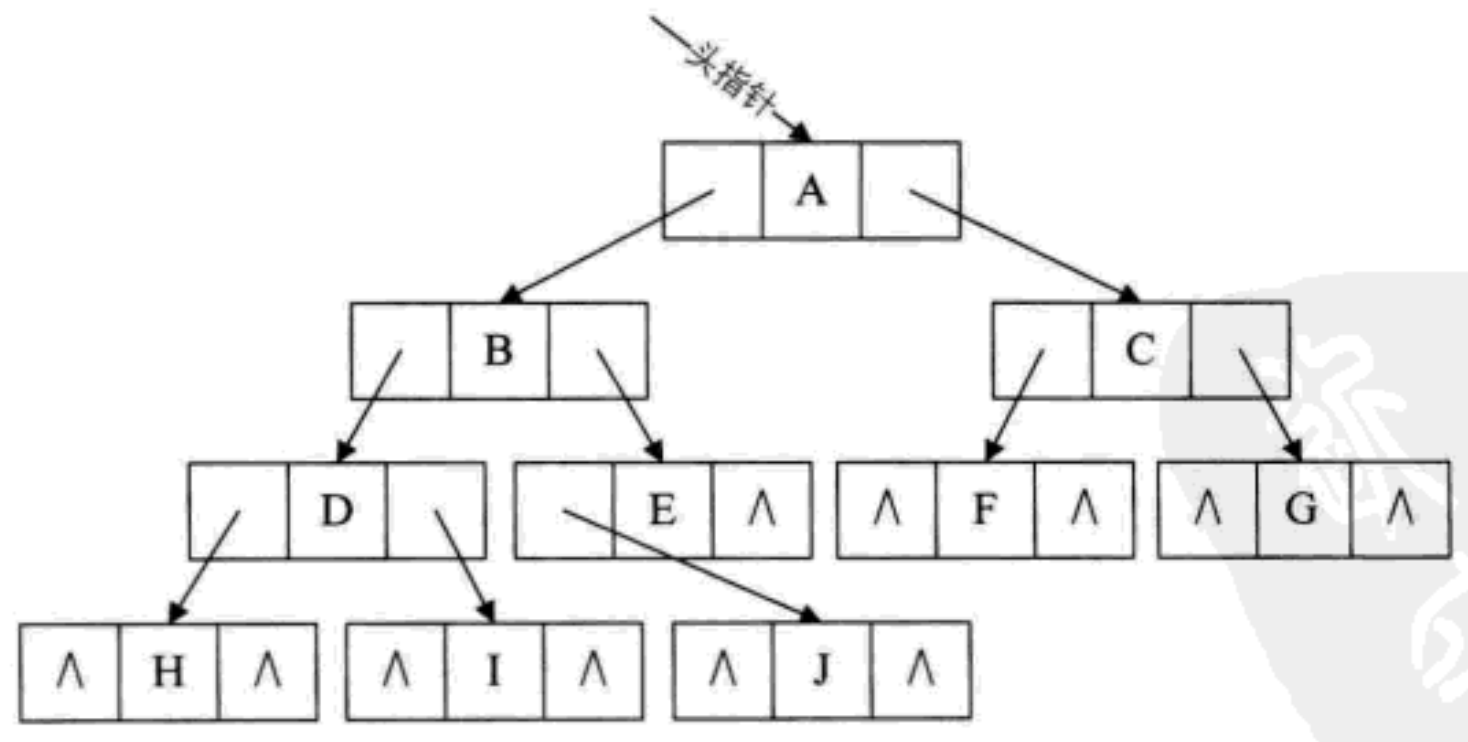^: 表示空指针, 图中二叉树有许多空指针, 发现这些空间都没有利用. 这其实不是一个好现象, 应该合理利用起来.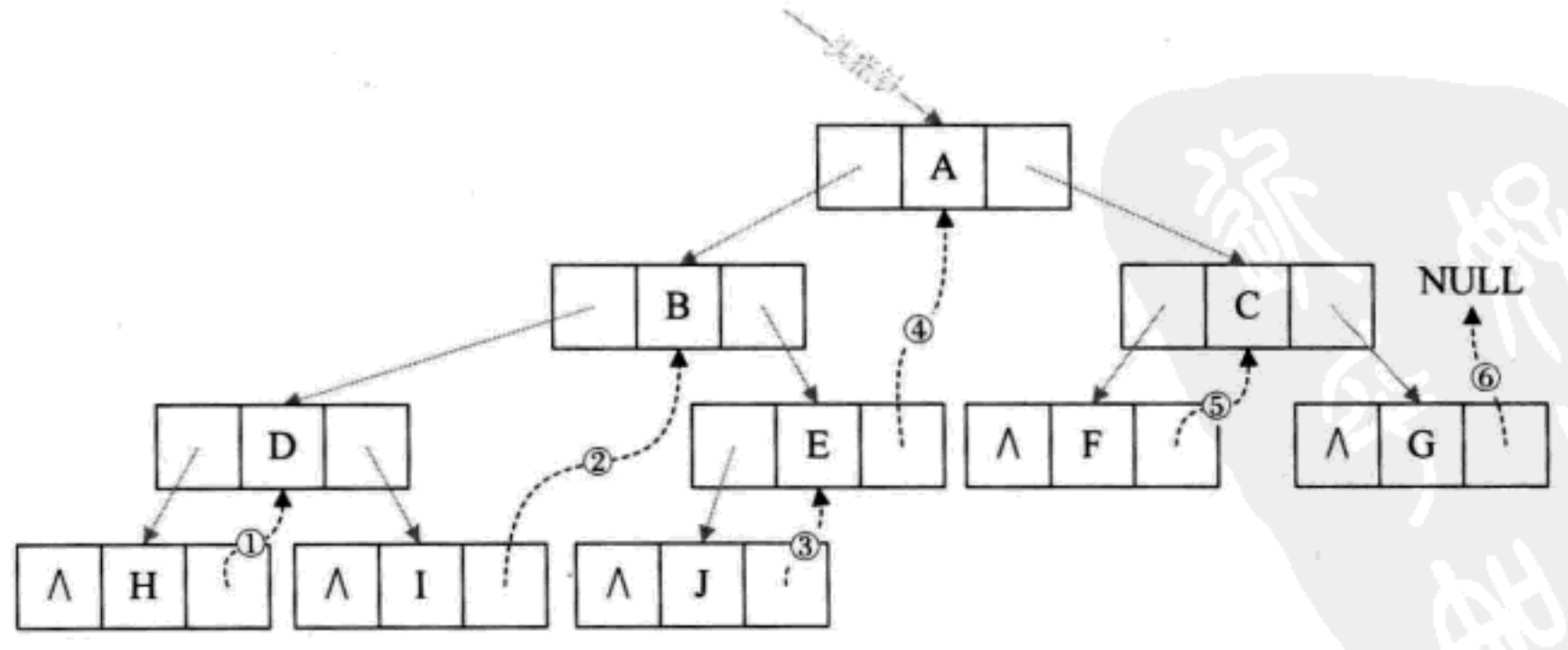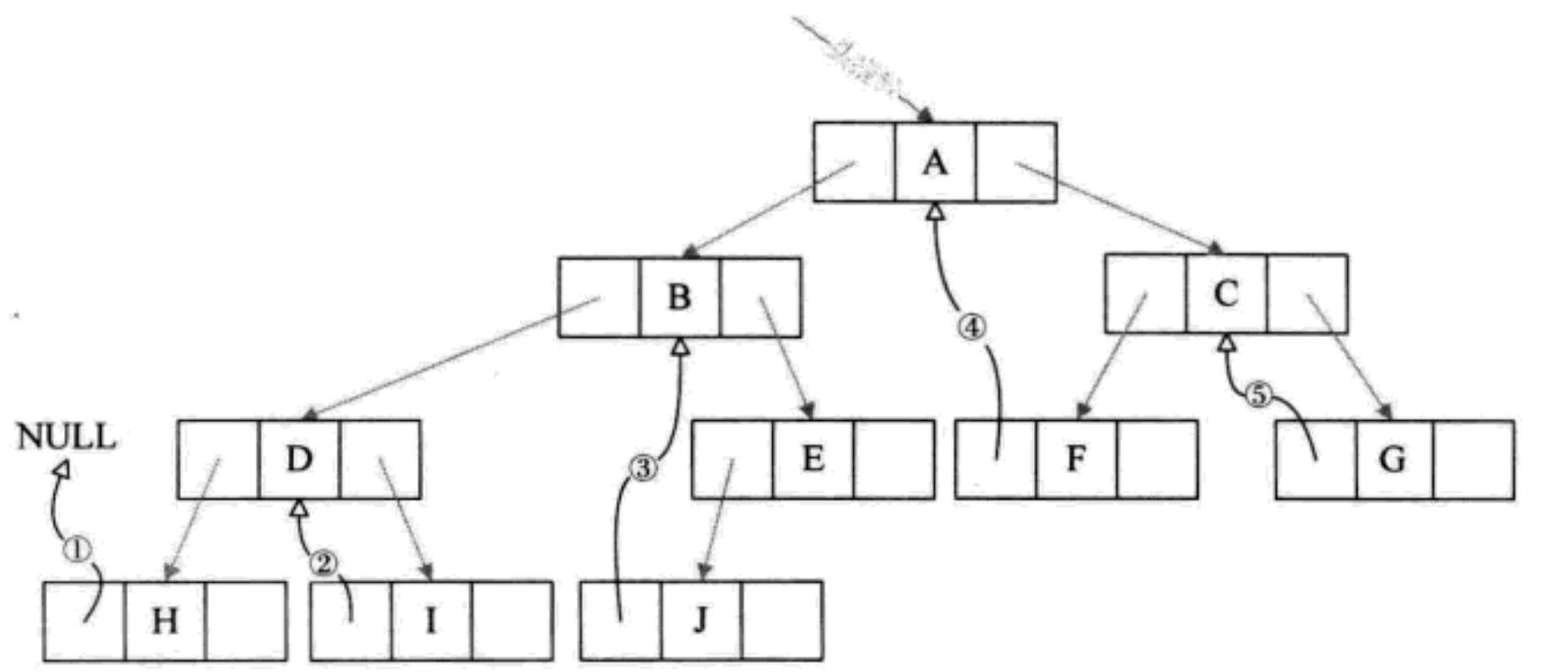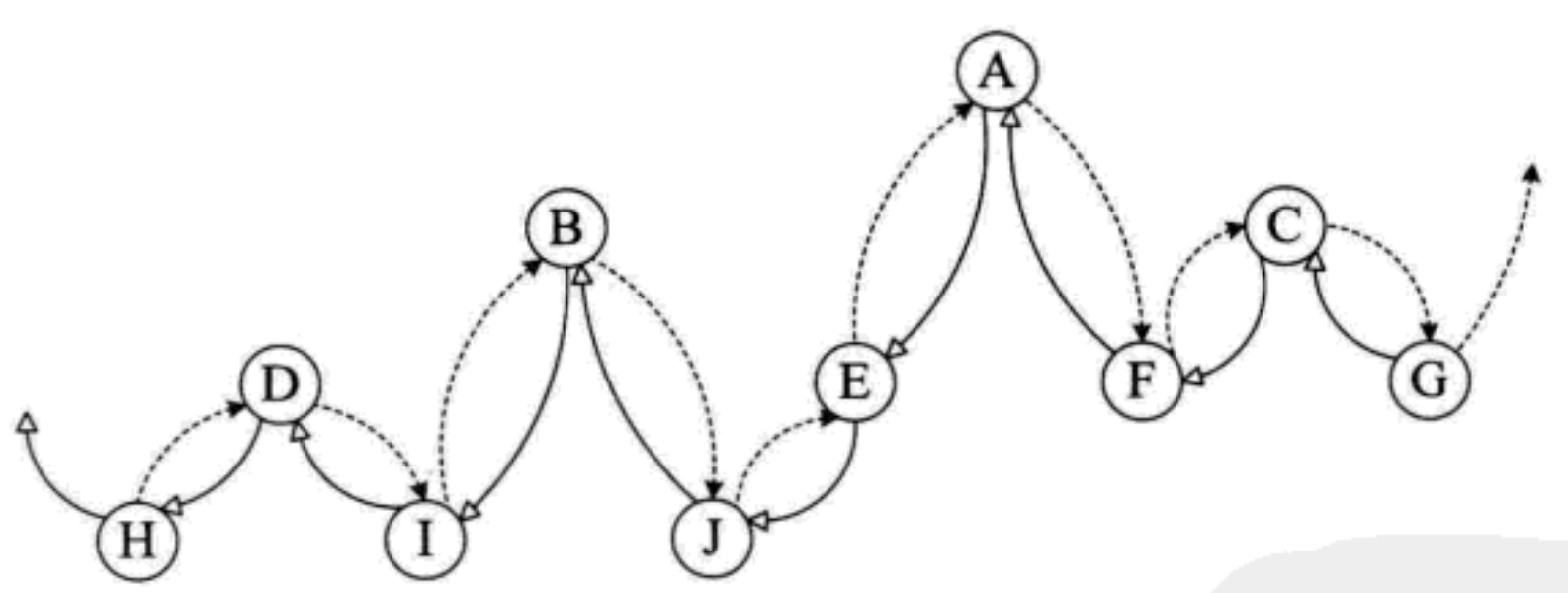OJ题目练习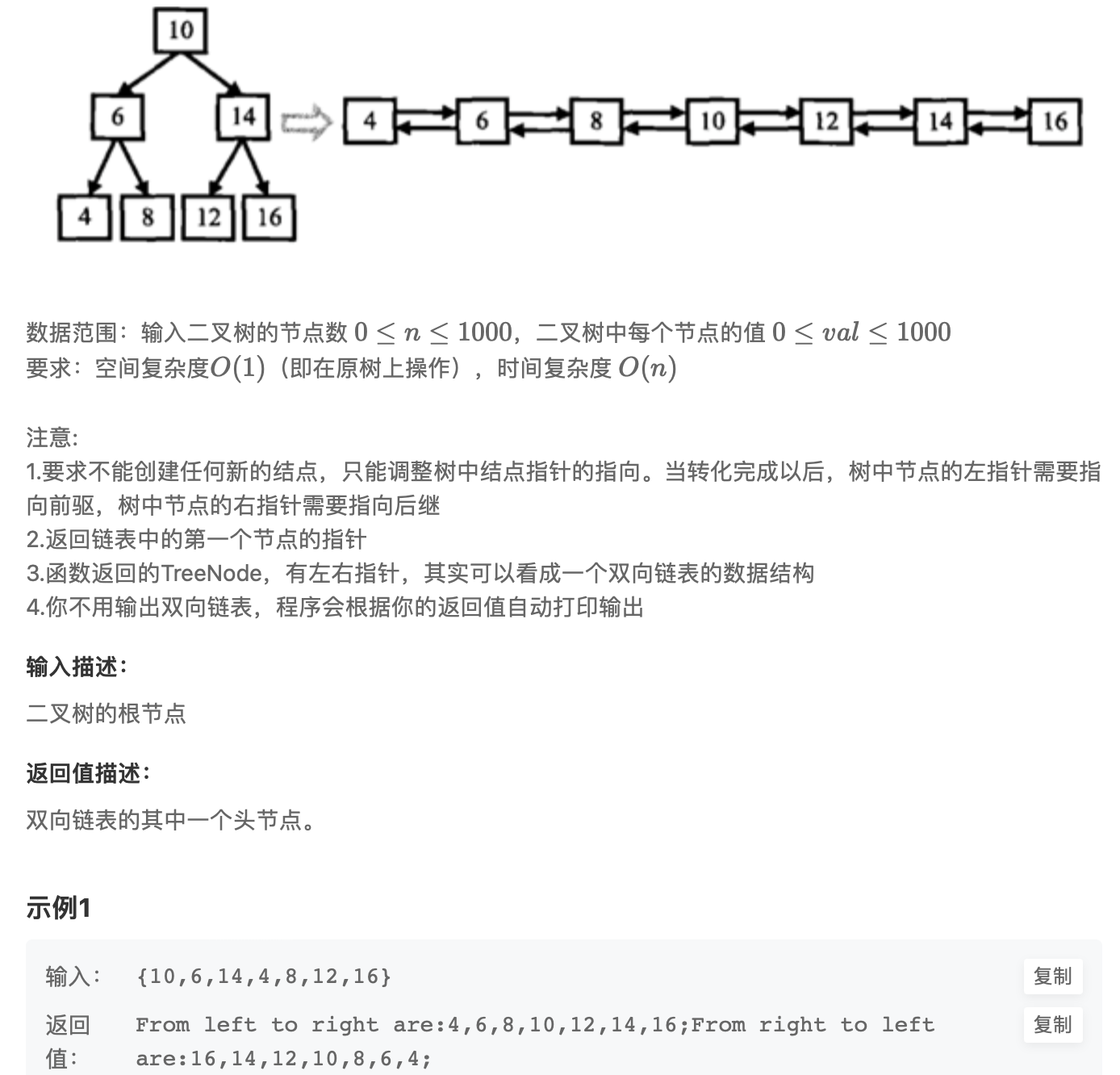Java: 构建代码

``````/**
public class TreeNode {
int val = 0;
TreeNode left = null;
TreeNode right = null;

public TreeNode(int val) {
this.val = val;

}

}
*/
public class Solution {
private TreeNode prev = null;// 1. 用来记录前驱节点
private void ConvertChild(TreeNode root){
if(root == null){
return;
}else{
// 2.根绝二叉搜索树性质: 中序遍历就是有序结果
ConvertChild(root.left);
/*
3.建立前后指向
节点的左子树是前驱, 右子树是后继
对于最左端叶子结点 4 这种没有左右子树的结点要特殊考虑: 保留的前驱节点的右子树指向当前函数调用的节点【4.right = 6】, 但对于这种情况还需要判断它是否为 null, 否则就会 空指针异常
4.left = null
null.right = 4;空指针异常
null = 4;

加上 if 判断后:
4.left = null;
null = 4;
程序 return 返回到 6节点函数
6.left = 4;
4.right = 6;
4 = 6;
*/
root.left = prev;// 第一次递归结束返回到 root 是4这个节点
if(prev != null){
prev.right = root;
}
prev = root;
ConvertChild(root.right);
}
}

public TreeNode Convert(TreeNode pRootOfTree) {
if(pRootOfTree == null){
return null;
}else{
ConvertChild(pRootOfTree);
while(pRootOfTree.left != null){
pRootOfTree = pRootOfTree.left;
}
return pRootOfTree;
}
}
}
``````

Java 语言在定义节点类的时候只需要: 数据域val, 左子树left, 右子树right, 在实现双向链表的时候需要额外一个 prev节点来存放当前节点的前驱【左子树】C: 线索二叉树结构体思路
lchild: 左子树, rchild: 右子树

lchildltagdatartagrchild
• ltag == 0时指向该节点的左孩子, 为1时指向该节点的后继
• rtag == 0时指向该节点的右孩子, 为1时指向该节点的后继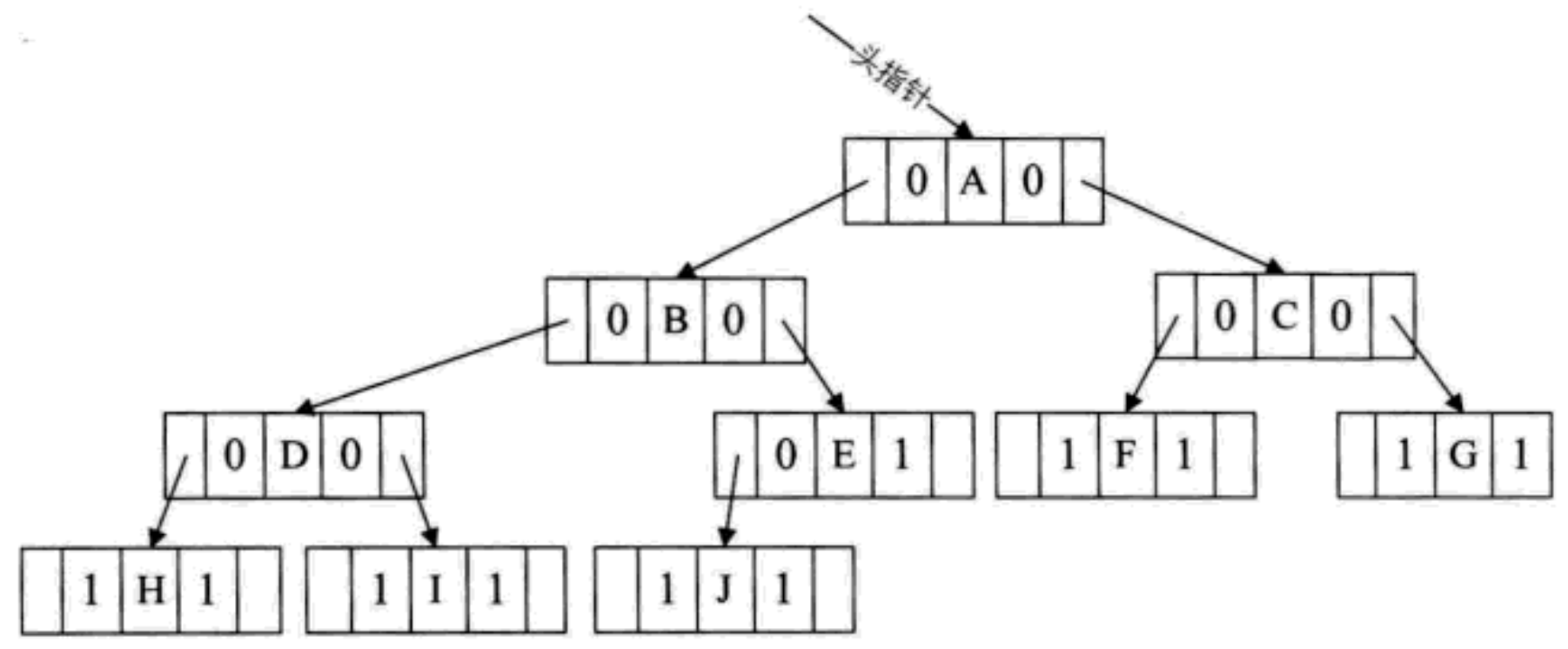``````typedef int DataType;// 数据类型

typedef enum {
} PointerTag;

typedef struct BiThrNode {// 二叉树存储节点结构
DataType data;// 数据域
struct BiThrNode *lchild, *rchild;// 左右子树
PointerTag  LTag;// 左标志
PointerTag  RTag;// 右标志
}BiThrNode, *BiThrTree;
``````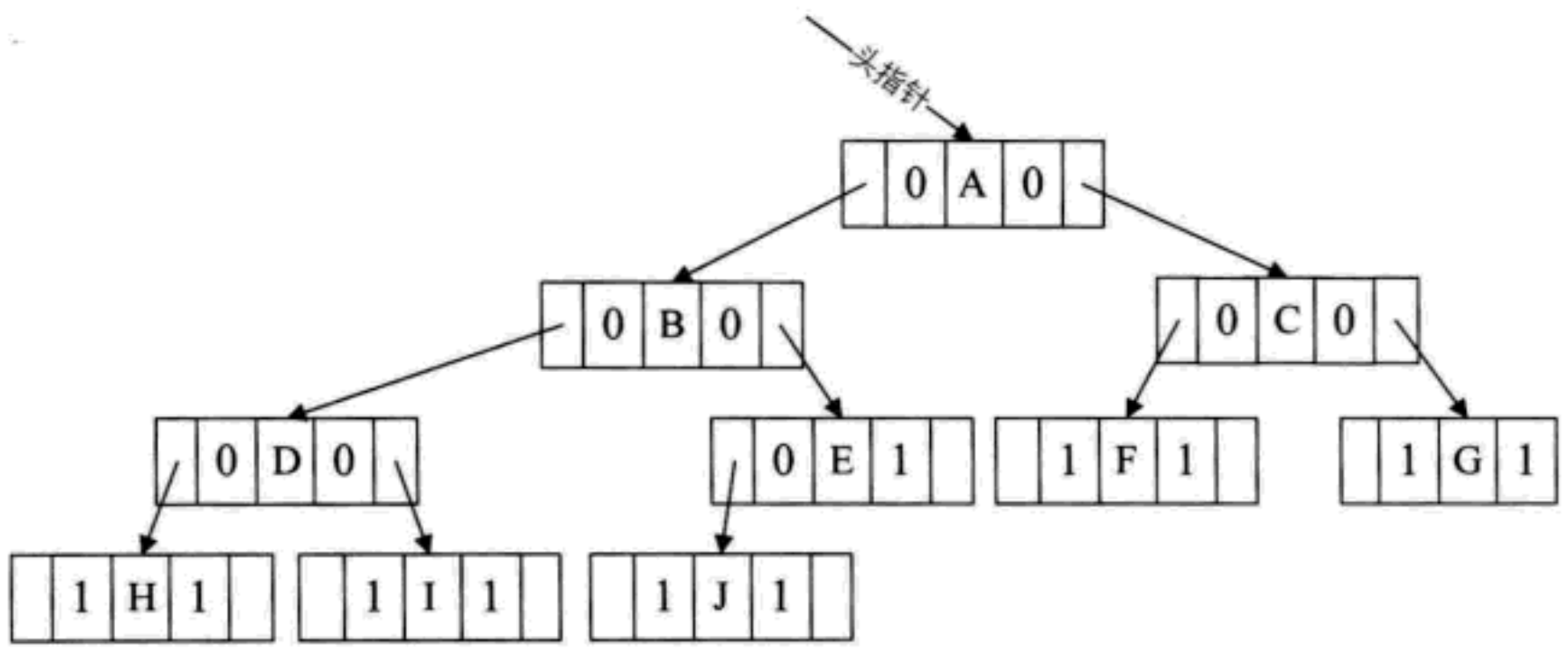``````BiThrNode *prev;// 全局变量, 始终指向刚刚访问过的节点
if (pb) {
/*
* 中序遍历线索化二叉树递归函数
*/
// 1. 左

/*
* 2.中间部分建立联系
*      因为中序遍历之后第一个元素就是 链表的头节点, 所以应该选取左节点为空的节点就是 链表头节点
*
* 前驱分析:
*      if(!pb->lchild): 表示如果某结点的左子树域为空, 因为其前驱节点刚刚访问过
*      赋值给了 prev, 所以可以把 prev 赋值给 pb->lchild, 并修改 pb->Ltag=Thread.完成了前驱节点的线索化
* 后继分析:
*       此时 pb 节点的后继还没有访问到, 只能通过它的前驱节点 prev 的右子树 rchild 来判断:
*              if(!prev-rchild)为空, 则 pb 就是 prev 的后继, 于是 prev-Thread, prev->rchild = pb 完成后继节点的线索化
* 完成前驱和后继的判断后把 pb 给 prev, 便于下一次遍历
*/
if (!pb->lchild) {// 如果没有左孩子
pb->lchild = prev;// 左孩子指针指向前驱
}
if (!prev->rchild) {// 如果没有右孩子
prev->lchild = pb;// 右孩子指针指向后继
}
prev = pb;
// 3.右